# Selina Solutions Concise Maths Class 7 Chapter 2: Rational Numbers

Selina Solutions Concise Maths Class 7 Chapter 2 Rational Numbers provides students with the basic idea of the method of solving them. Rational numbers are those numbers which can be expressed as the result of dividing an integer by a non zero integer. The main aim of preparing solutions is to help students solve textbook problems without any difficulty. Students who are not able to clear doubts during class hours can clear them by referring to the solutions. Students can effortlessly download the Selina Solutions Concise Maths Class 7 Chapter 2 Rational Numbers free PDF, from the links available here.

Chapter 2 explains students about the introduction of rational numbers, representation of rational numbers on number line, comparing rational numbers and problems on rational numbers. Students can understand these concepts and improve time management skills by practicing the problems.

## Selina Solutions Concise Maths Class 7 Chapter 2: Rational Numbers Download PDF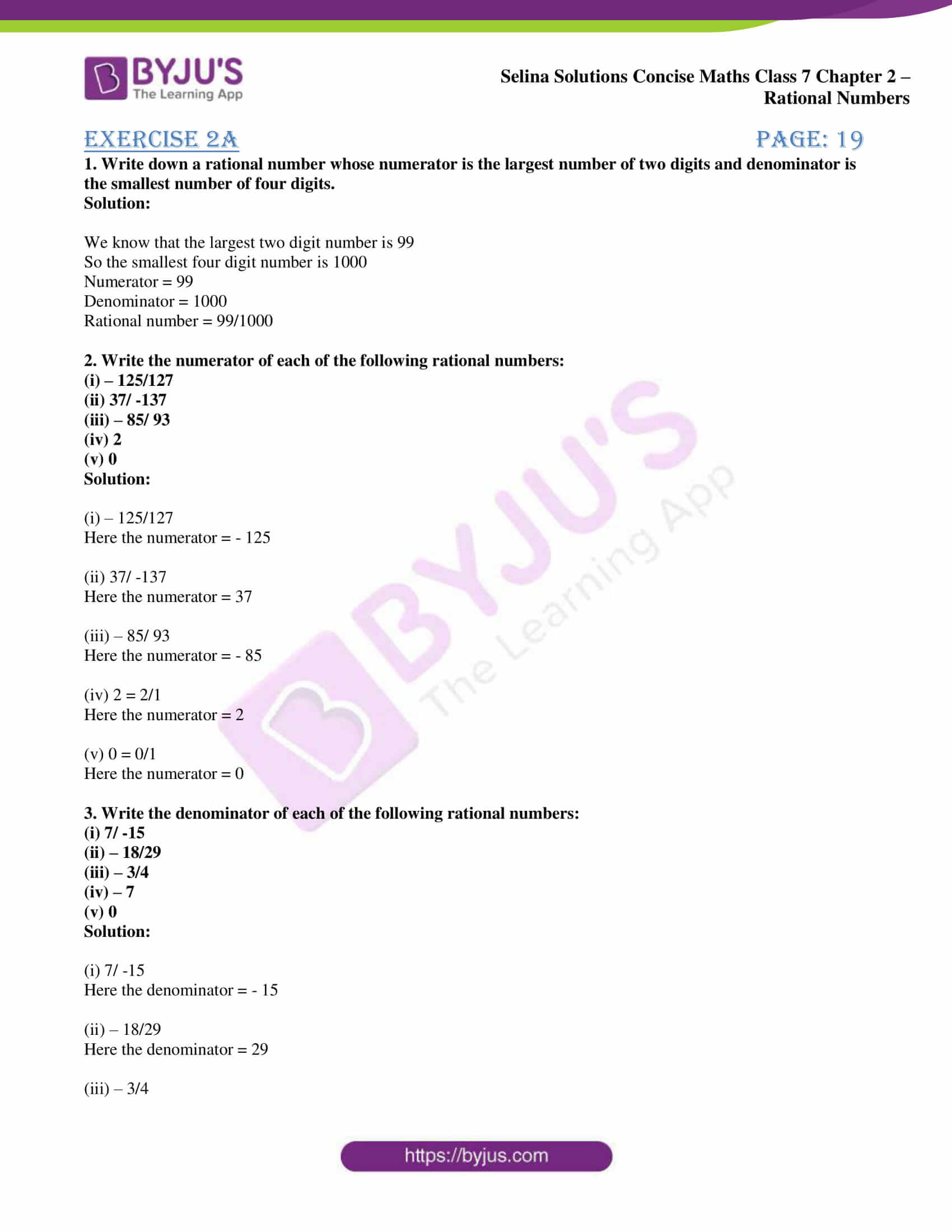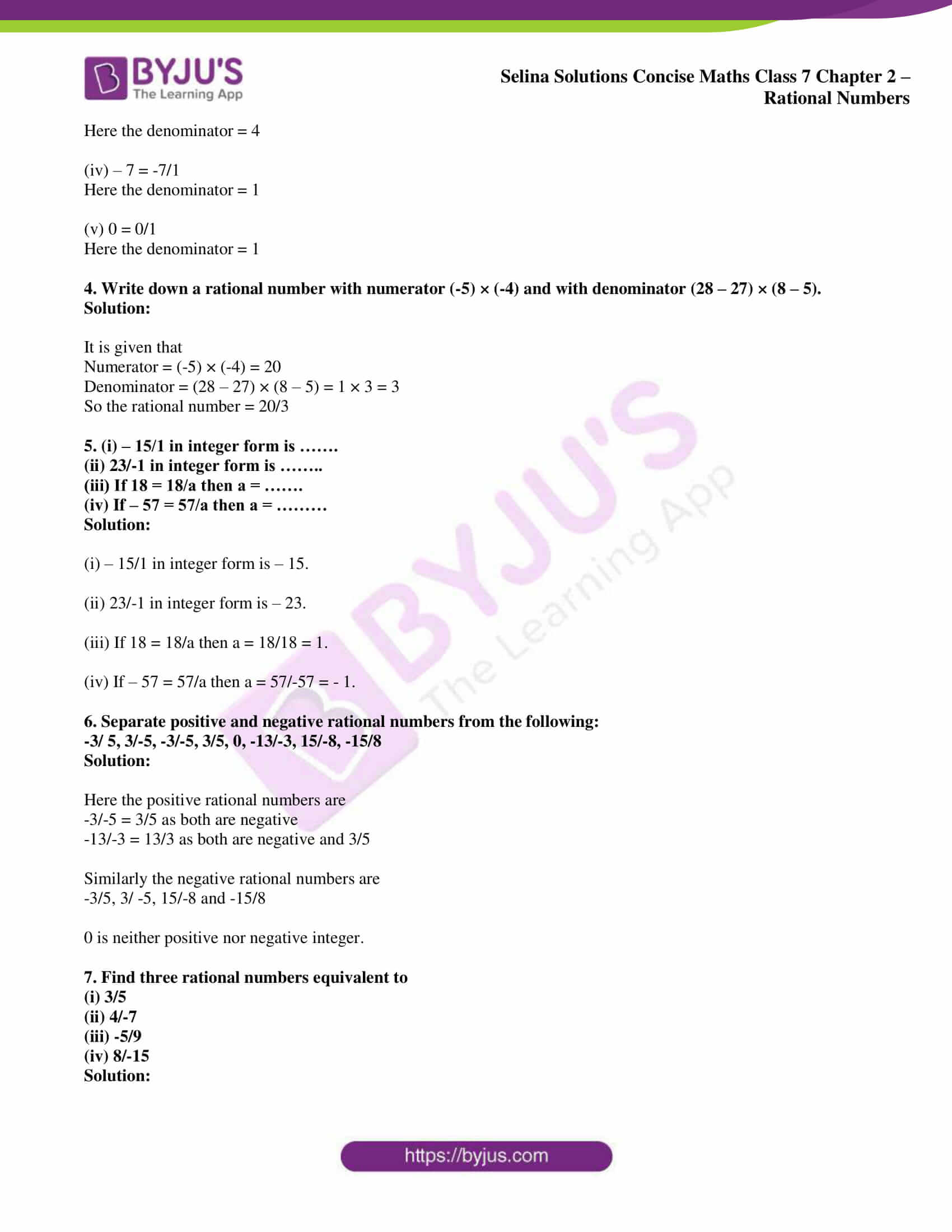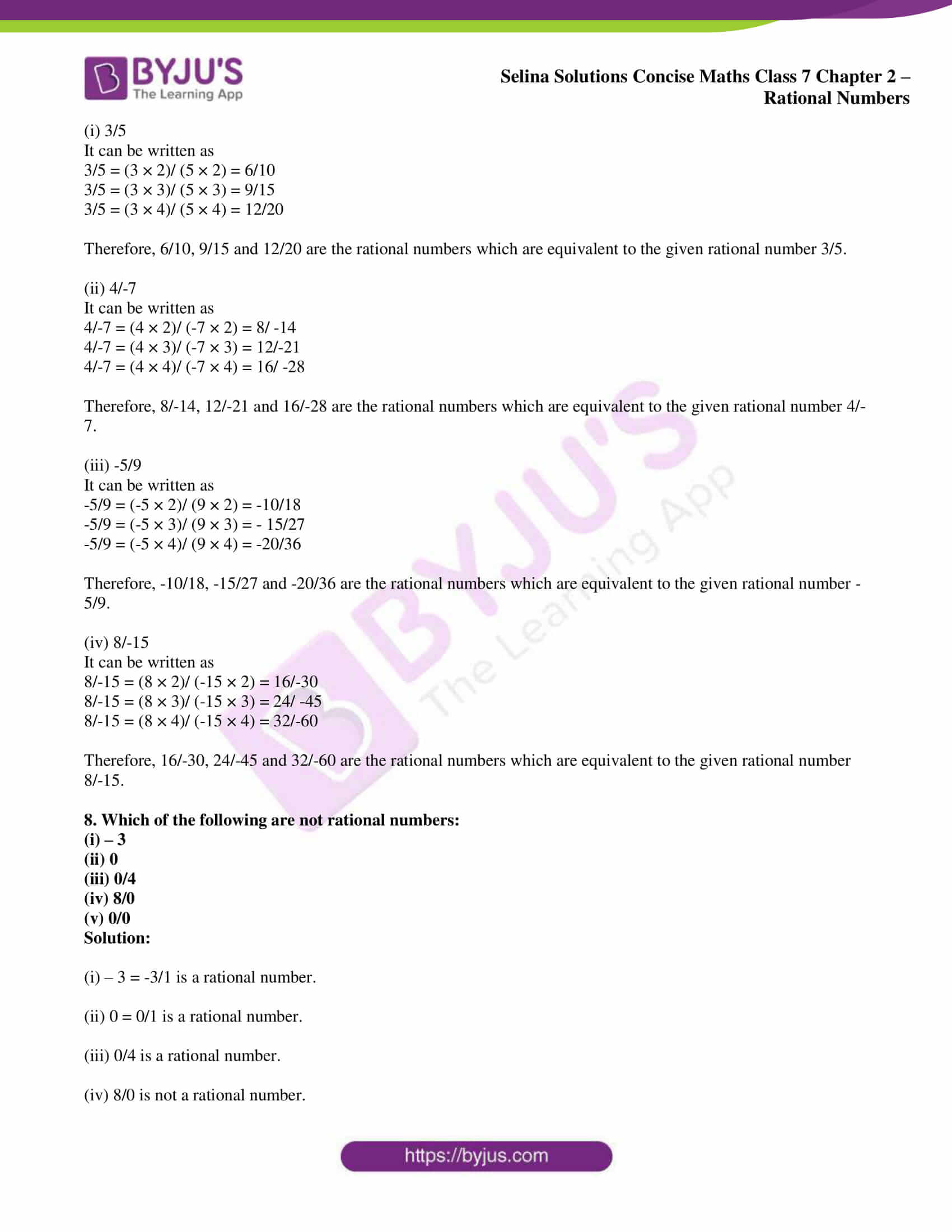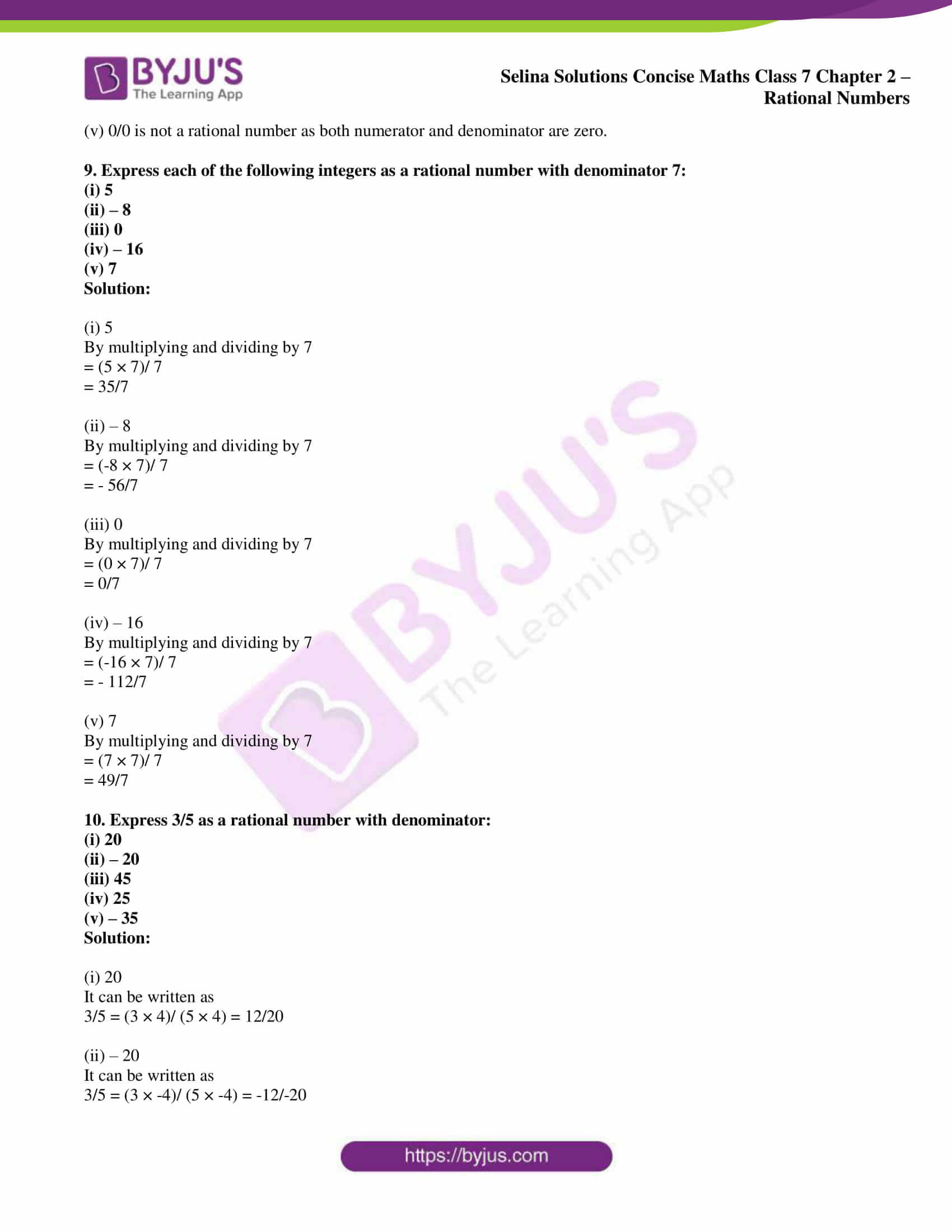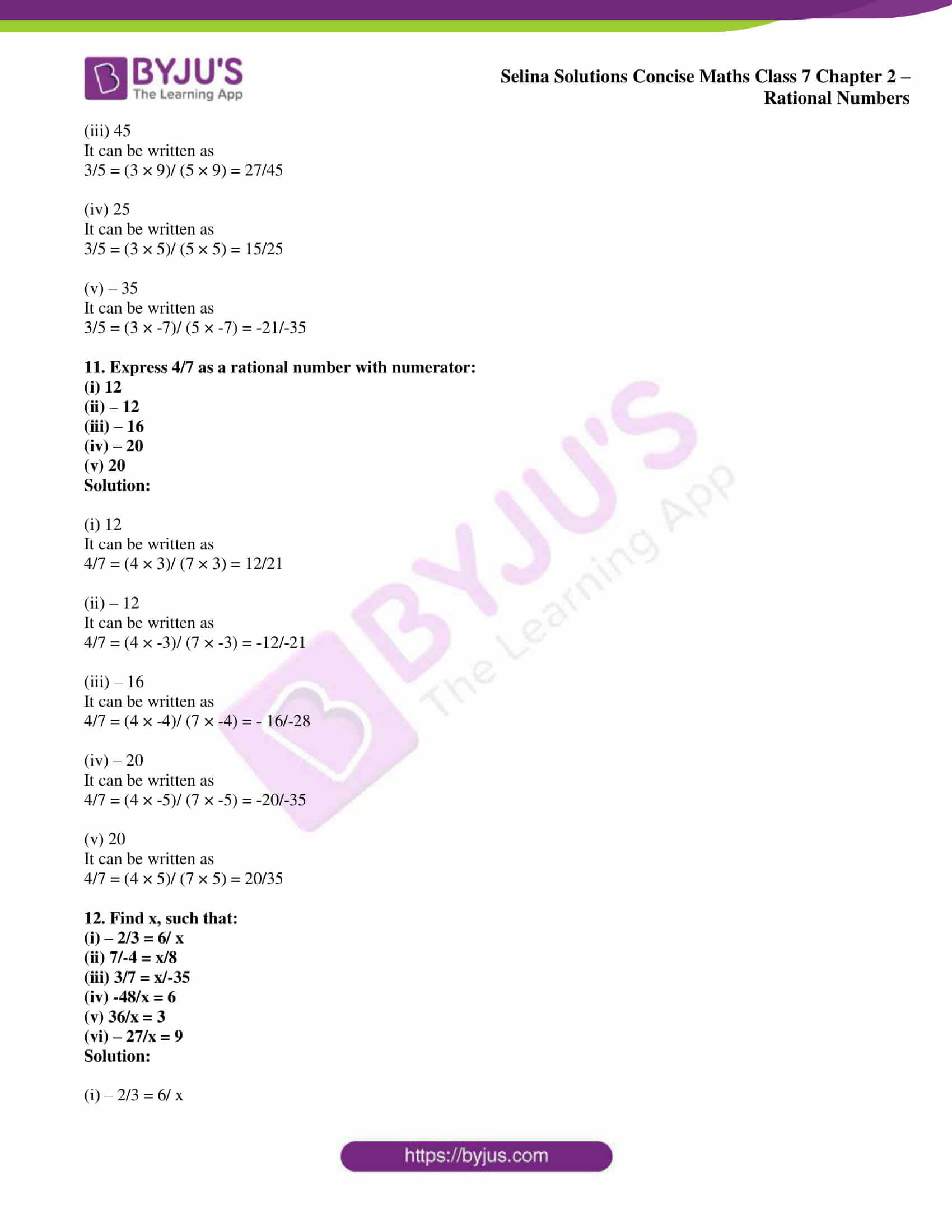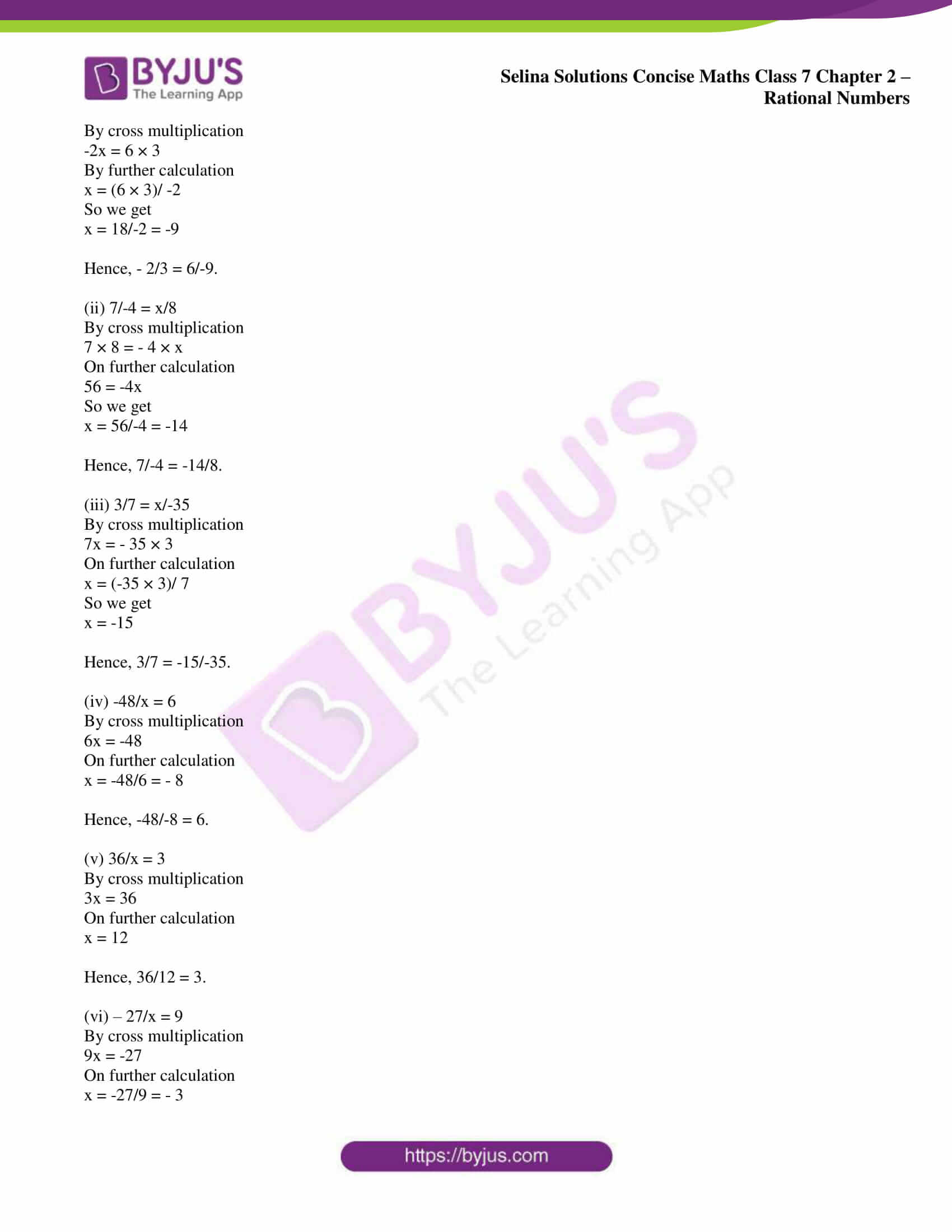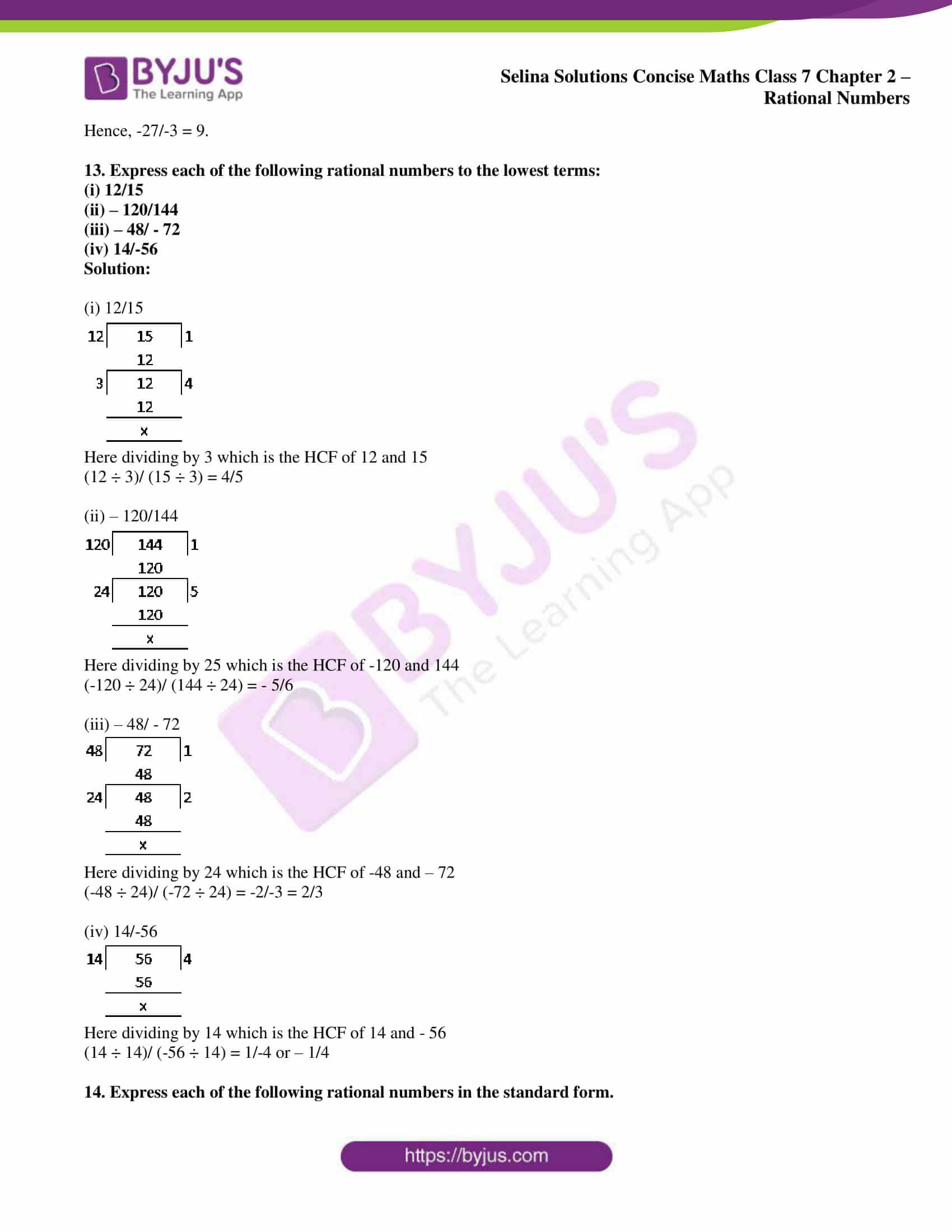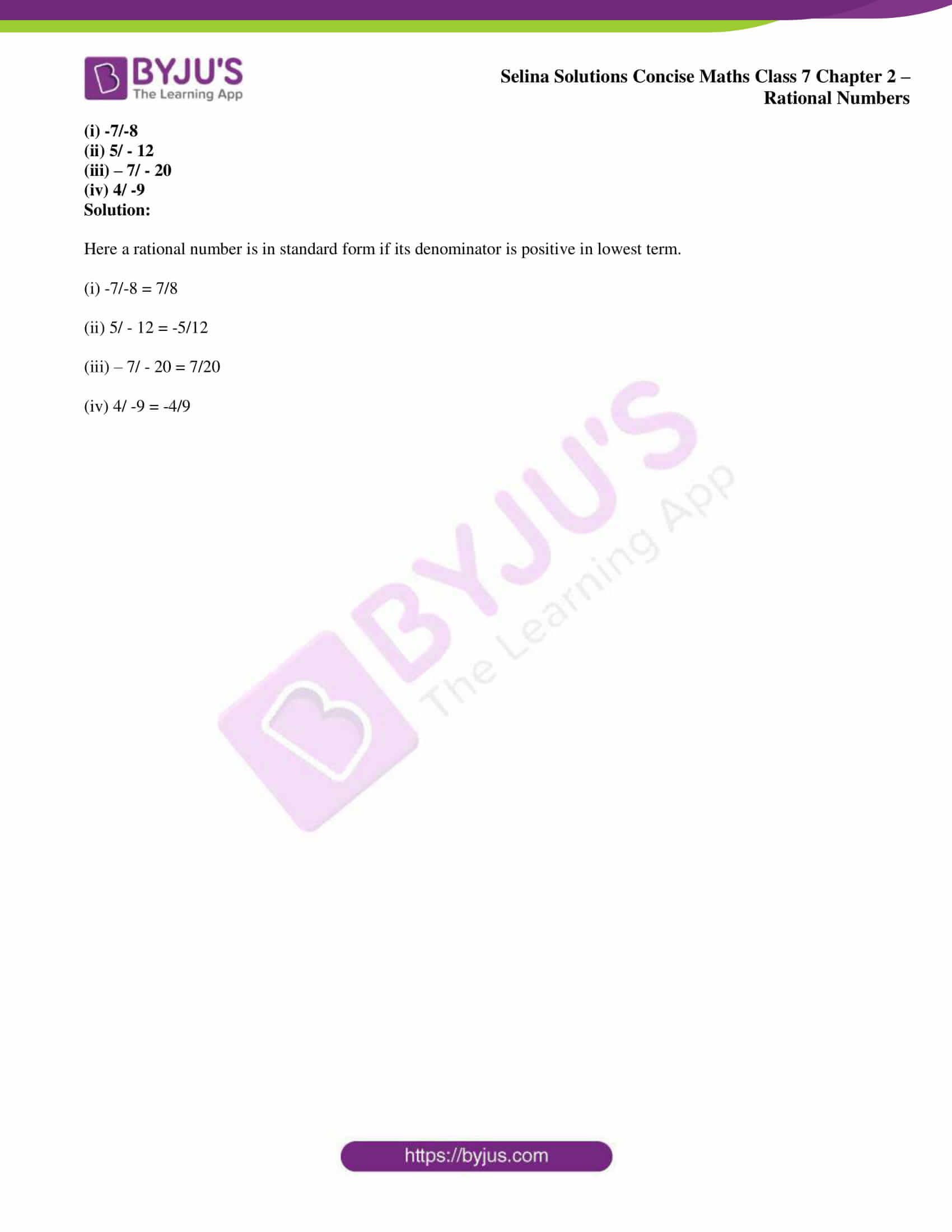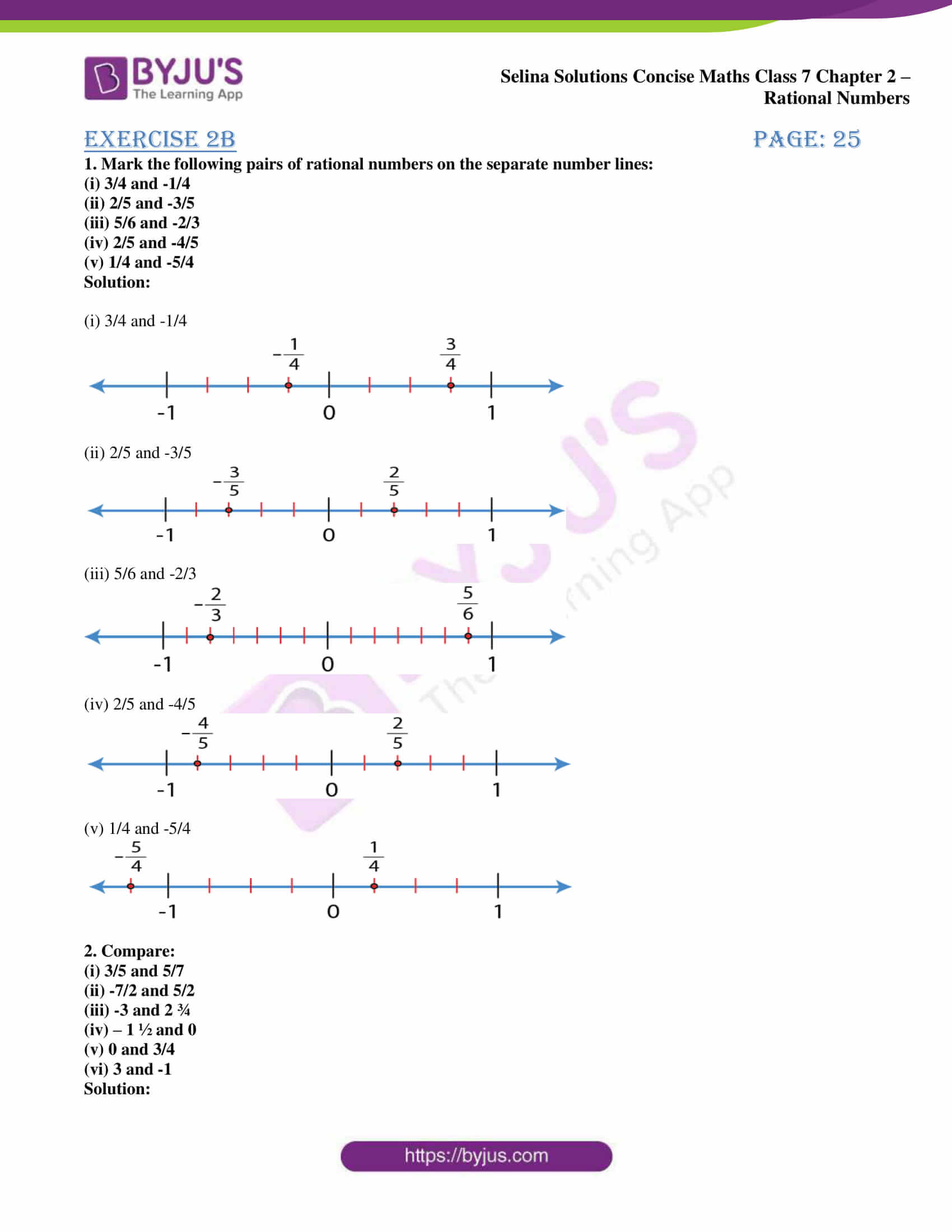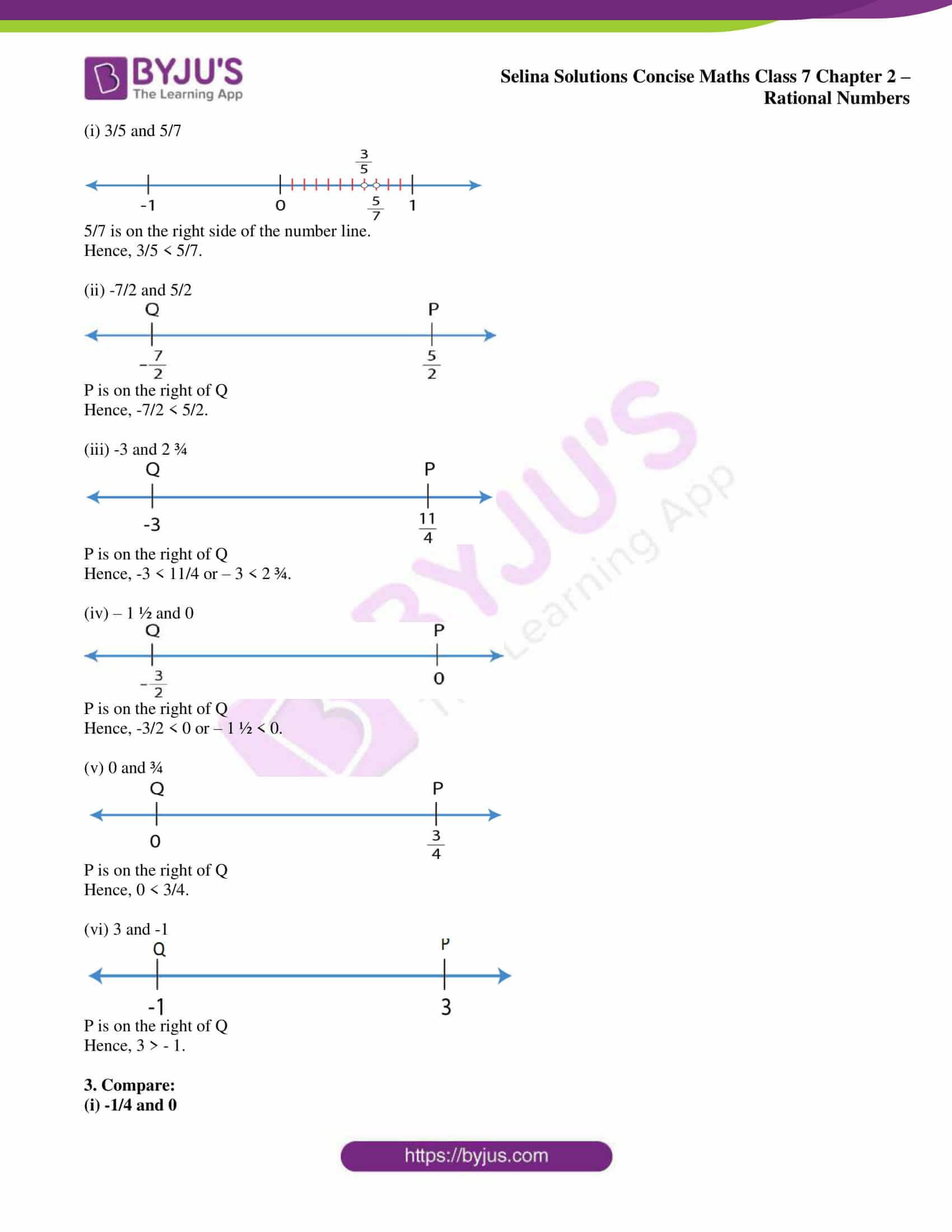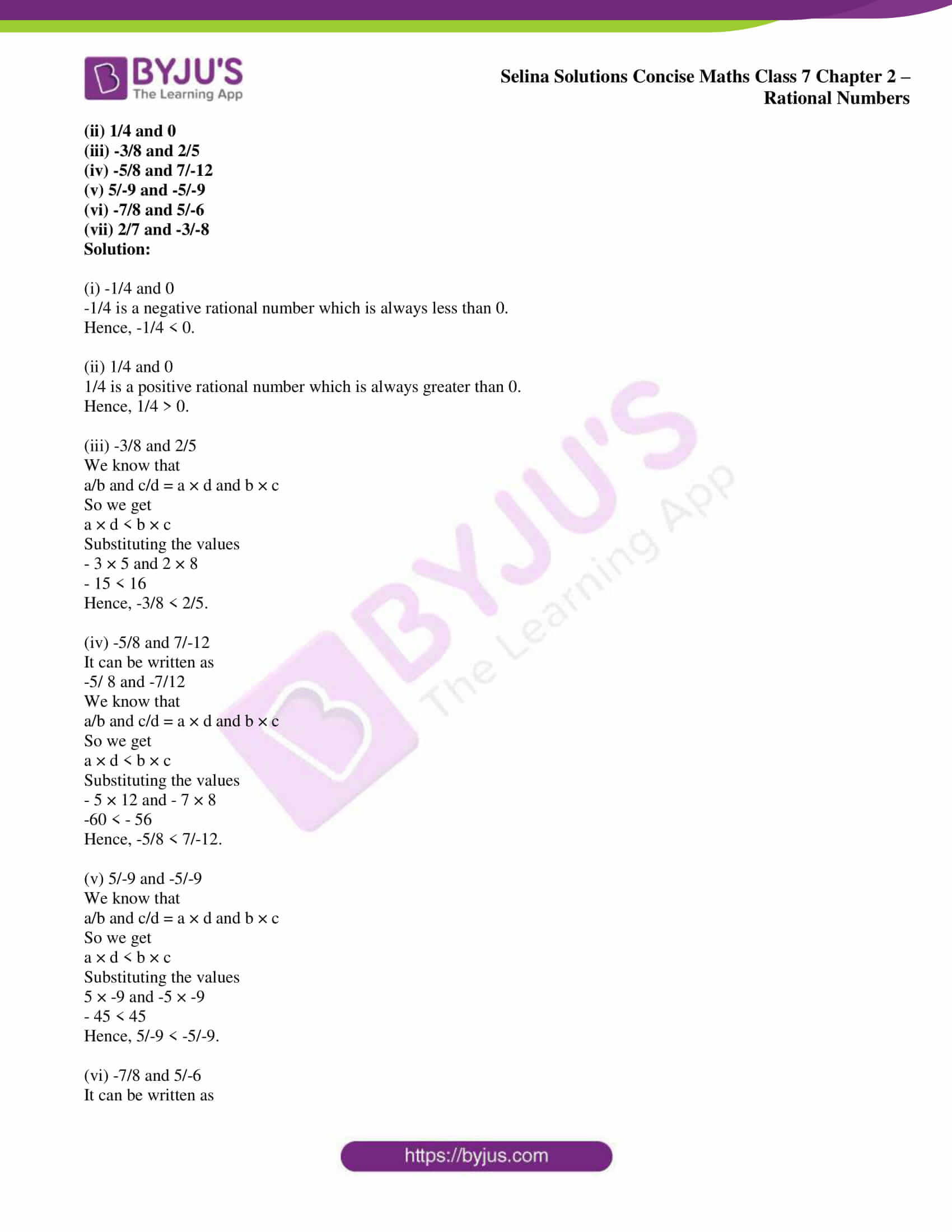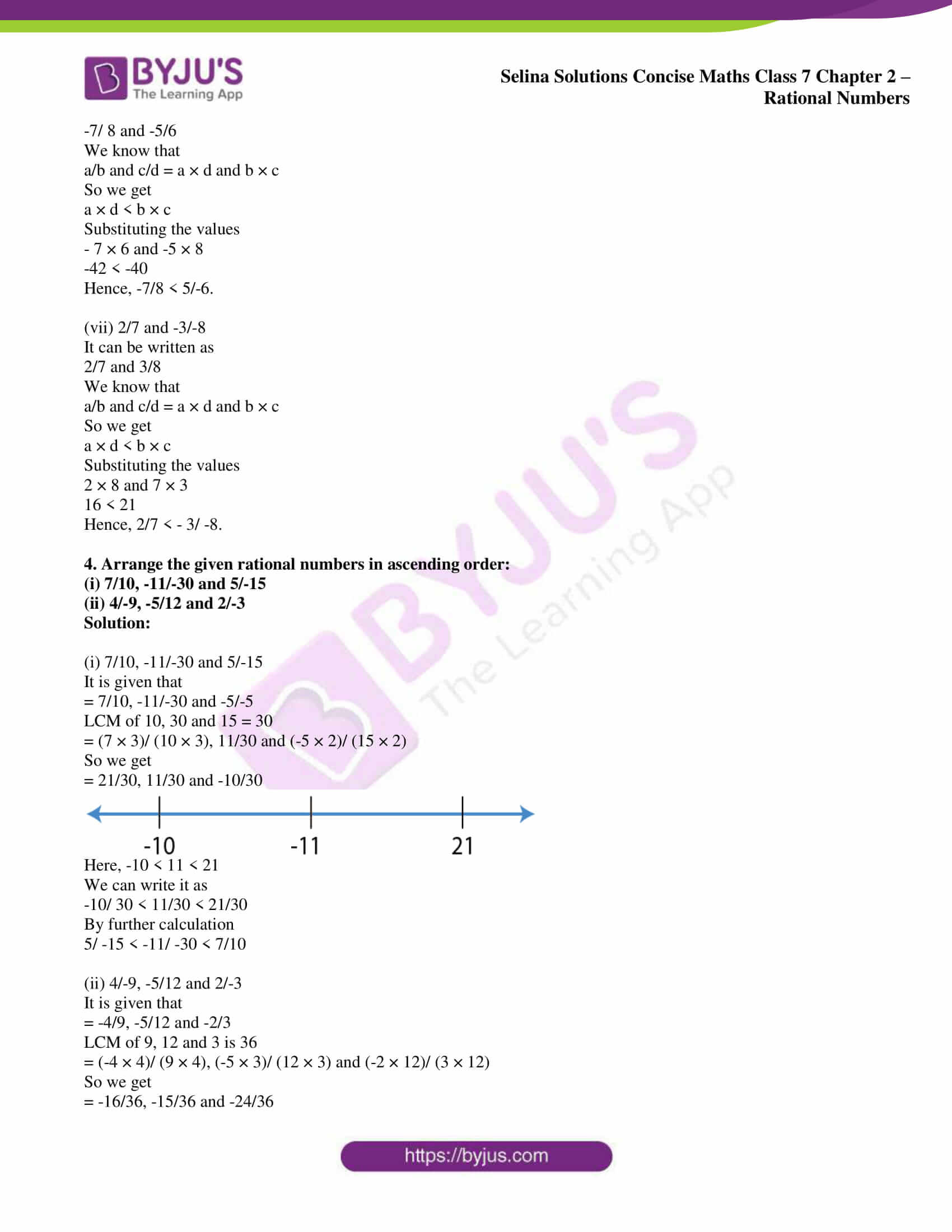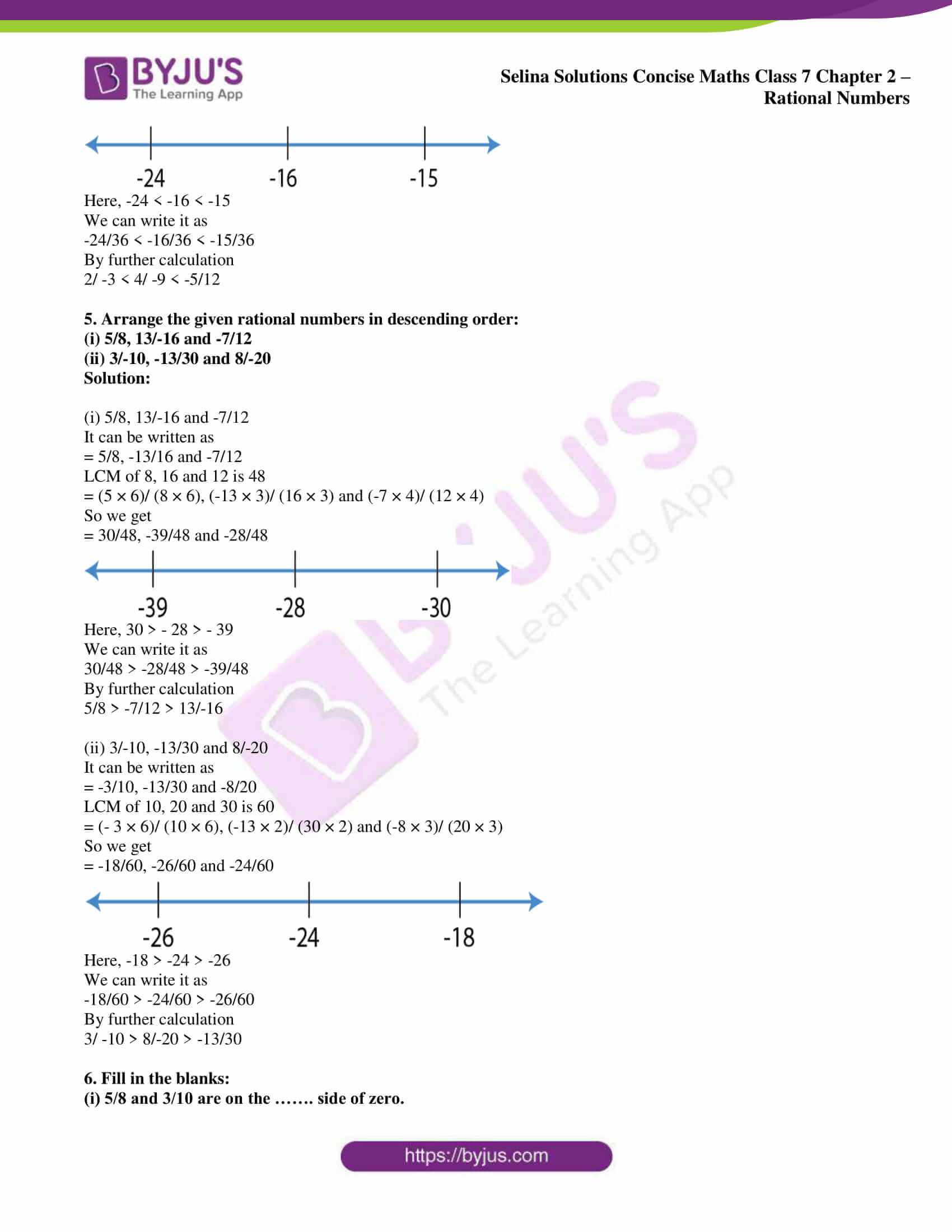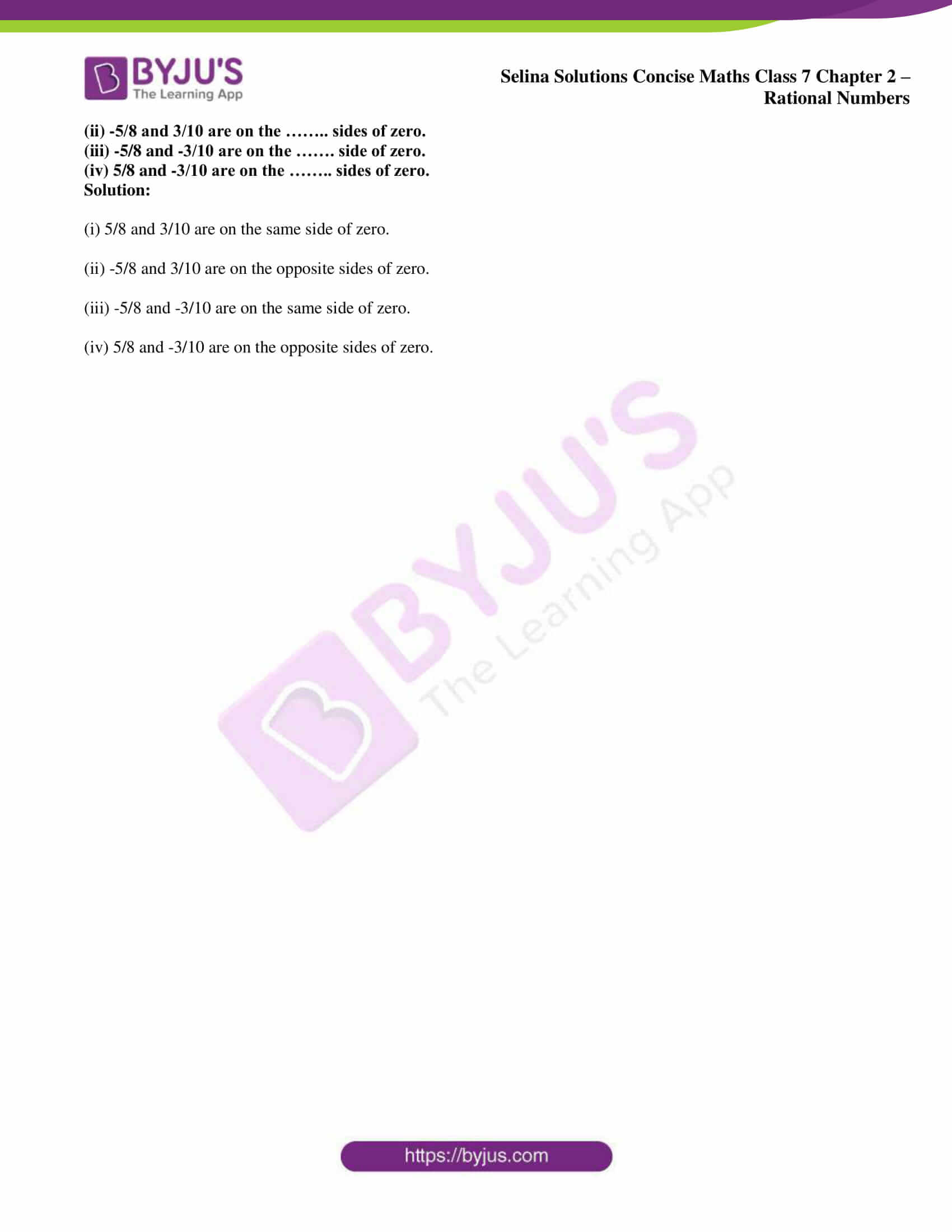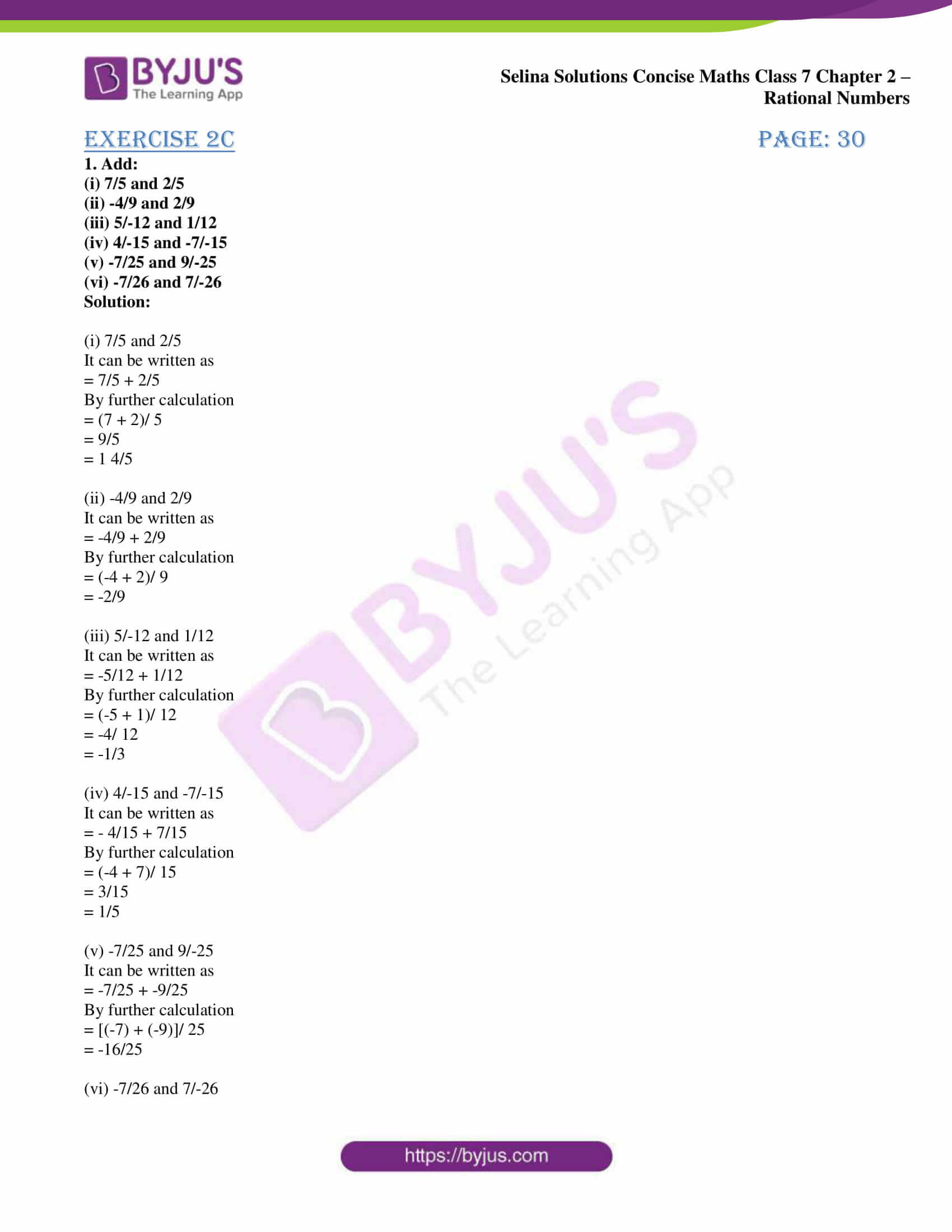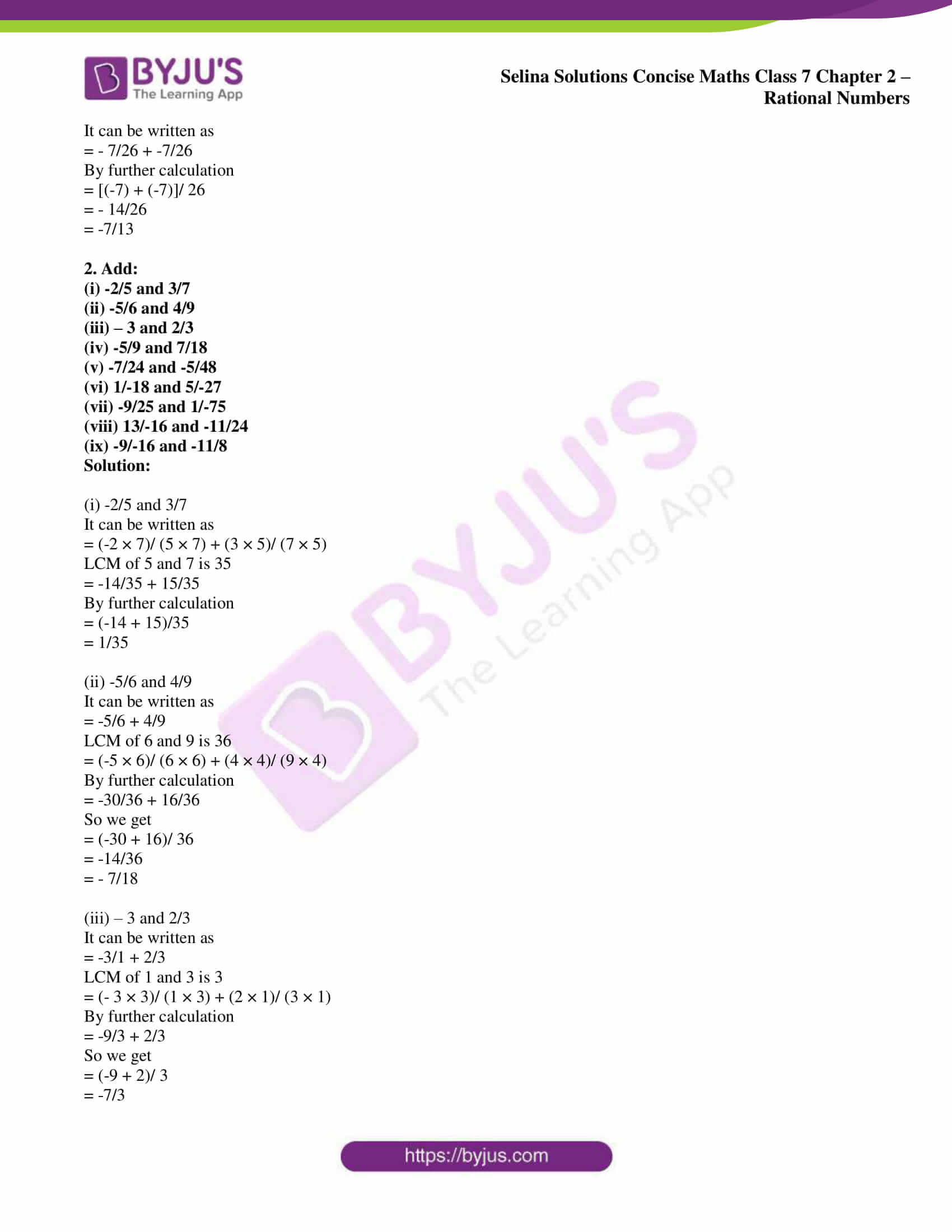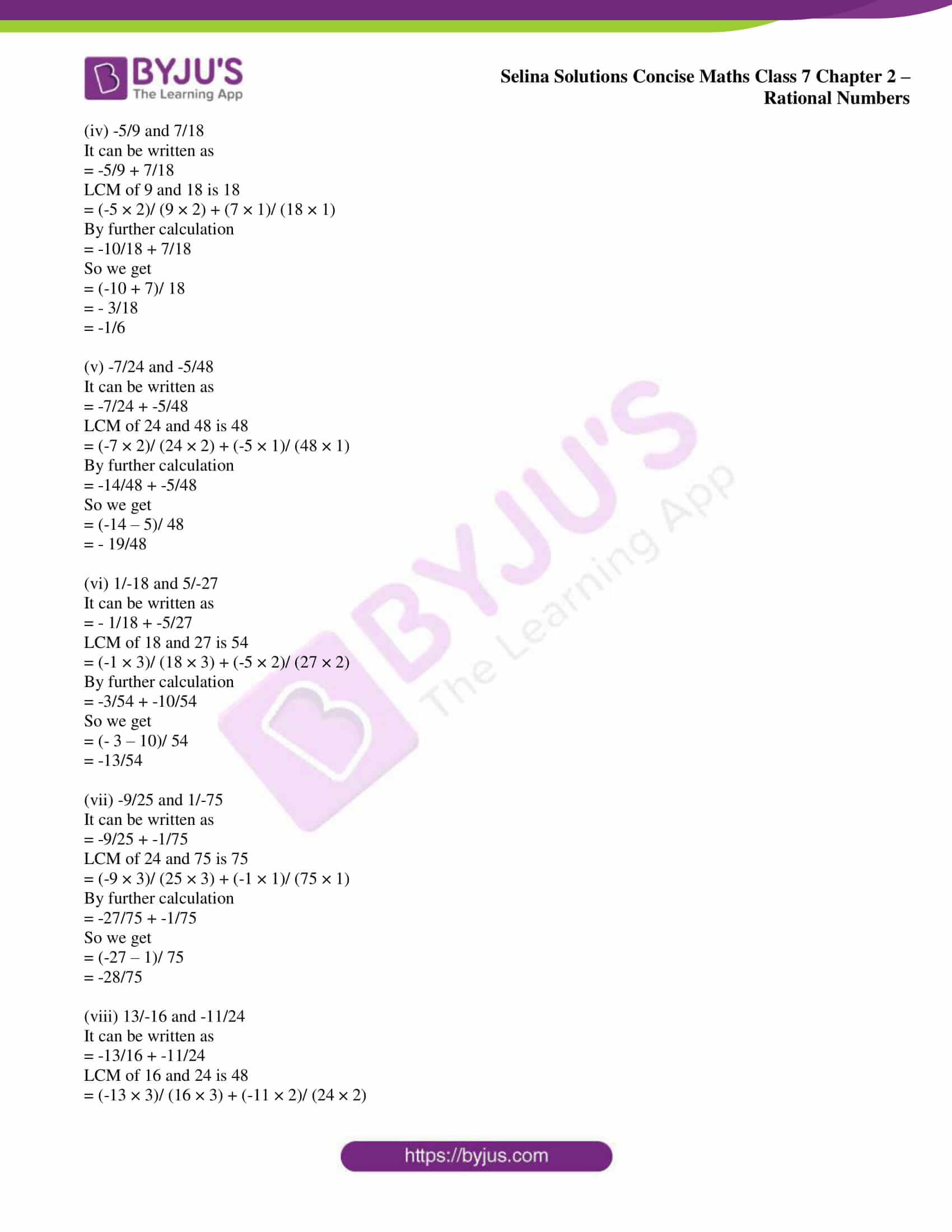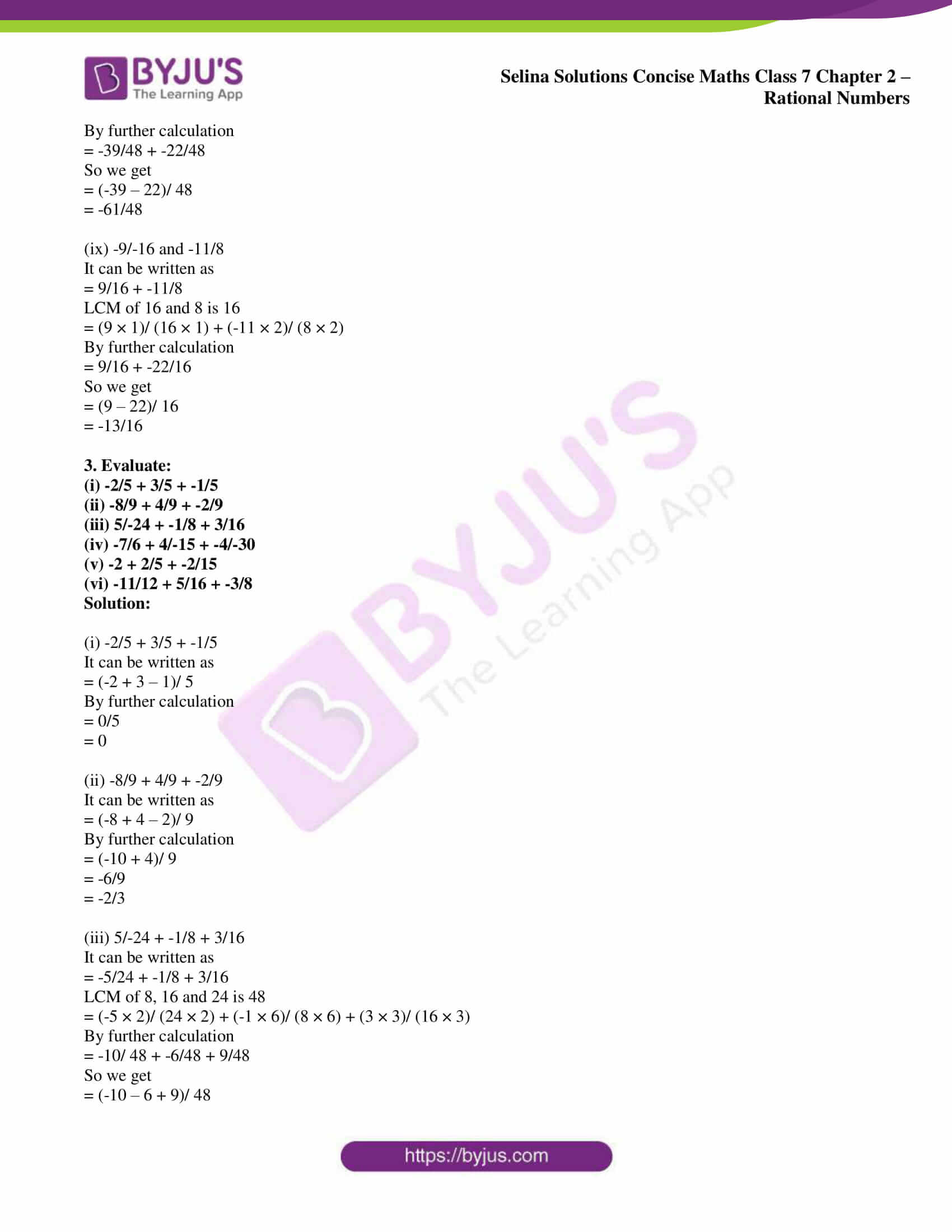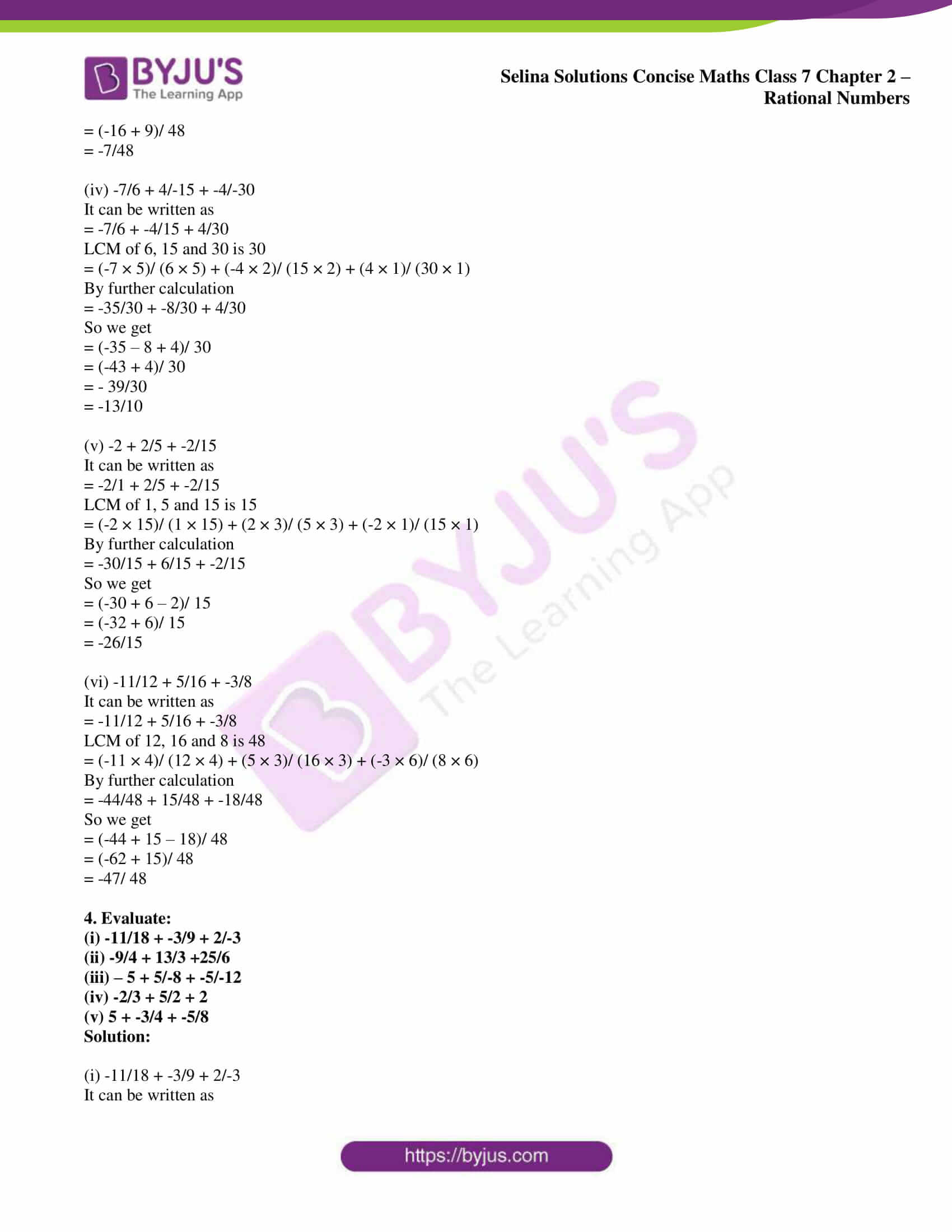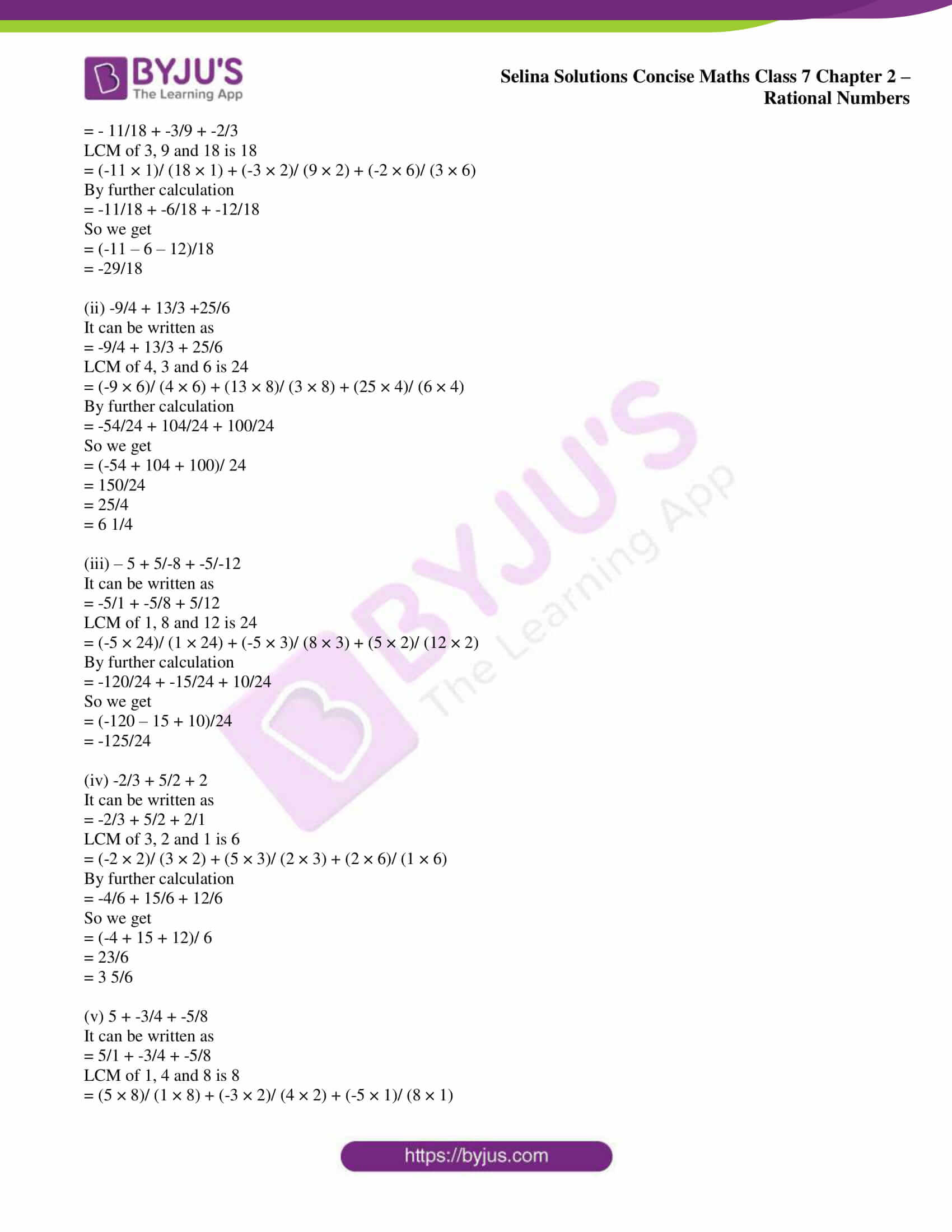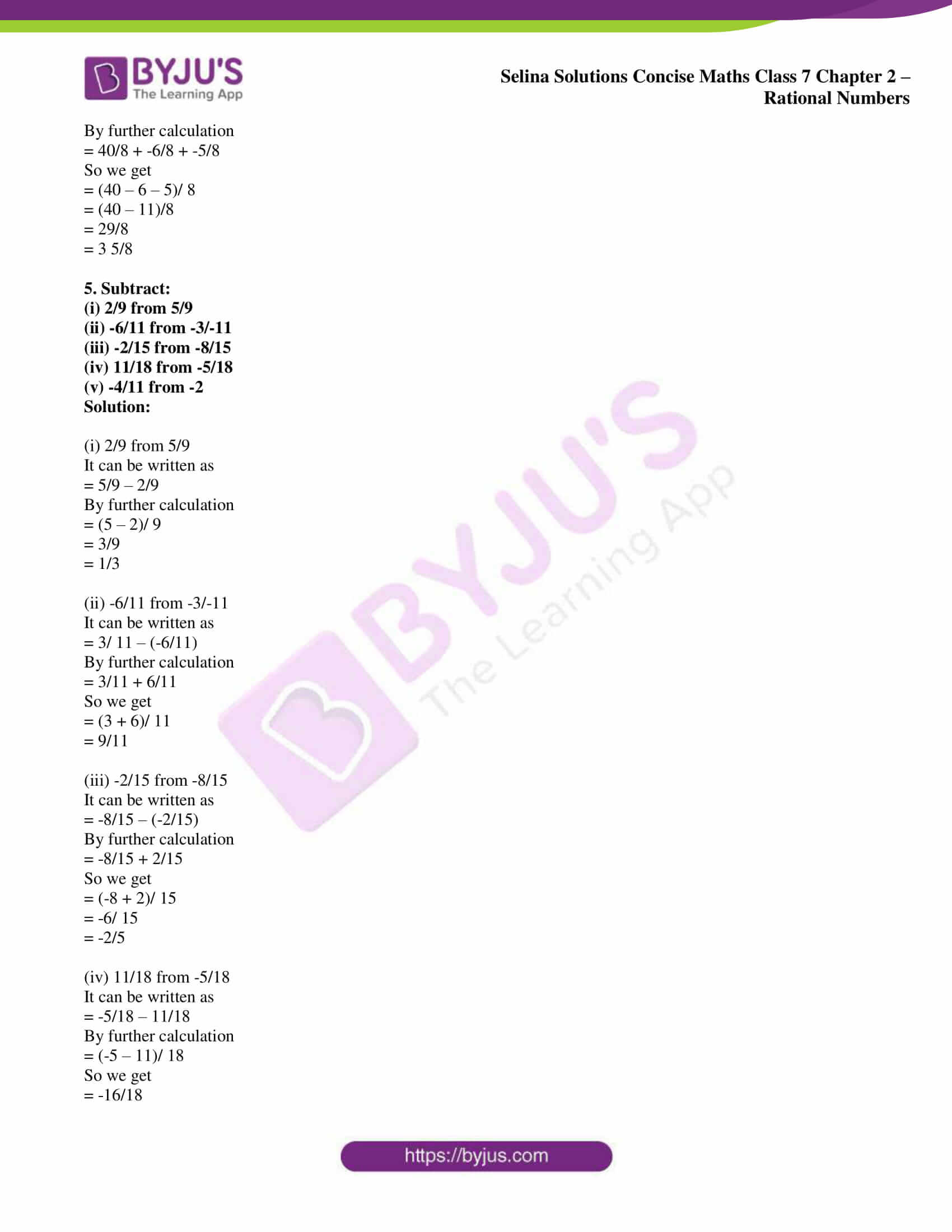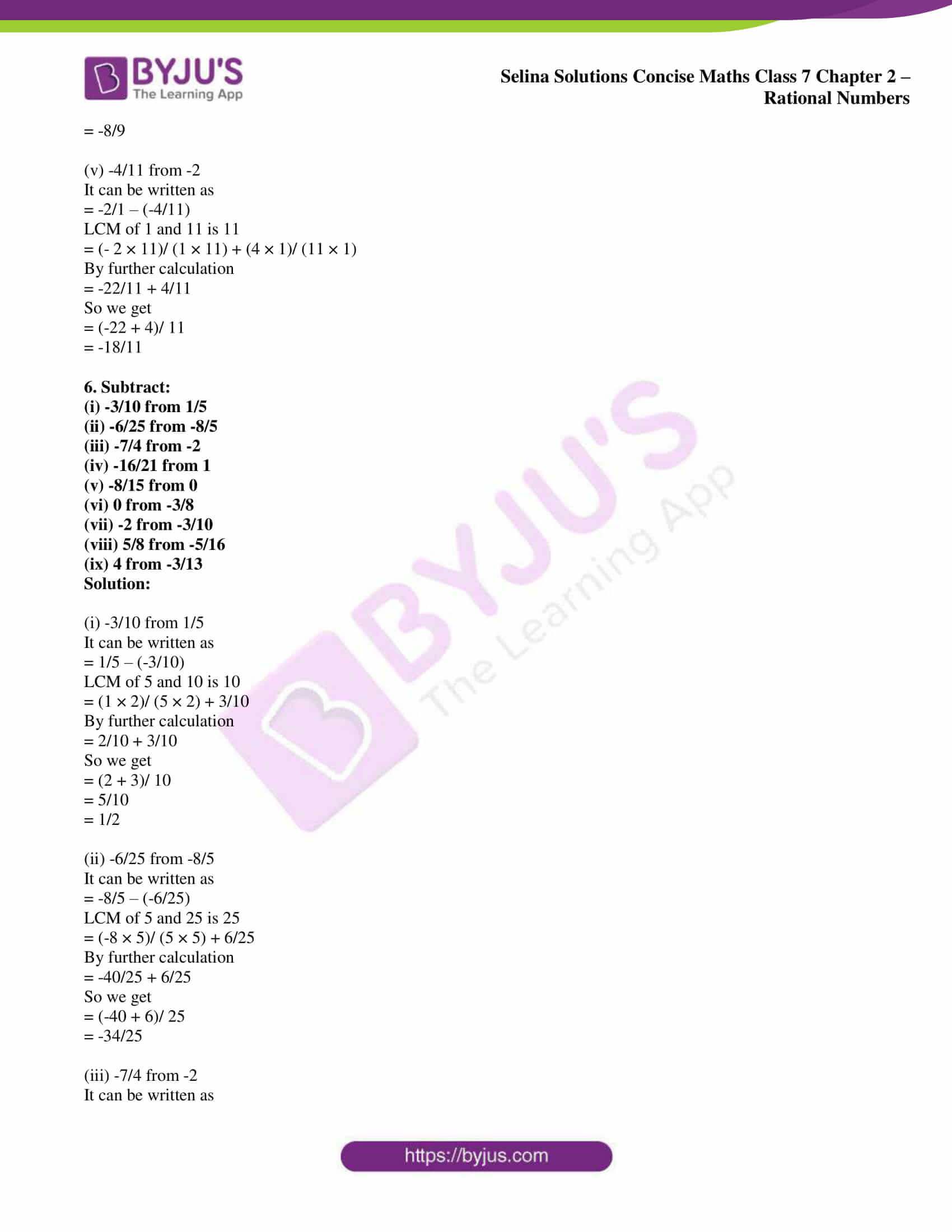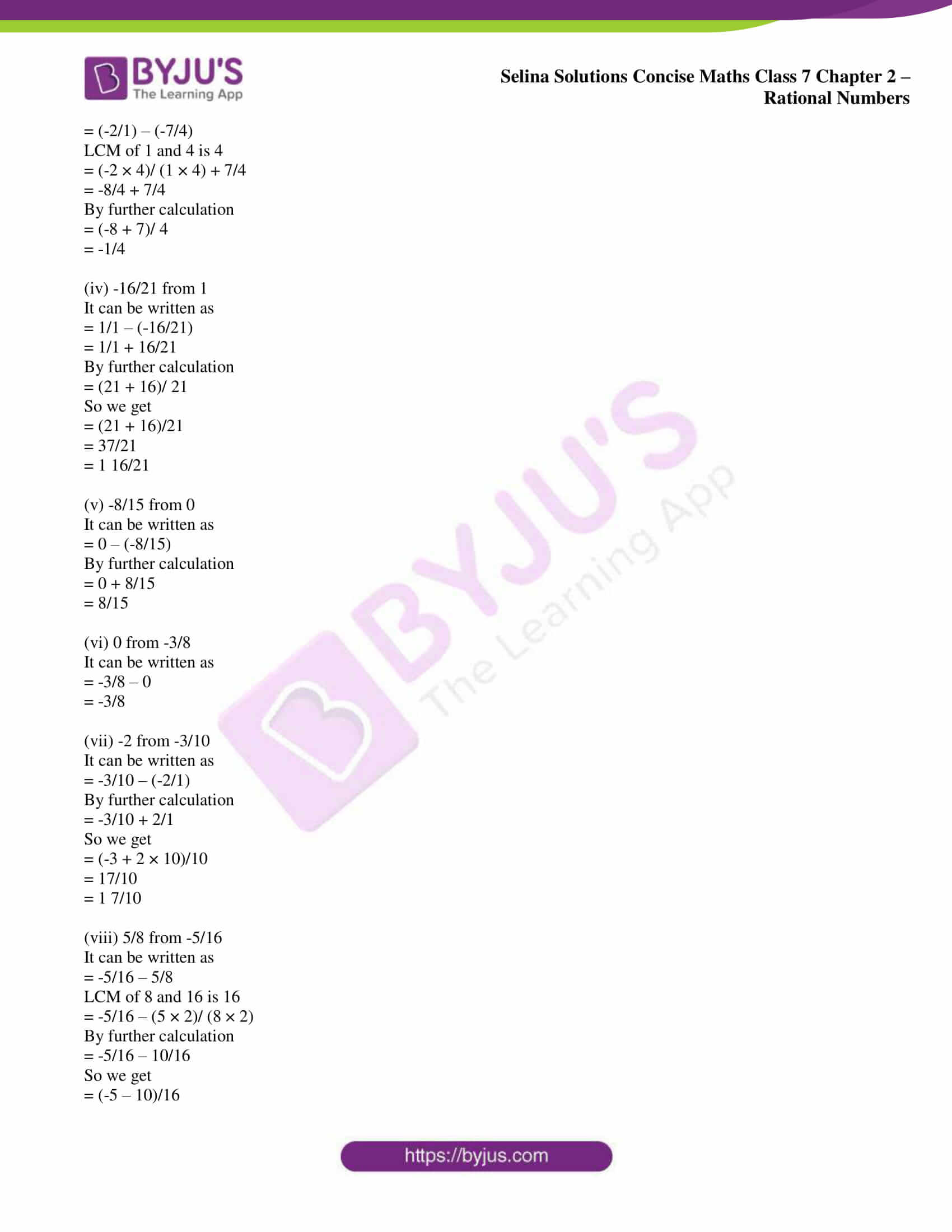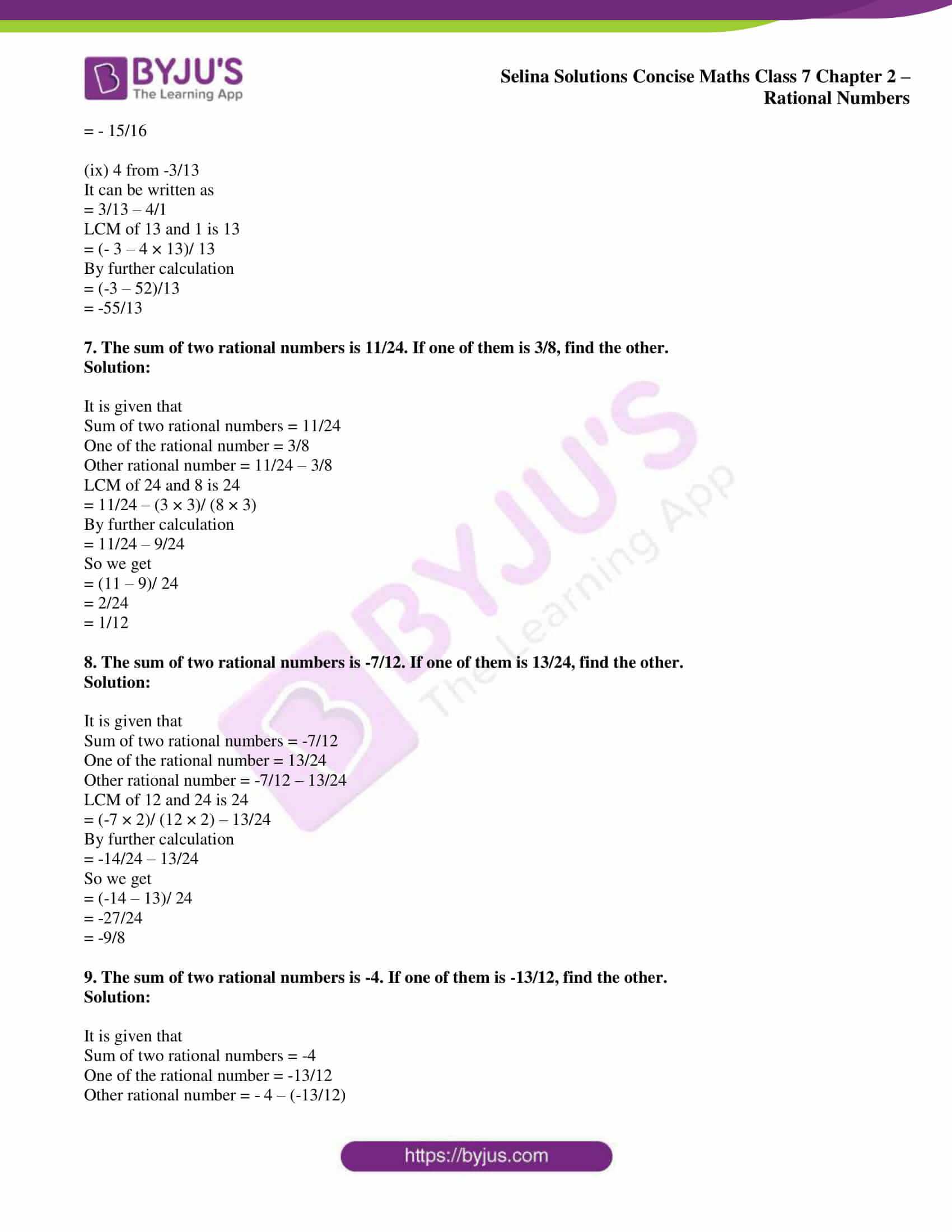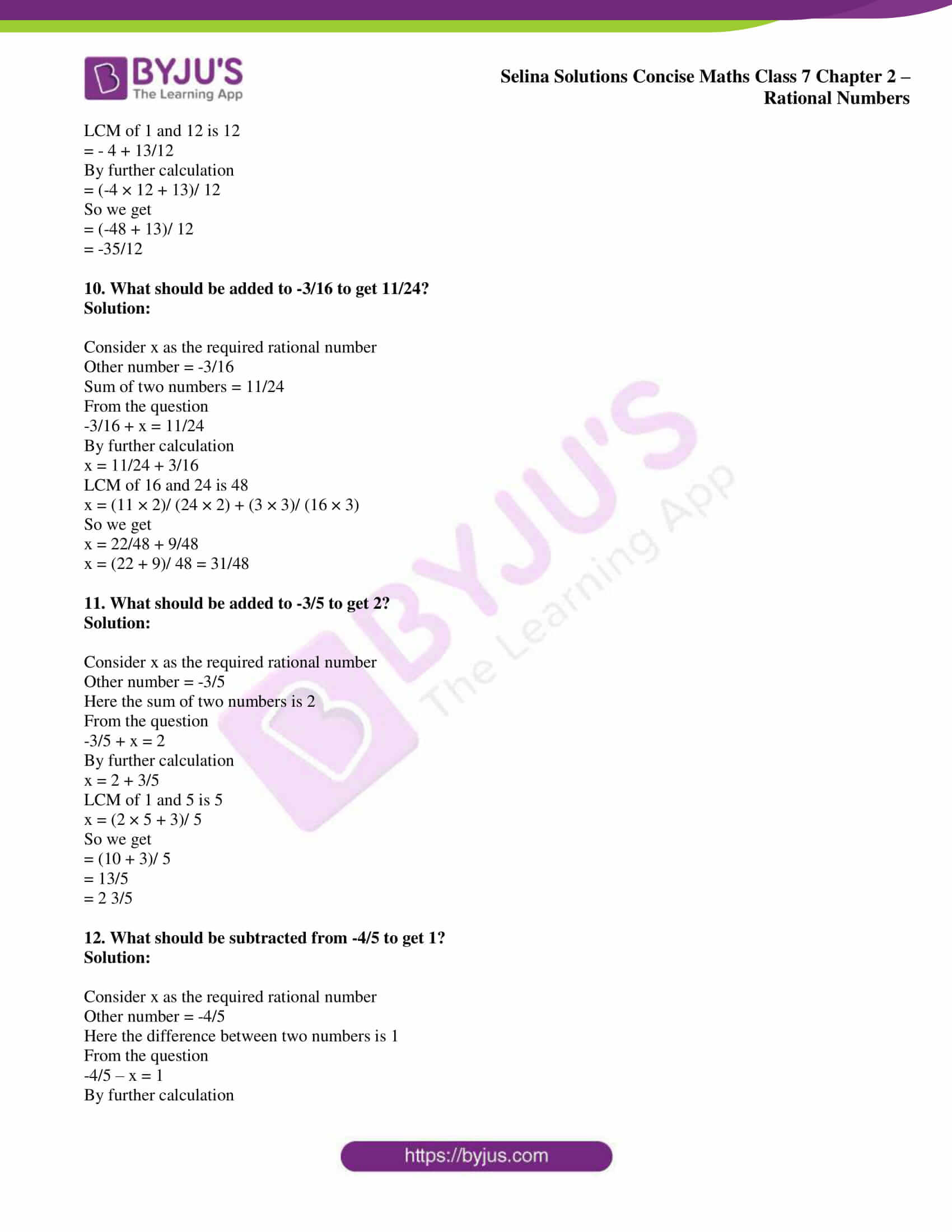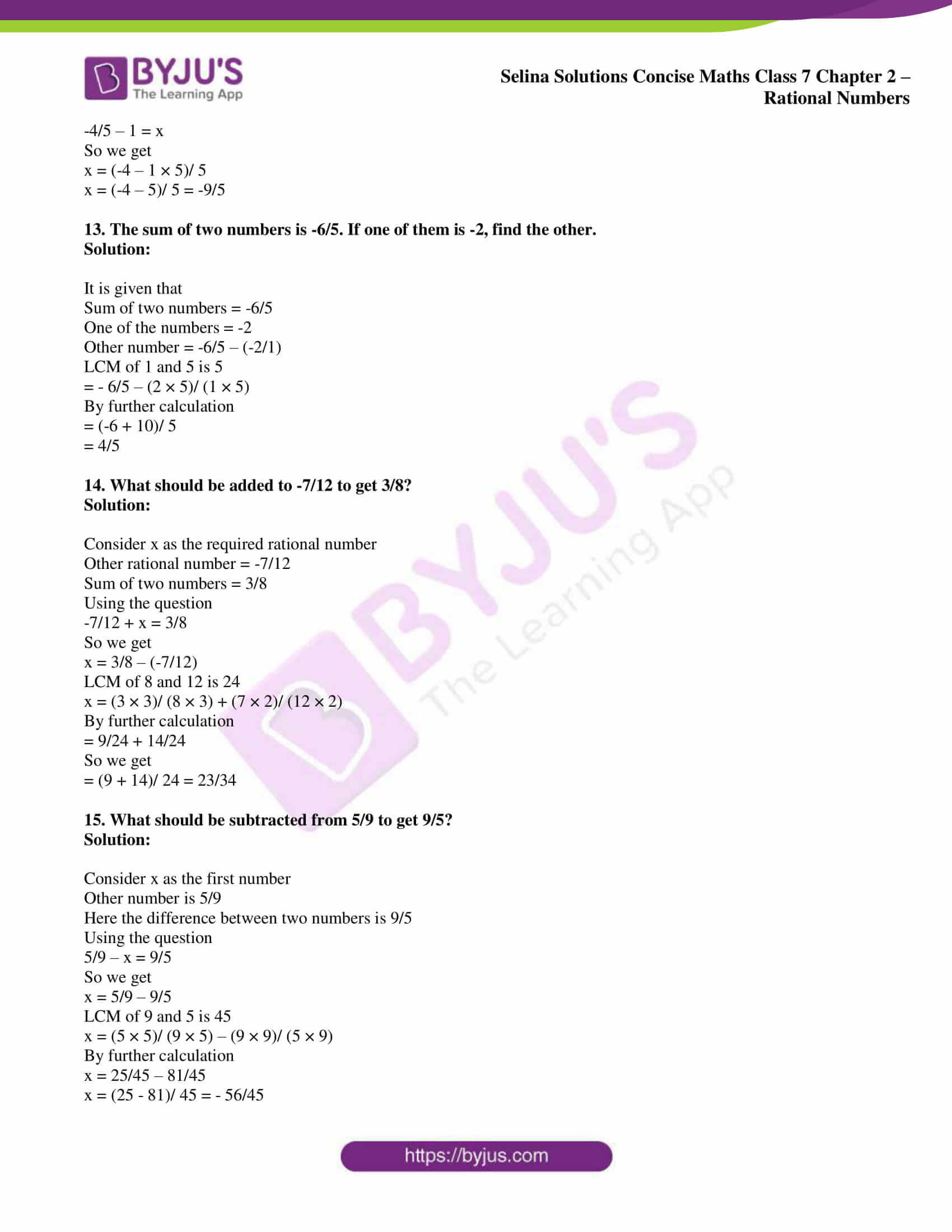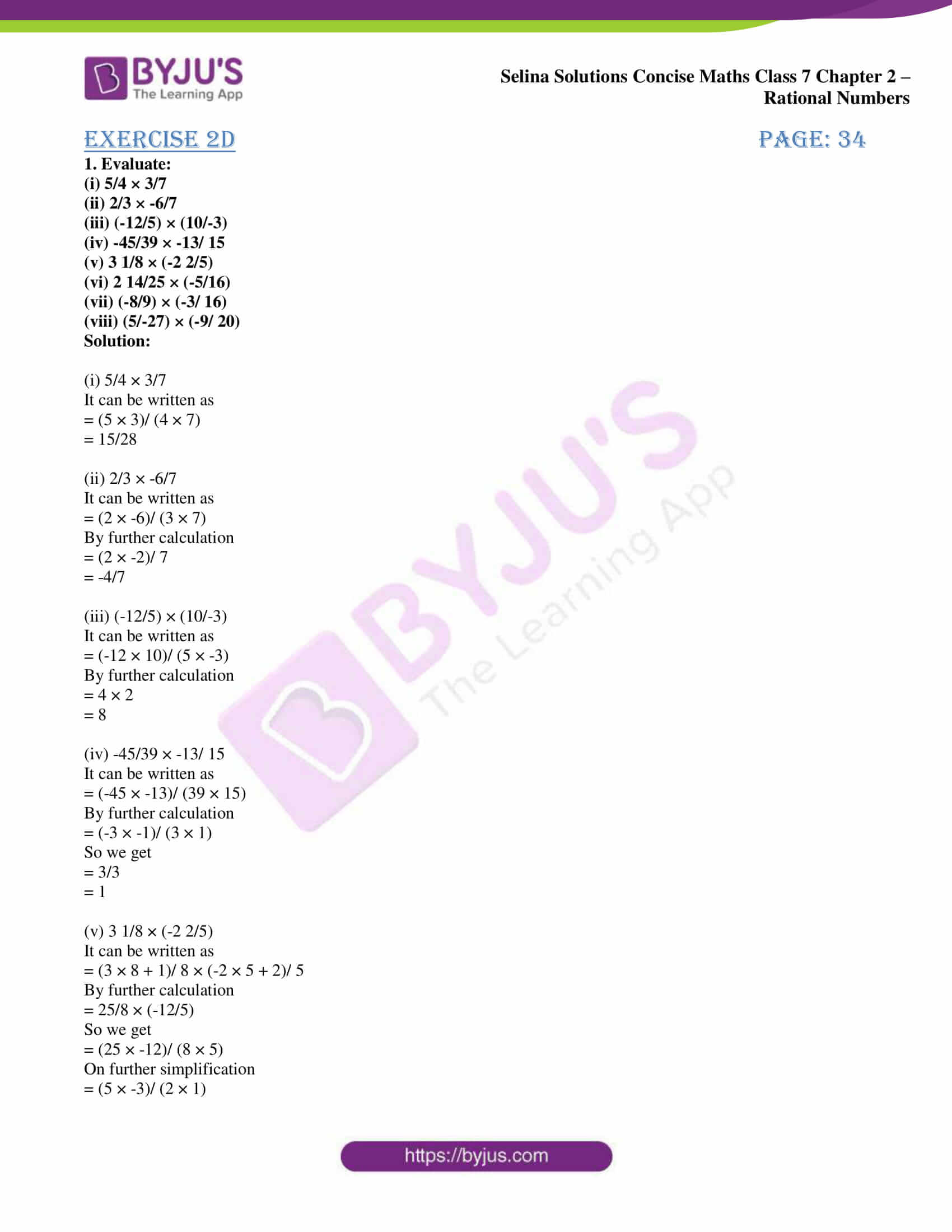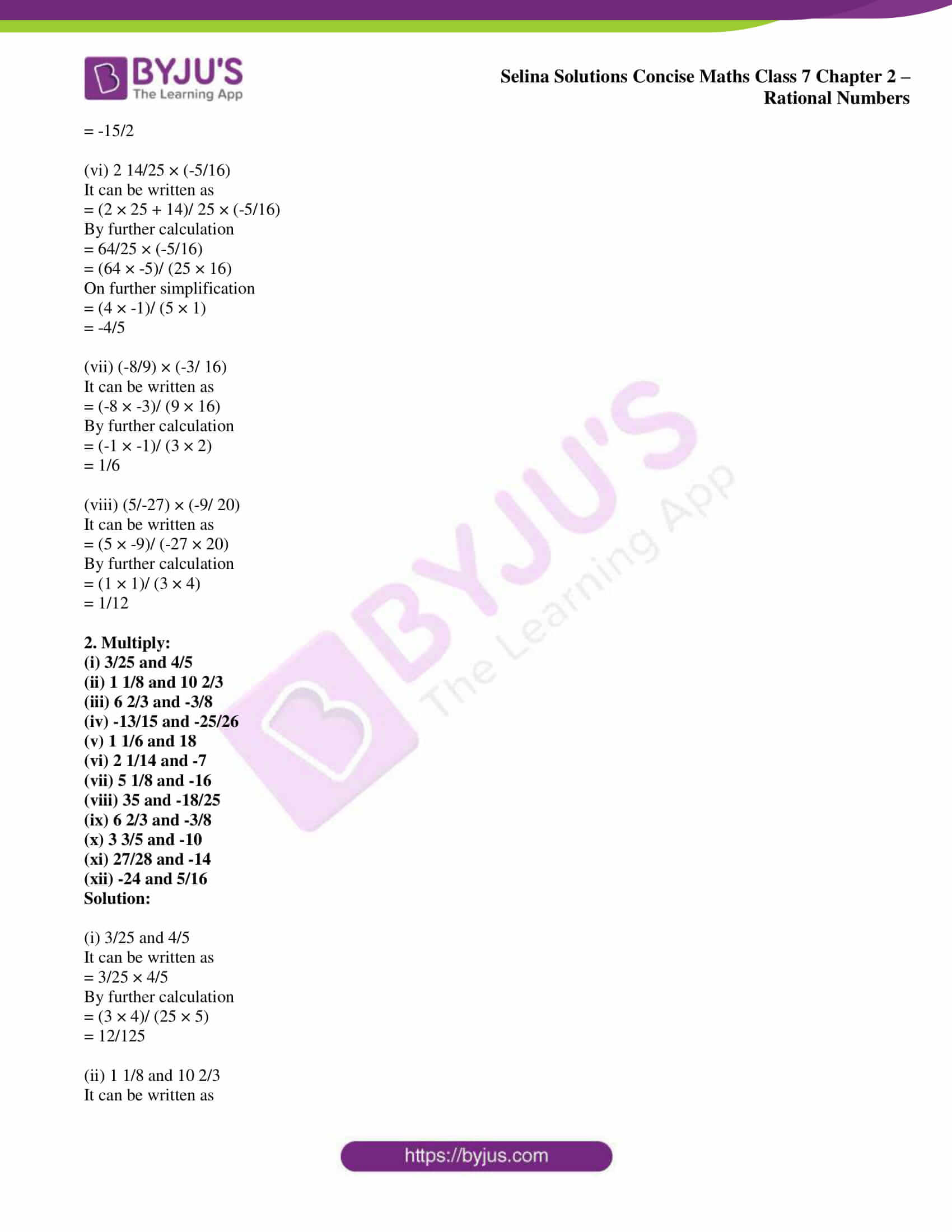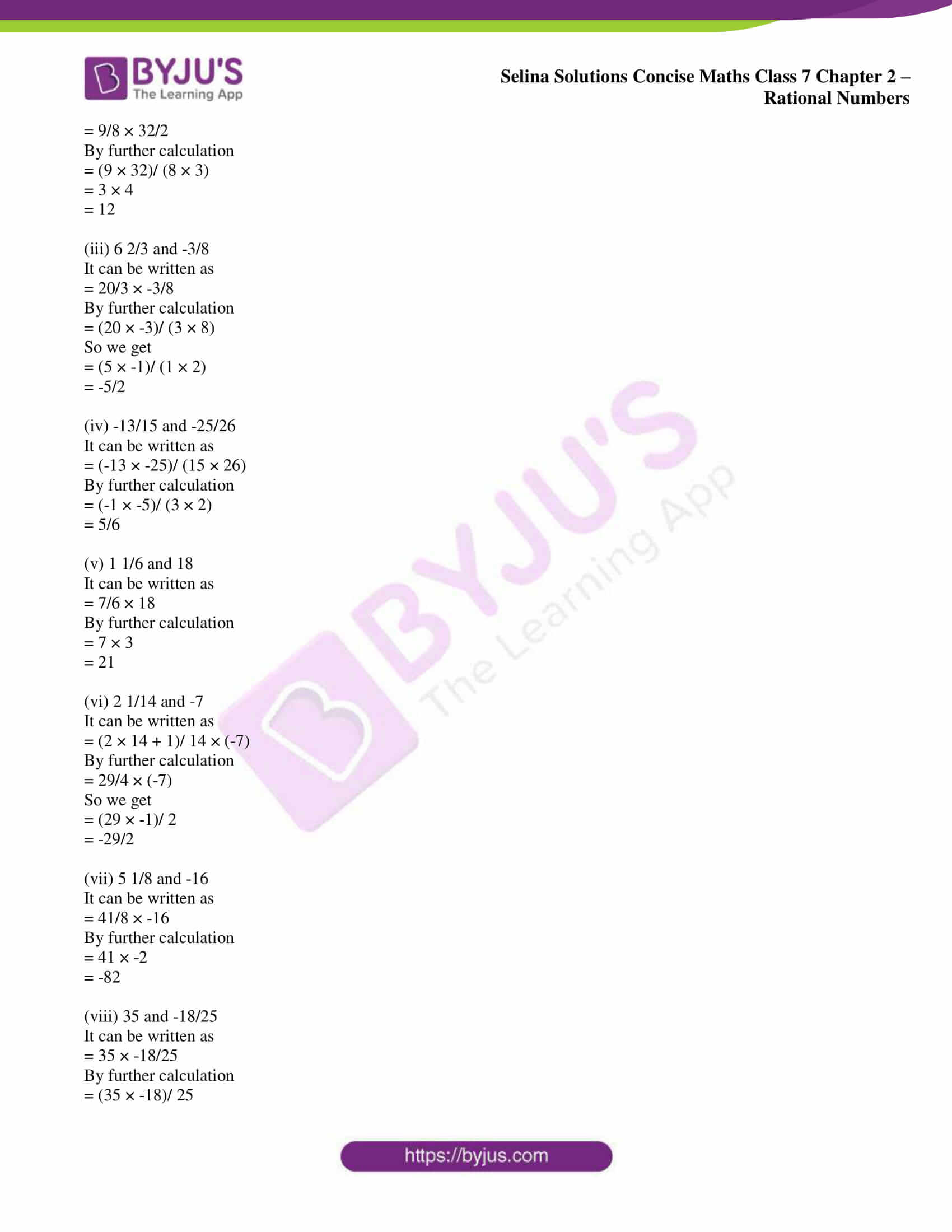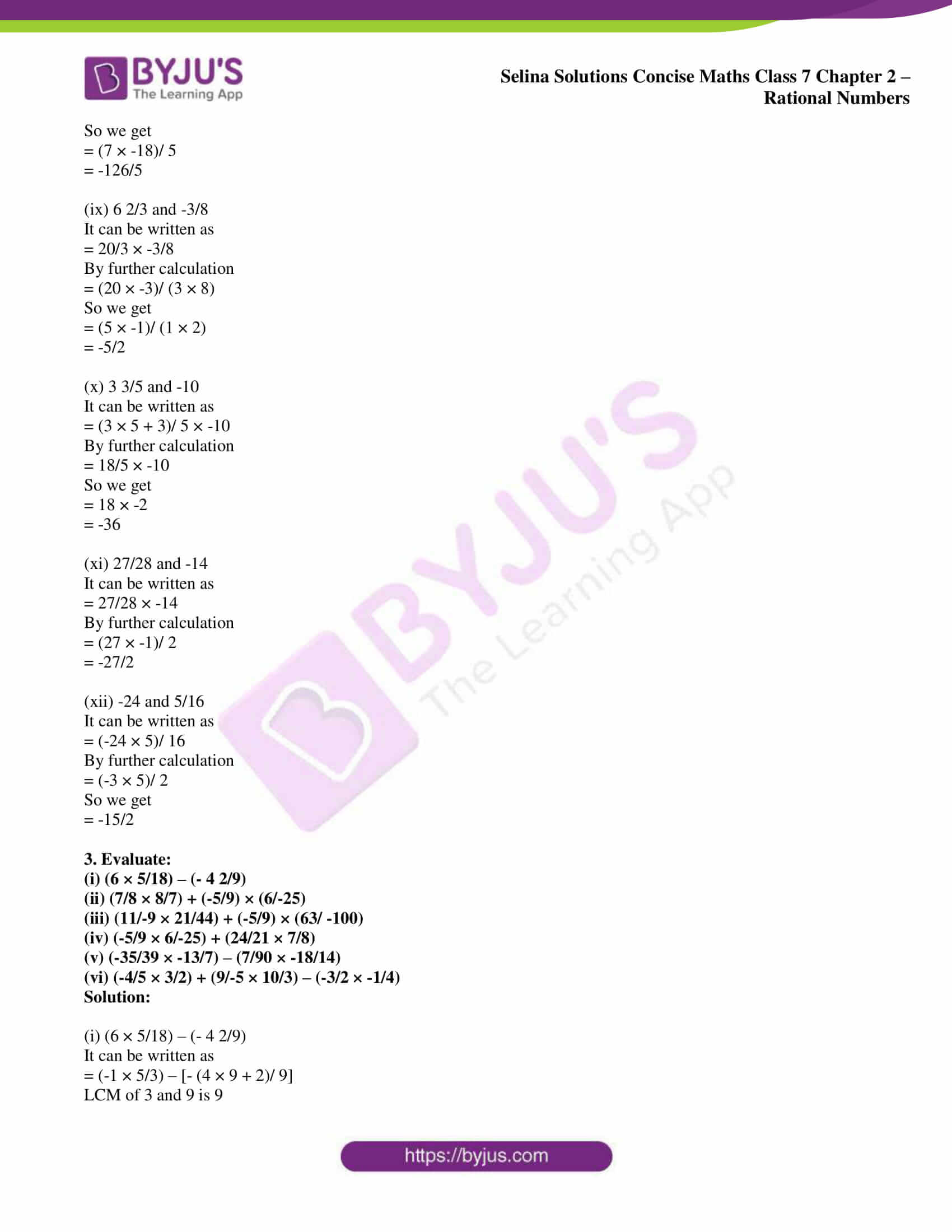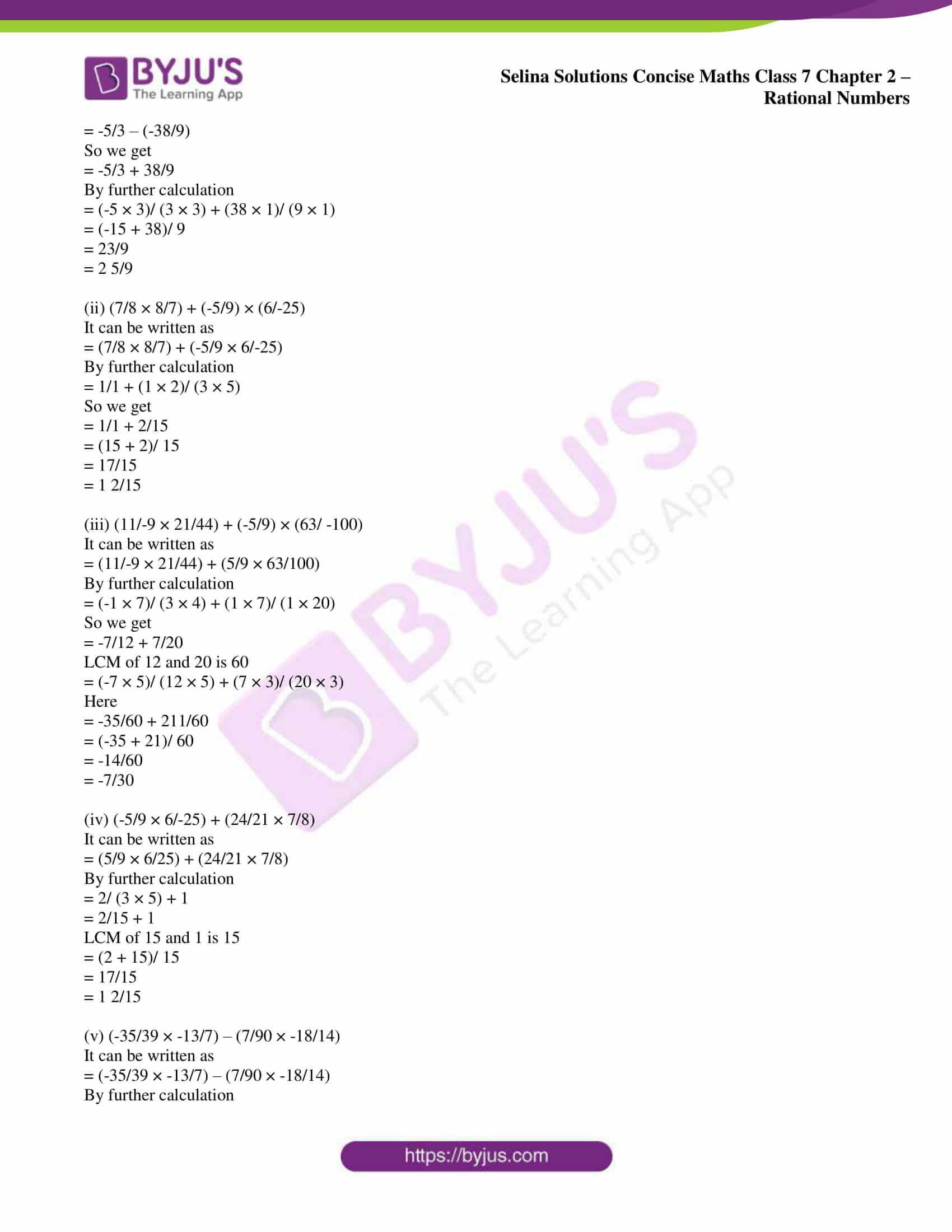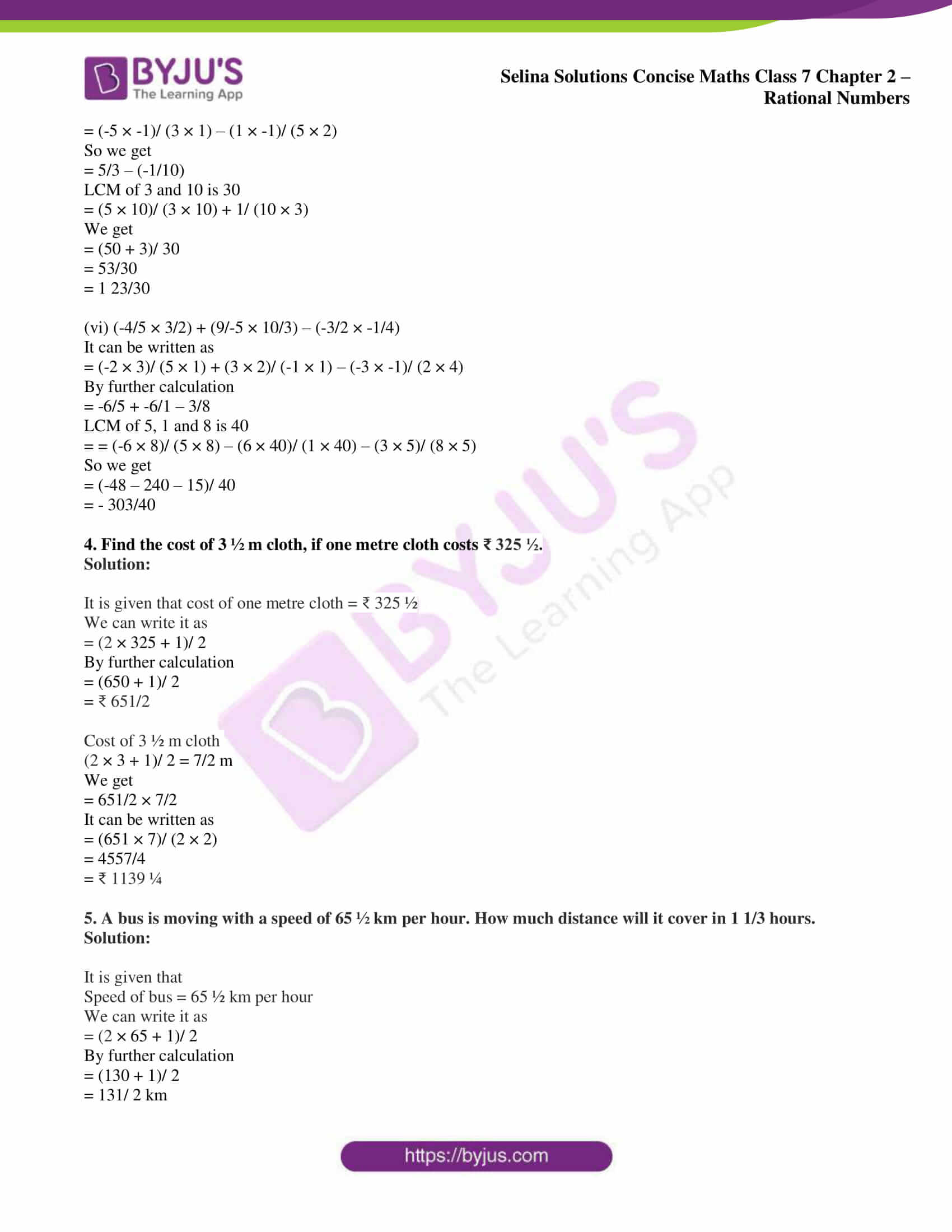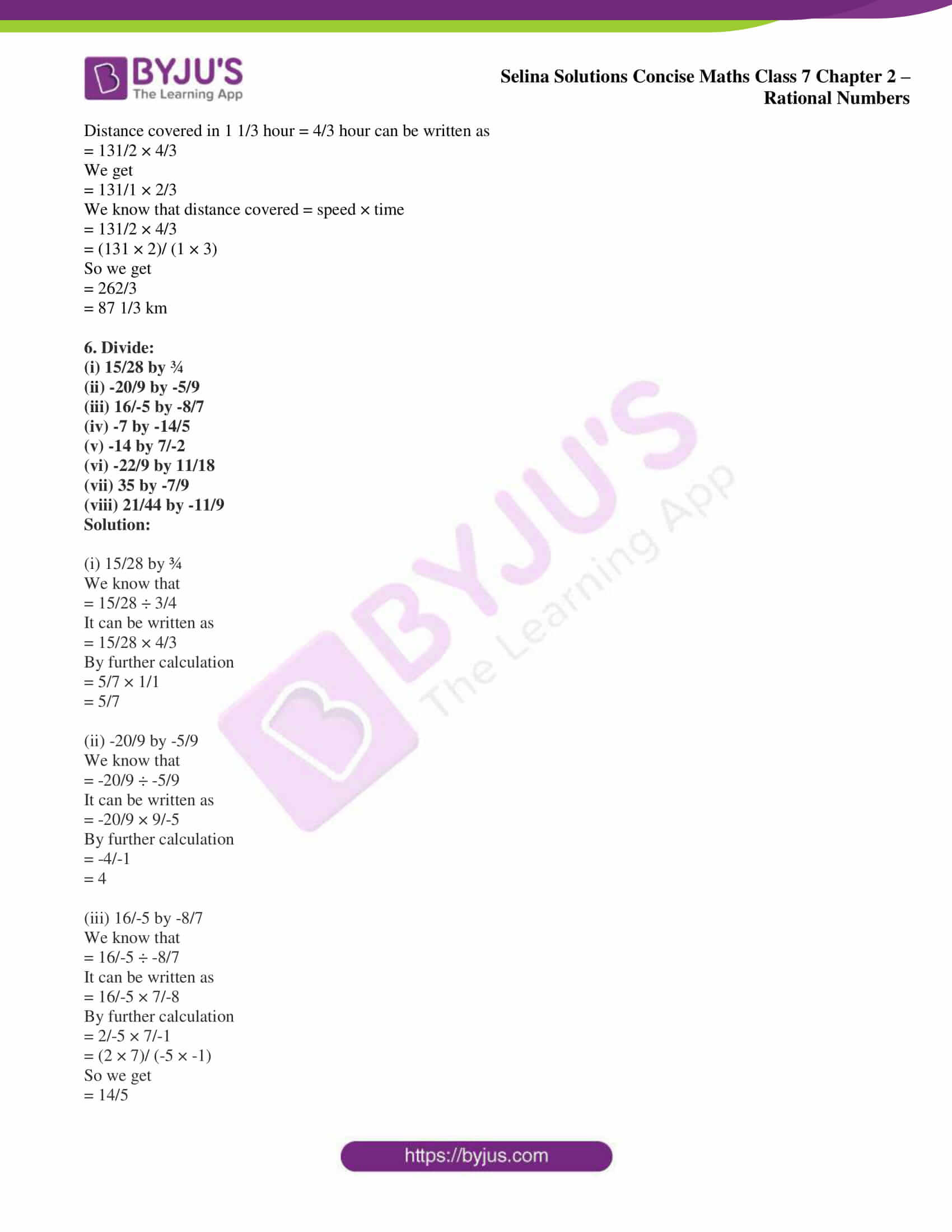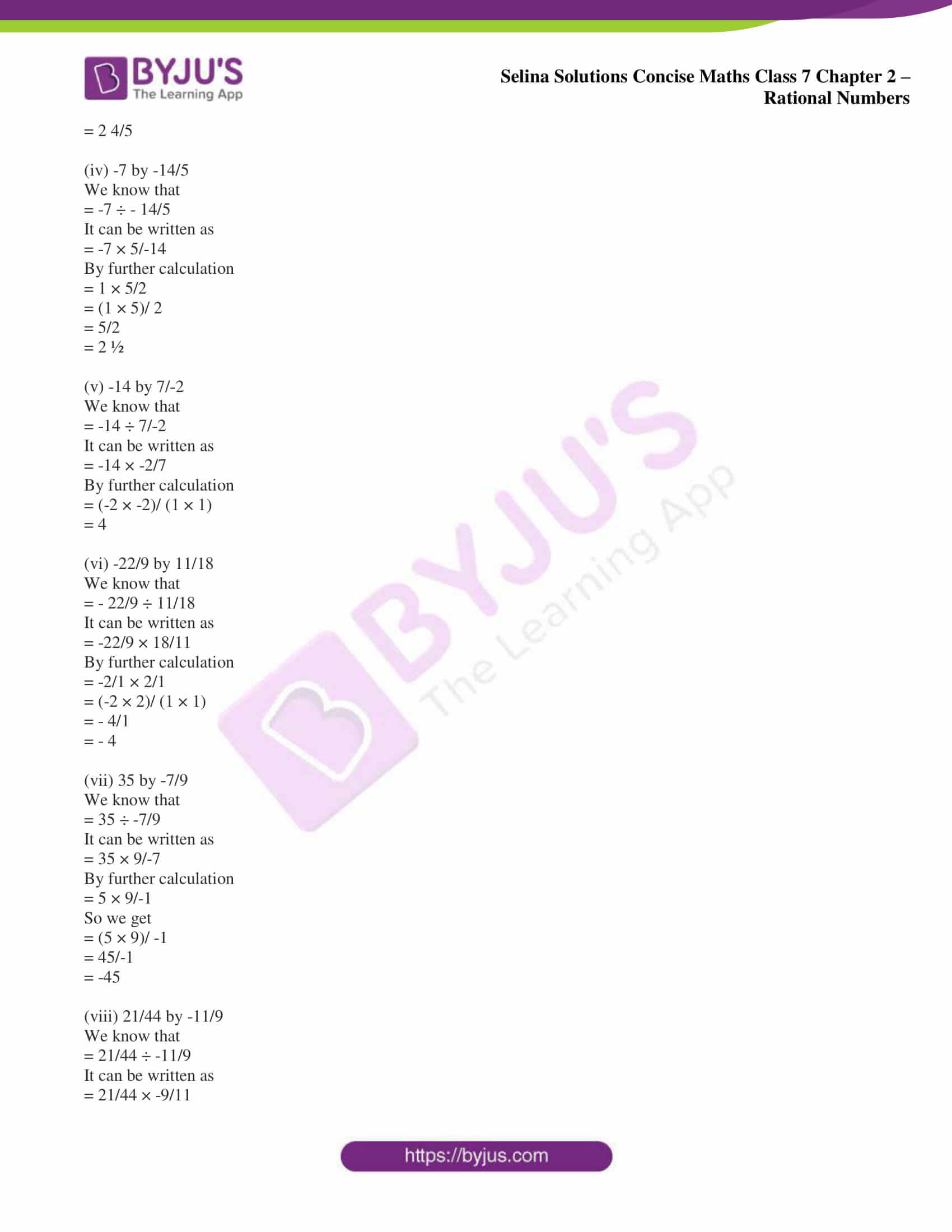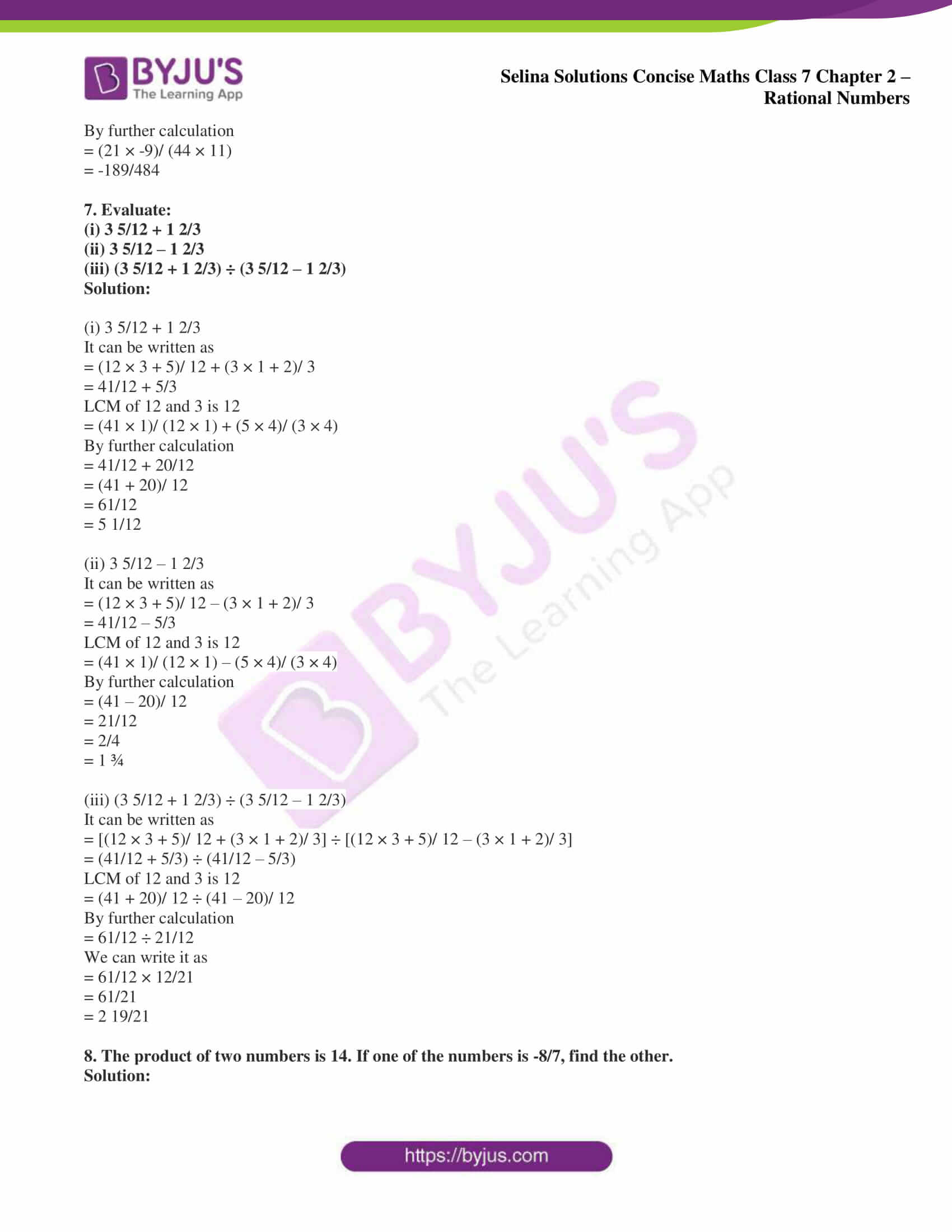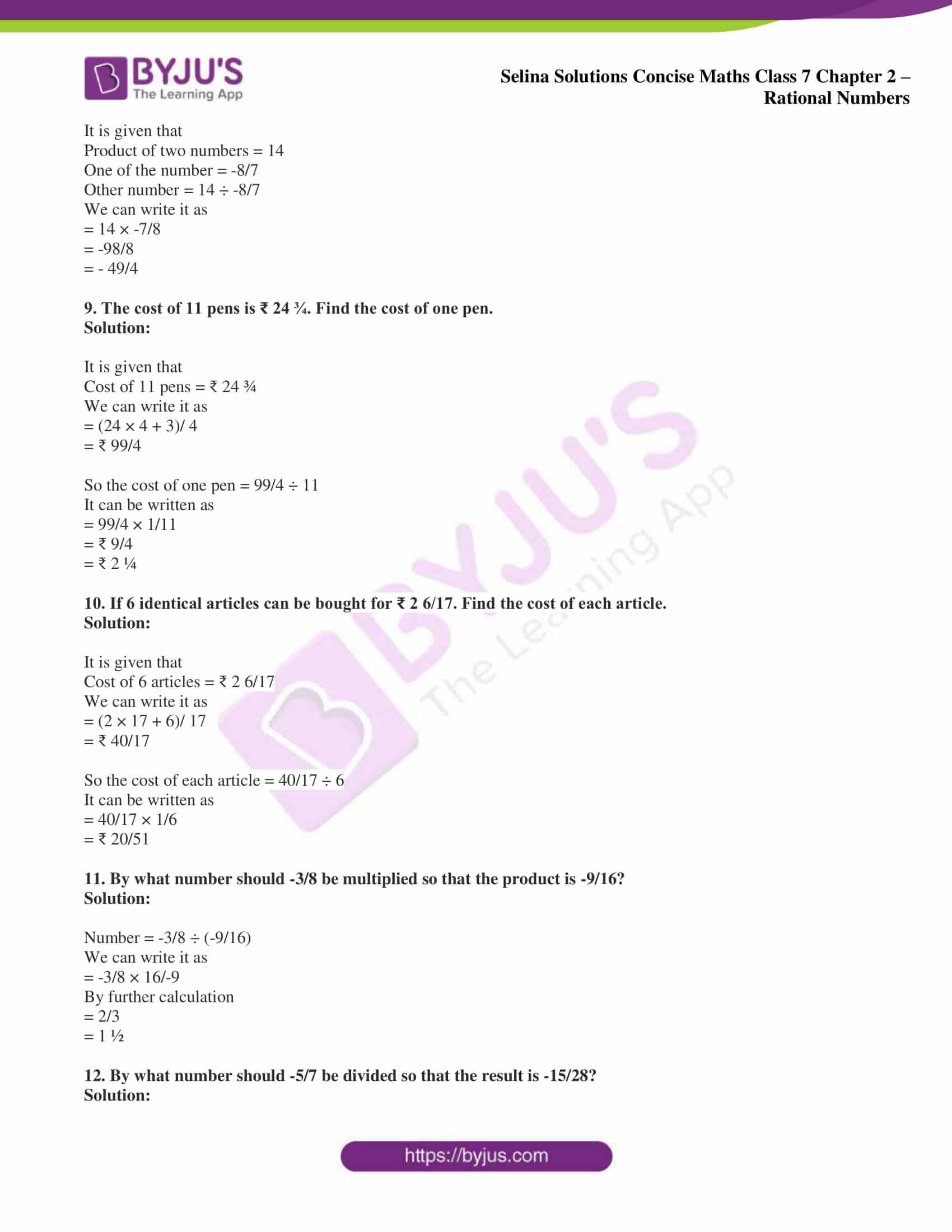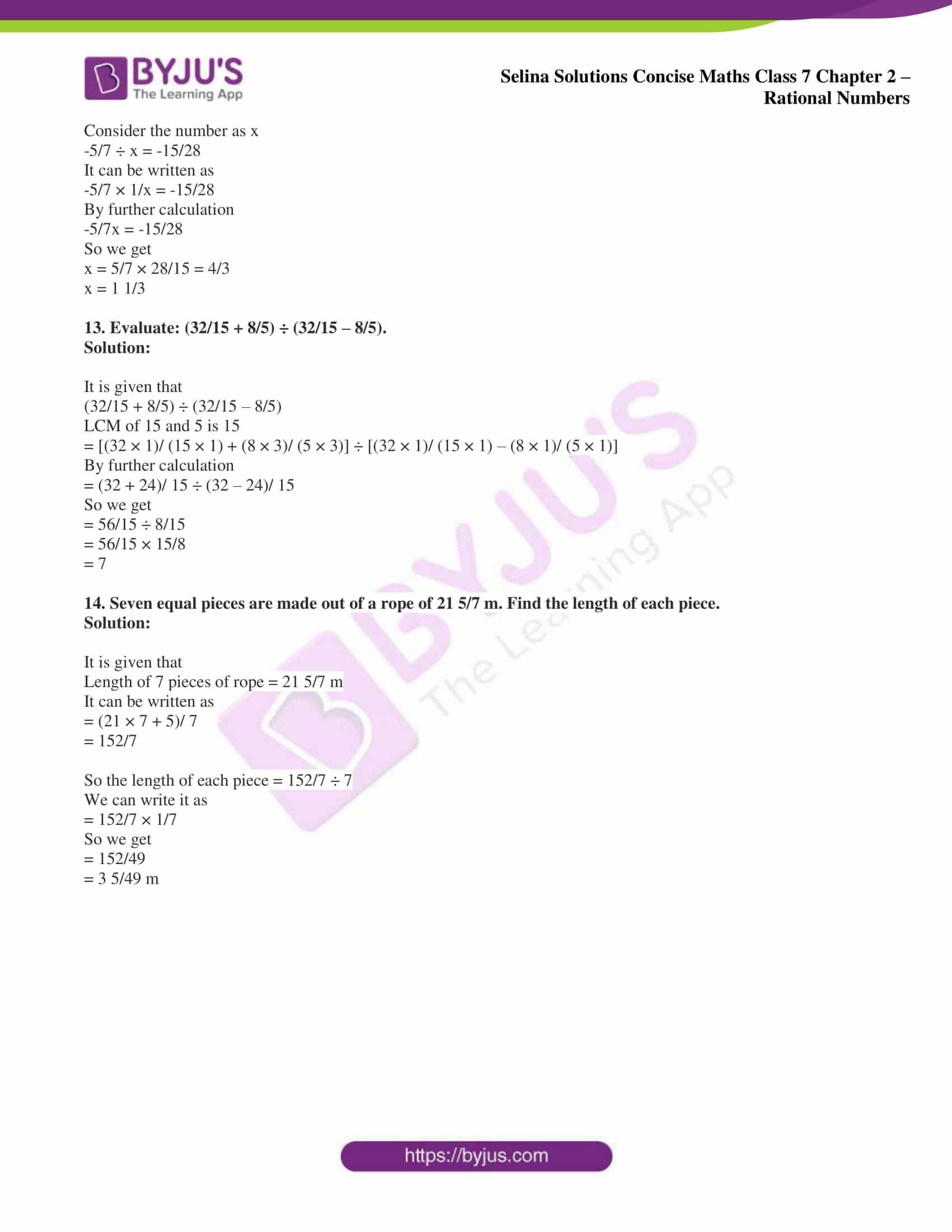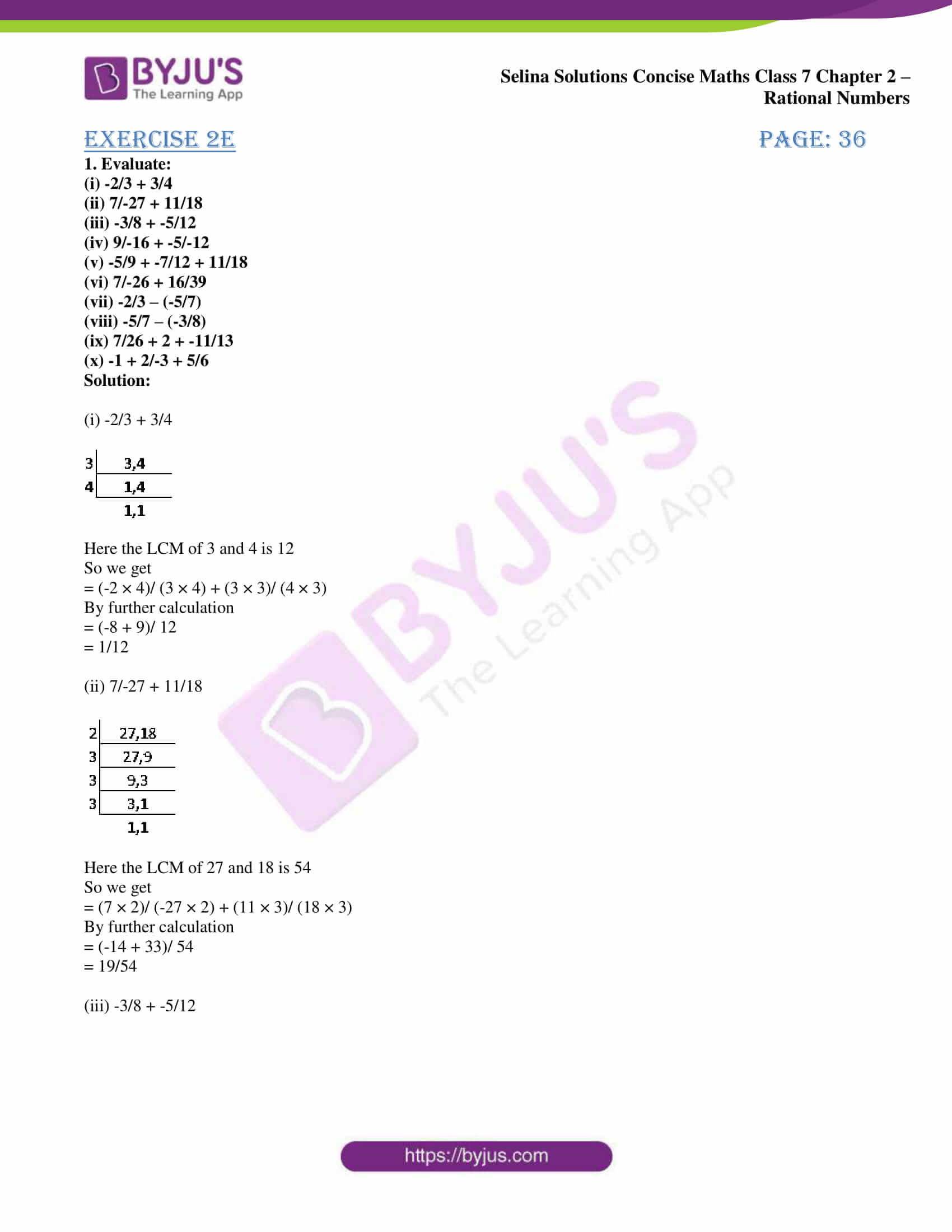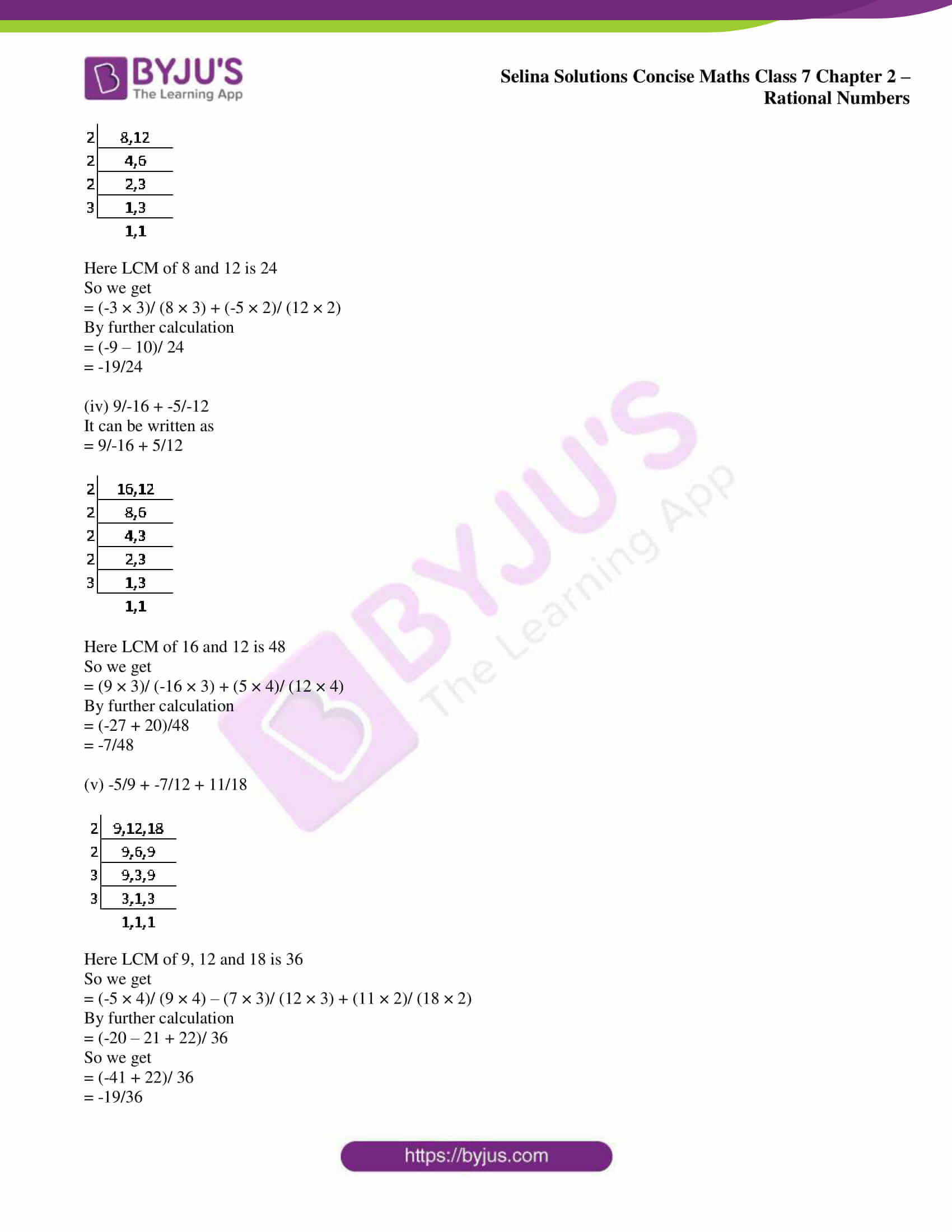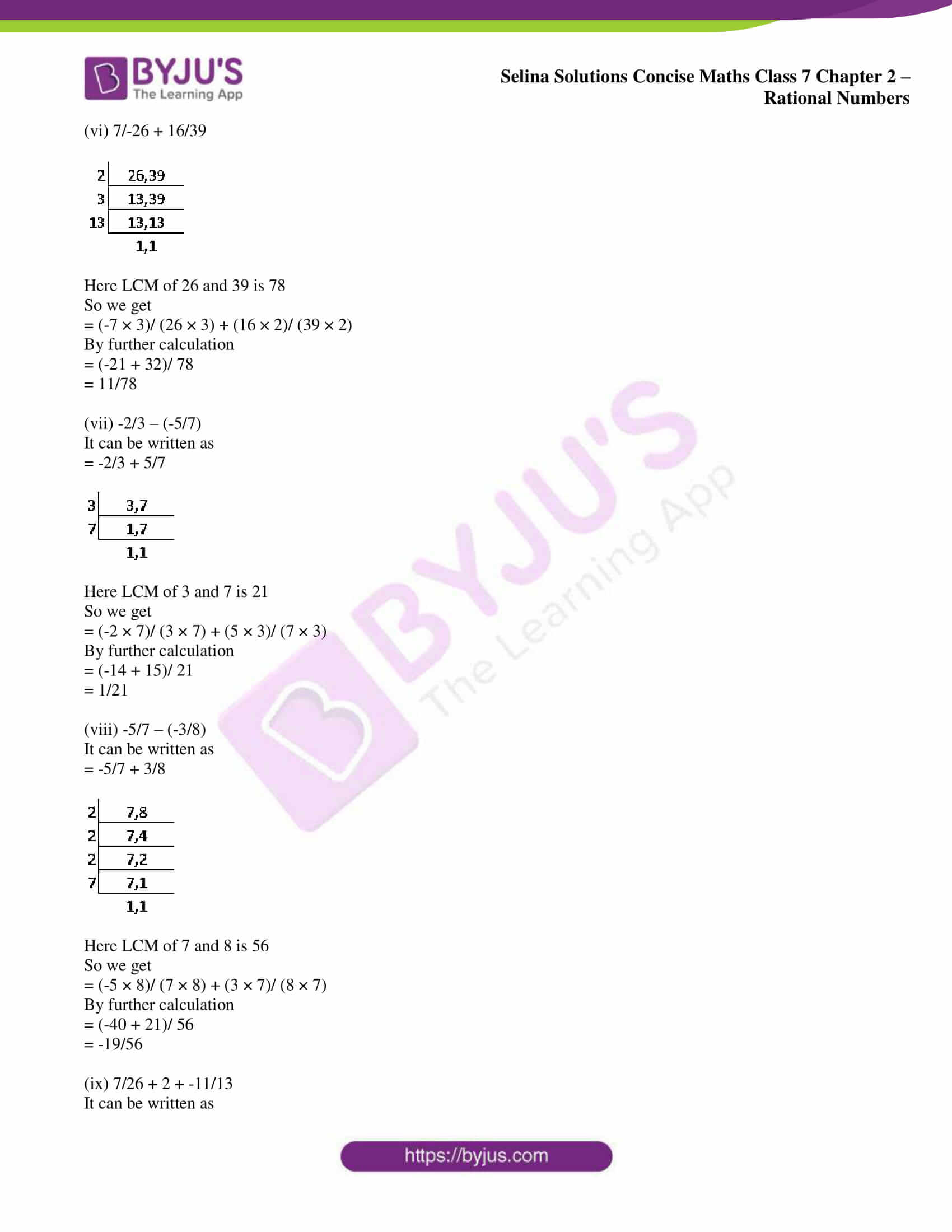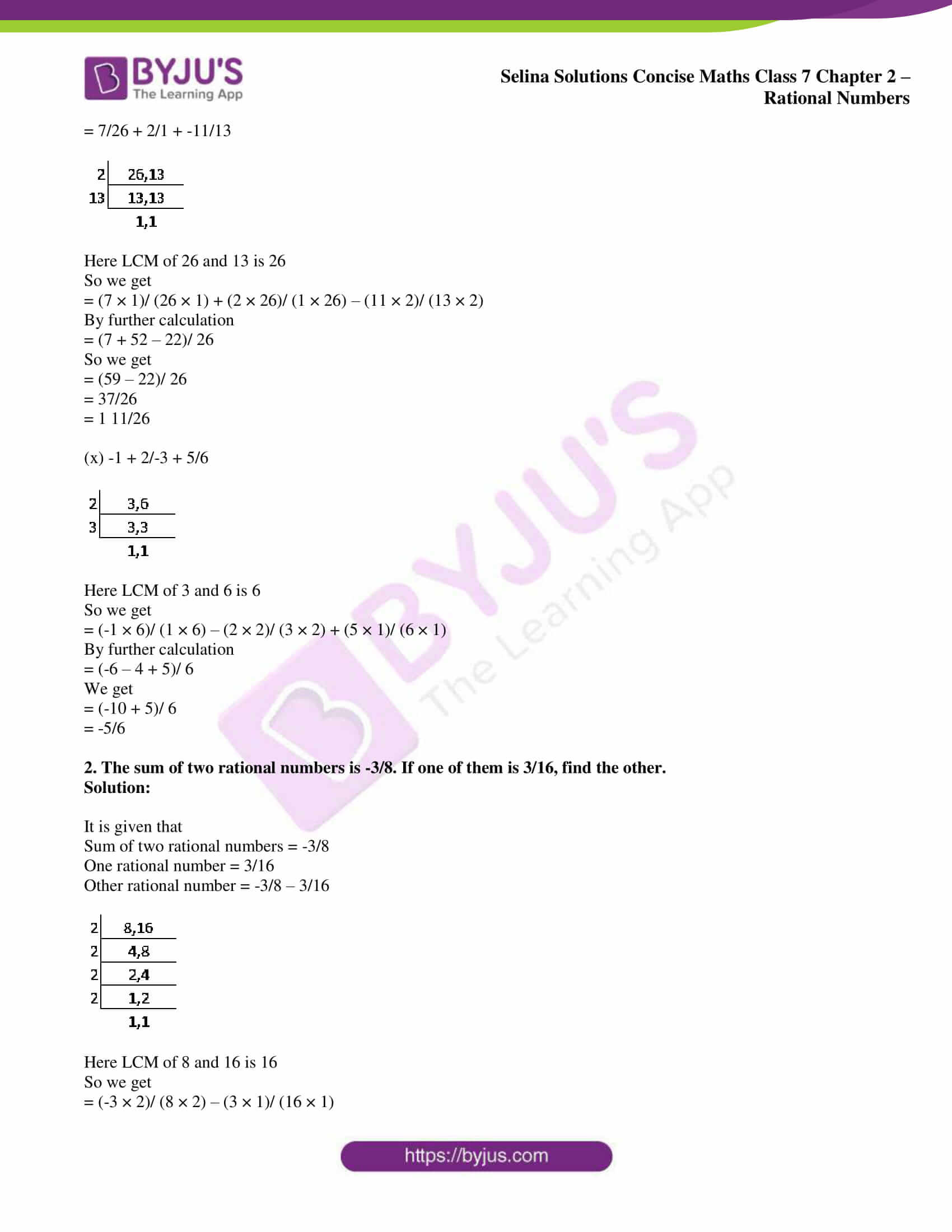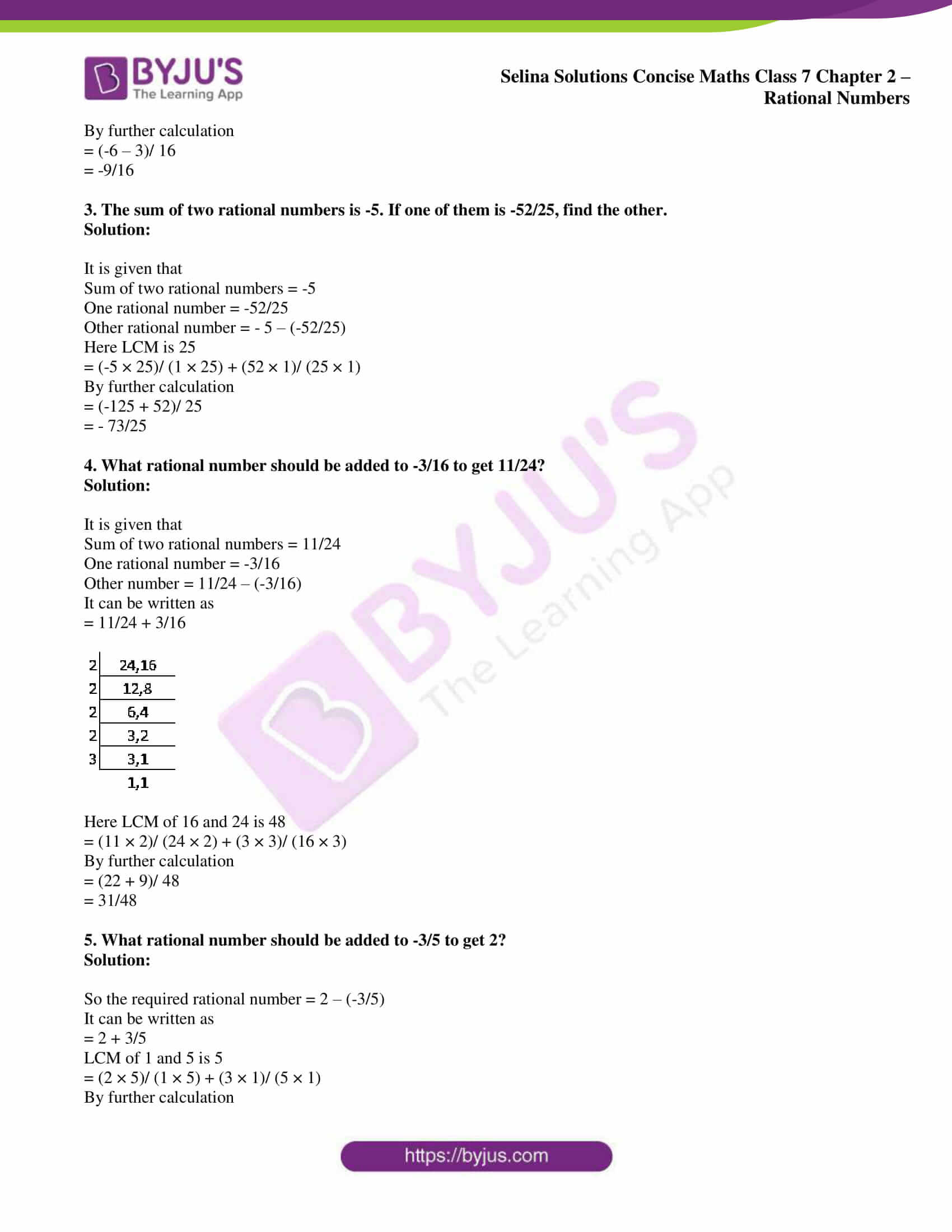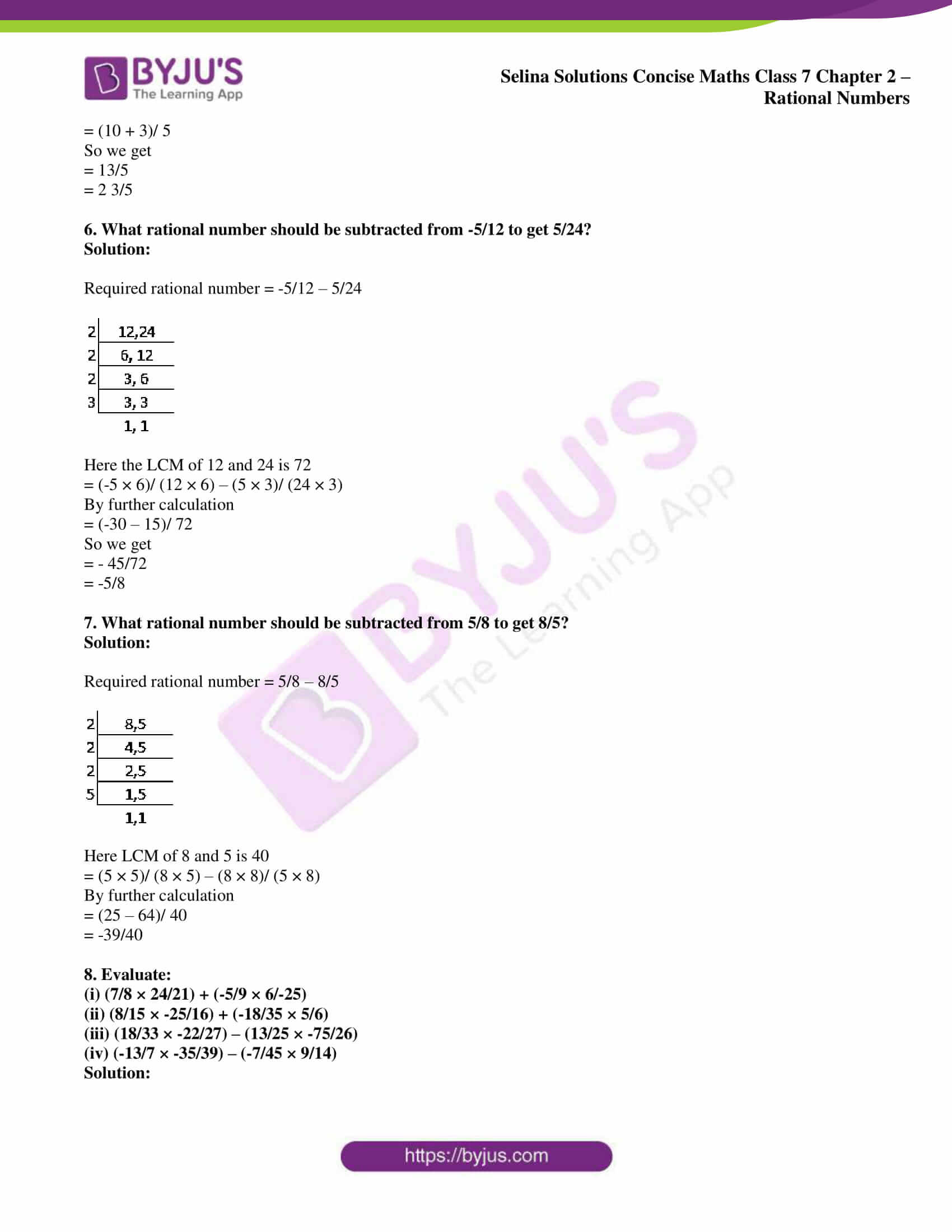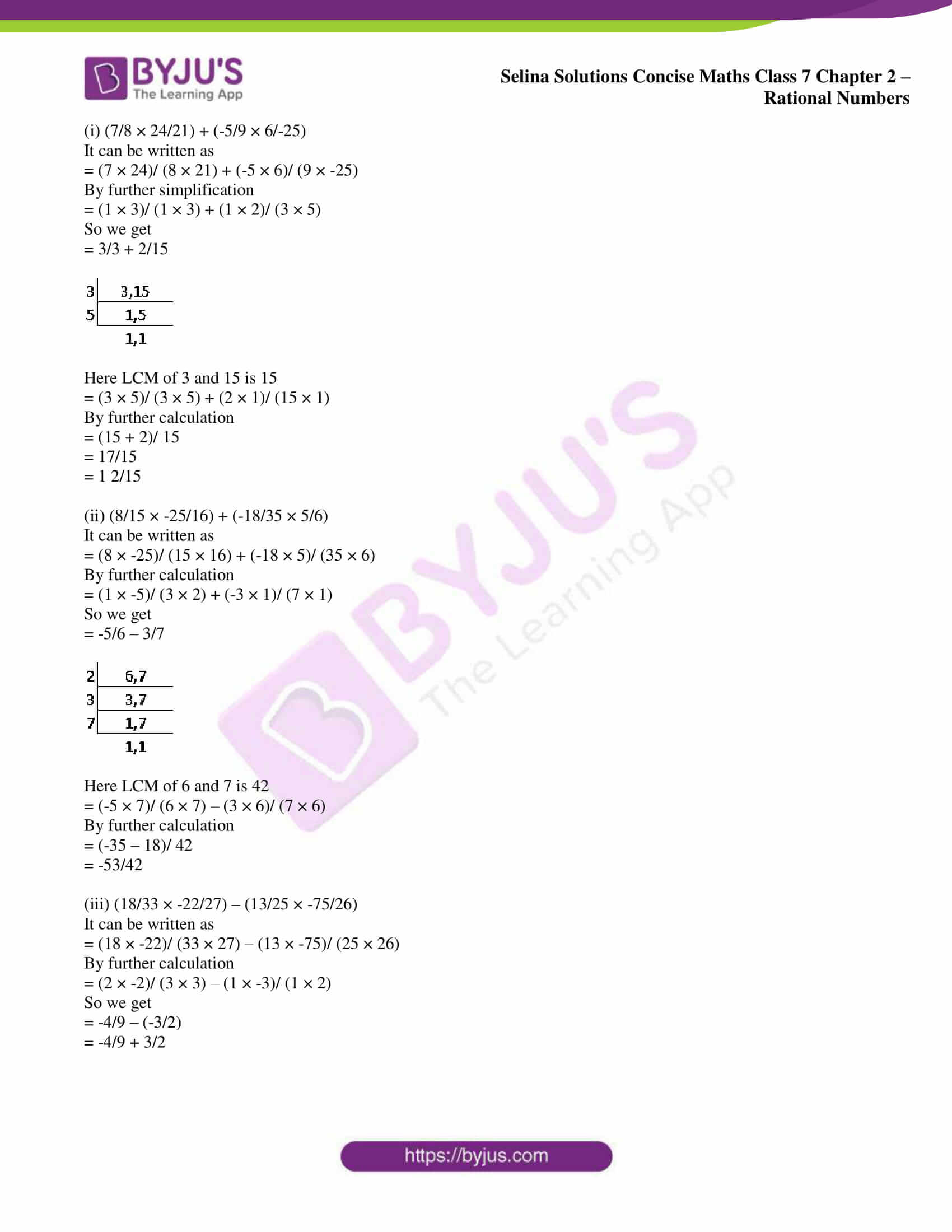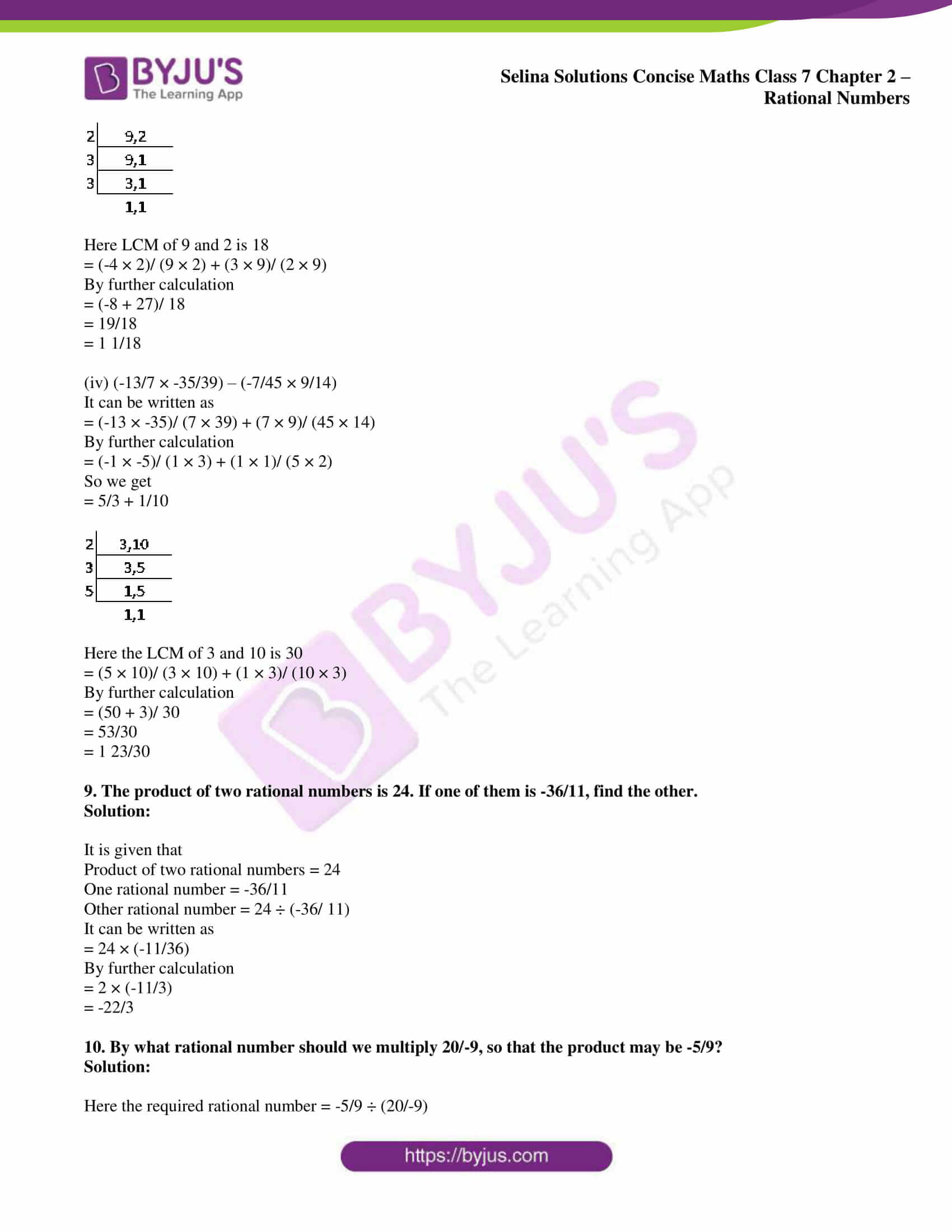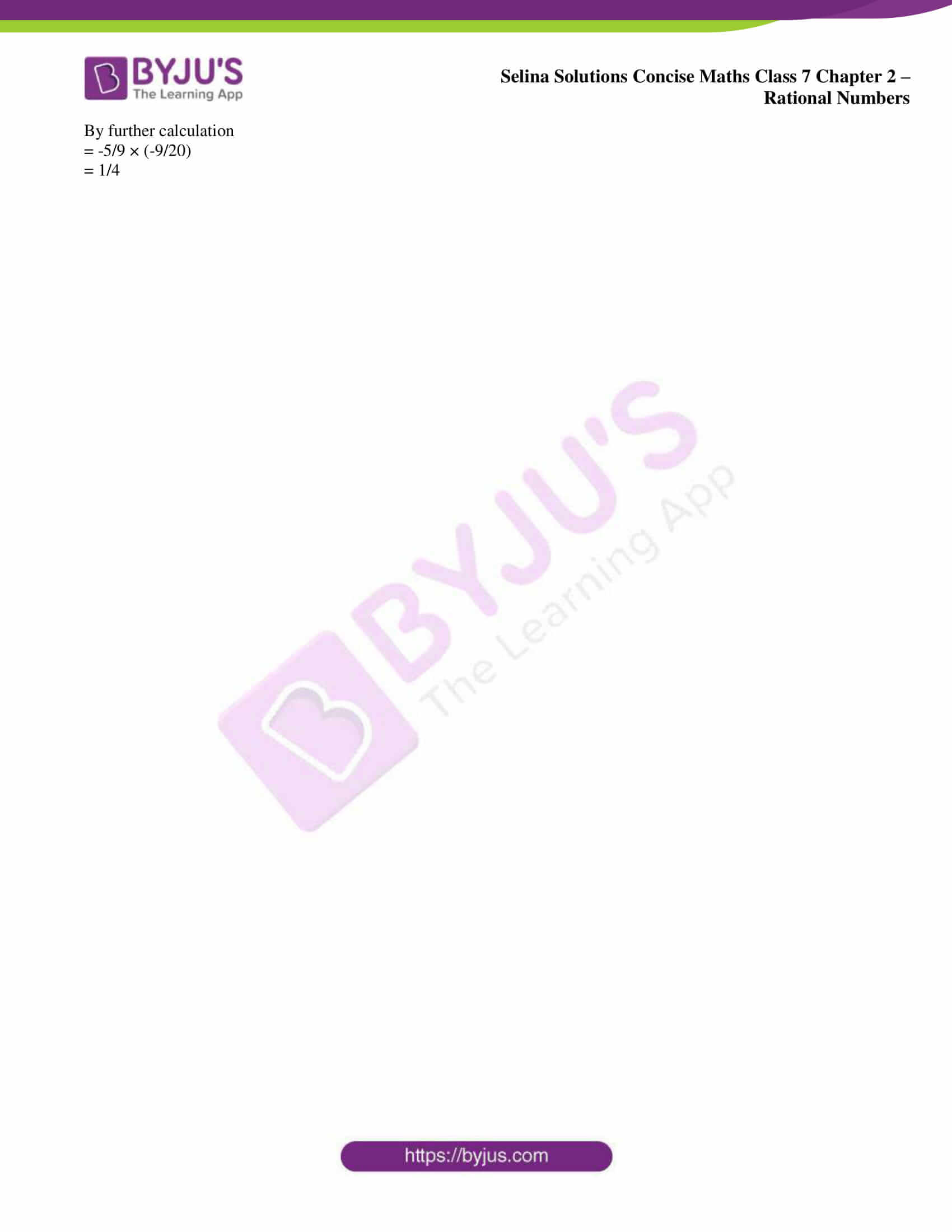## Access Selina Solutions Concise Maths Class 7 Chapter 2: Rational Numbers

#### Exercise 2A page: 19

1. Write down a rational number whose numerator is the largest number of two digits and denominator is the smallest number of four digits.

Solution:

We know that the largest two digit number is 99

So the smallest four digit number is 1000

Numerator = 99

Denominator = 1000

Rational number = 99/1000

2. Write the numerator of each of the following rational numbers:

(i) – 125/127

(ii) 37/ -137

(iii) – 85/ 93

(iv) 2

(v) 0

Solution:

(i) – 125/127

Here the numerator = – 125

(ii) 37/ -137

Here the numerator = 37

(iii) – 85/ 93

Here the numerator = – 85

(iv) 2 = 2/1

Here the numerator = 2

(v) 0 = 0/1

Here the numerator = 0

3. Write the denominator of each of the following rational numbers:

(i) 7/ -15

(ii) – 18/29

(iii) – 3/4

(iv) – 7

(v) 0

Solution:

(i) 7/ -15

Here the denominator = – 15

(ii) – 18/29

Here the denominator = 29

(iii) – 3/4

Here the denominator = 4

(iv) – 7 = -7/1

Here the denominator = 1

(v) 0 = 0/1

Here the denominator = 1

4. Write down a rational number with numerator (-5) × (-4) and with denominator (28 – 27) × (8 – 5).

Solution:

It is given that

Numerator = (-5) × (-4) = 20

Denominator = (28 – 27) × (8 – 5) = 1 × 3 = 3

So the rational number = 20/3

5. (i) – 15/1 in integer form is …….

(ii) 23/-1 in integer form is ……..

(iii) If 18 = 18/a then a = …….

(iv) If – 57 = 57/a then a = ………

Solution:

(i) – 15/1 in integer form is – 15.

(ii) 23/-1 in integer form is – 23.

(iii) If 18 = 18/a then a = 18/18 = 1.

(iv) If – 57 = 57/a then a = 57/-57 = – 1.

6. Separate positive and negative rational numbers from the following:

-3/ 5, 3/-5, -3/-5, 3/5, 0, -13/-3, 15/-8, -15/8

Solution:

Here the positive rational numbers are

-3/-5 = 3/5 as both are negative

-13/-3 = 13/3 as both are negative and 3/5

Similarly the negative rational numbers are

-3/5, 3/ -5, 15/-8 and -15/8

0 is neither positive nor negative integer.

7. Find three rational numbers equivalent to

(i) 3/5

(ii) 4/-7

(iii) -5/9

(iv) 8/-15

Solution:

(i) 3/5

It can be written as

3/5 = (3 × 2)/ (5 × 2) = 6/10

3/5 = (3 × 3)/ (5 × 3) = 9/15

3/5 = (3 × 4)/ (5 × 4) = 12/20

Therefore, 6/10, 9/15 and 12/20 are the rational numbers which are equivalent to the given rational number 3/5.

(ii) 4/-7

It can be written as

4/-7 = (4 × 2)/ (-7 × 2) = 8/ -14

4/-7 = (4 × 3)/ (-7 × 3) = 12/-21

4/-7 = (4 × 4)/ (-7 × 4) = 16/ -28

Therefore, 8/-14, 12/-21 and 16/-28 are the rational numbers which are equivalent to the given rational number 4/-7.

(iii) -5/9

It can be written as

-5/9 = (-5 × 2)/ (9 × 2) = -10/18

-5/9 = (-5 × 3)/ (9 × 3) = – 15/27

-5/9 = (-5 × 4)/ (9 × 4) = -20/36

Therefore, -10/18, -15/27 and -20/36 are the rational numbers which are equivalent to the given rational number -5/9.

(iv) 8/-15

It can be written as

8/-15 = (8 × 2)/ (-15 × 2) = 16/-30

8/-15 = (8 × 3)/ (-15 × 3) = 24/ -45

8/-15 = (8 × 4)/ (-15 × 4) = 32/-60

Therefore, 16/-30, 24/-45 and 32/-60 are the rational numbers which are equivalent to the given rational number 8/-15.

8. Which of the following are not rational numbers:

(i) – 3

(ii) 0

(iii) 0/4

(iv) 8/0

(v) 0/0

Solution:

(i) – 3 = -3/1 is a rational number.

(ii) 0 = 0/1 is a rational number.

(iii) 0/4 is a rational number.

(iv) 8/0 is not a rational number.

(v) 0/0 is not a rational number as both numerator and denominator are zero.

9. Express each of the following integers as a rational number with denominator 7:

(i) 5

(ii) – 8

(iii) 0

(iv) – 16

(v) 7

Solution:

(i) 5

By multiplying and dividing by 7

= (5 × 7)/ 7

= 35/7

(ii) – 8

By multiplying and dividing by 7

= (-8 × 7)/ 7

= – 56/7

(iii) 0

By multiplying and dividing by 7

= (0 × 7)/ 7

= 0/7

(iv) – 16

By multiplying and dividing by 7

= (-16 × 7)/ 7

= – 112/7

(v) 7

By multiplying and dividing by 7

= (7 × 7)/ 7

= 49/7

10. Express 3/5 as a rational number with denominator:

(i) 20

(ii) – 20

(iii) 45

(iv) 25

(v) – 35

Solution:

(i) 20

It can be written as

3/5 = (3 × 4)/ (5 × 4) = 12/20

(ii) – 20

It can be written as

3/5 = (3 × -4)/ (5 × -4) = -12/-20

(iii) 45

It can be written as

3/5 = (3 × 9)/ (5 × 9) = 27/45

(iv) 25

It can be written as

3/5 = (3 × 5)/ (5 × 5) = 15/25

(v) – 35

It can be written as

3/5 = (3 × -7)/ (5 × -7) = -21/-35

11. Express 4/7 as a rational number with numerator:

(i) 12

(ii) – 12

(iii) – 16

(iv) – 20

(v) 20

Solution:

(i) 12

It can be written as

4/7 = (4 × 3)/ (7 × 3) = 12/21

(ii) – 12

It can be written as

4/7 = (4 × -3)/ (7 × -3) = -12/-21

(iii) – 16

It can be written as

4/7 = (4 × -4)/ (7 × -4) = – 16/-28

(iv) – 20

It can be written as

4/7 = (4 × -5)/ (7 × -5) = -20/-35

(v) 20

It can be written as

4/7 = (4 × 5)/ (7 × 5) = 20/35

12. Find x, such that:

(i) – 2/3 = 6/ x

(ii) 7/-4 = x/8

(iii) 3/7 = x/-35

(iv) -48/x = 6

(v) 36/x = 3

(vi) – 27/x = 9

Solution:

(i) – 2/3 = 6/ x

By cross multiplication

-2x = 6 × 3

By further calculation

x = (6 × 3)/ -2

So we get

x = 18/-2 = -9

Hence, – 2/3 = 6/-9.

(ii) 7/-4 = x/8

By cross multiplication

7 × 8 = – 4 × x

On further calculation

56 = -4x

So we get

x = 56/-4 = -14

Hence, 7/-4 = -14/8.

(iii) 3/7 = x/-35

By cross multiplication

7x = – 35 × 3

On further calculation

x = (-35 × 3)/ 7

So we get

x = -15

Hence, 3/7 = -15/-35.

(iv) -48/x = 6

By cross multiplication

6x = -48

On further calculation

x = -48/6 = – 8

Hence, -48/-8 = 6.

(v) 36/x = 3

By cross multiplication

3x = 36

On further calculation

x = 12

Hence, 36/12 = 3.

(vi) – 27/x = 9

By cross multiplication

9x = -27

On further calculation

x = -27/9 = – 3

Hence, -27/-3 = 9.

13. Express each of the following rational numbers to the lowest terms:

(i) 12/15

(ii) – 120/144

(iii) – 48/ – 72

(iv) 14/-56

Solution:

(i) 12/15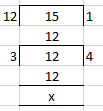Here dividing by 3 which is the HCF of 12 and 15

(12 ÷ 3)/ (15 ÷ 3) = 4/5

(ii) – 120/144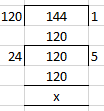Here dividing by 25 which is the HCF of -120 and 144

(-120 ÷ 24)/ (144 ÷ 24) = – 5/6

(iii) – 48/ – 72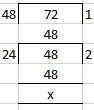Here dividing by 24 which is the HCF of -48 and – 72

(-48 ÷ 24)/ (-72 ÷ 24) = -2/-3 = 2/3

(iv) 14/-56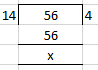Here dividing by 14 which is the HCF of 14 and – 56

(14 ÷ 14)/ (-56 ÷ 14) = 1/-4 or – 1/4

14. Express each of the following rational numbers in the standard form.

(i) -7/-8

(ii) 5/ – 12

(iii) – 7/ – 20

(iv) 4/ -9

Solution:

Here a rational number is in standard form if its denominator is positive in lowest term.

(i) -7/-8 = 7/8

(ii) 5/ – 12 = -5/12

(iii) – 7/ – 20 = 7/20

(iv) 4/ -9 = -4/9

#### Exercise 2B page: 25

1. Mark the following pairs of rational numbers on the separate number lines:

(i) 3/4 and -1/4

(ii) 2/5 and -3/5

(iii) 5/6 and -2/3

(iv) 2/5 and -4/5

(v) 1/4 and -5/4

Solution:

(i) 3/4 and -1/4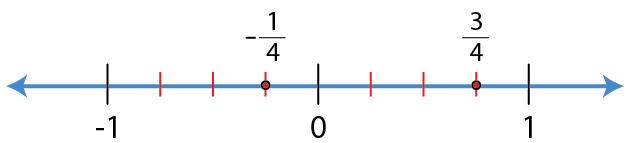(ii) 2/5 and -3/5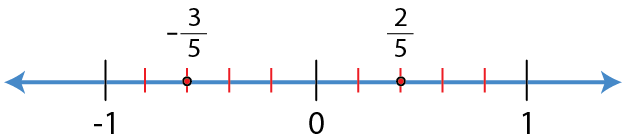(iii) 5/6 and -2/3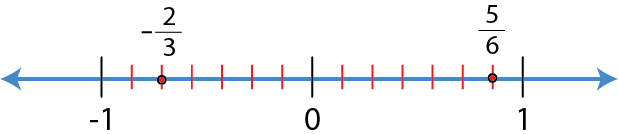(iv) 2/5 and -4/5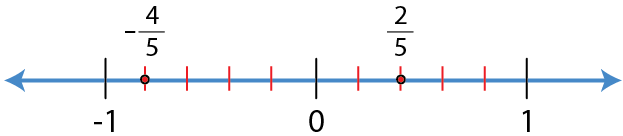(v) 1/4 and -5/4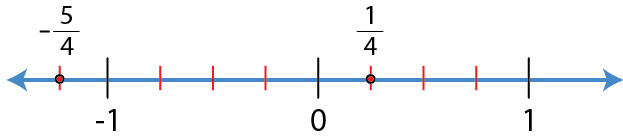2. Compare:

(i) 3/5 and 5/7

(ii) -7/2 and 5/2

(iii) -3 and 2 ¾

(iv) – 1 ½ and 0

(v) 0 and 3/4

(vi) 3 and -1

Solution:

(i) 3/5 and 5/7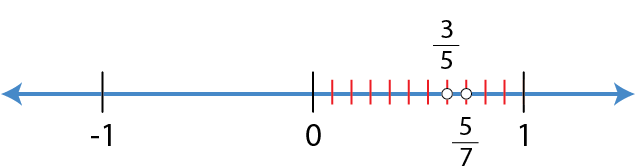5/7 is on the right side of the number line.

Hence, 3/5 < 5/7.

(ii) -7/2 and 5/2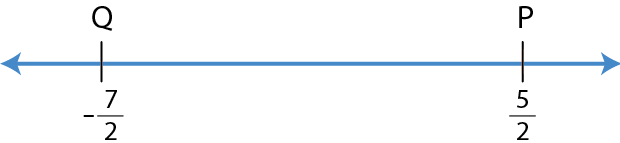P is on the right of Q

Hence, -7/2 < 5/2.

(iii) -3 and 2 ¾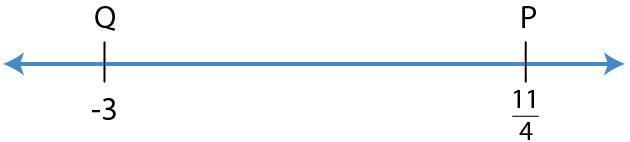P is on the right of Q

Hence, -3 < 11/4 or – 3 < 2 ¾.

(iv) – 1 ½ and 0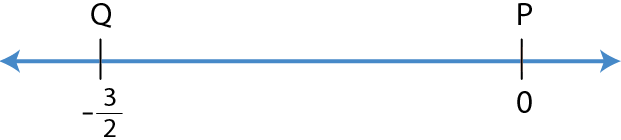P is on the right of Q

Hence, -3/2 < 0 or – 1 ½ < 0.

(v) 0 and ¾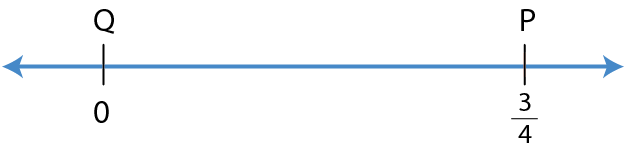P is on the right of Q

Hence, 0 < 3/4.

(vi) 3 and -1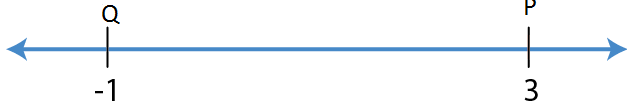P is on the right of Q

Hence, 3 > – 1.

3. Compare:

(i) -1/4 and 0

(ii) 1/4 and 0

(iii) -3/8 and 2/5

(iv) -5/8 and 7/-12

(v) 5/-9 and -5/-9

(vi) -7/8 and 5/-6

(vii) 2/7 and -3/-8

Solution:

(i) -1/4 and 0

-1/4 is a negative rational number which is always less than 0.

Hence, -1/4 < 0.

(ii) 1/4 and 0

1/4 is a positive rational number which is always greater than 0.

Hence, 1/4 > 0.

(iii) -3/8 and 2/5

We know that

a/b and c/d = a × d and b × c

So we get

a × d < b × c

Substituting the values

– 3 × 5 and 2 × 8

– 15 < 16

Hence, -3/8 < 2/5.

(iv) -5/8 and 7/-12

It can be written as

-5/ 8 and -7/12

We know that

a/b and c/d = a × d and b × c

So we get

a × d < b × c

Substituting the values

– 5 × 12 and – 7 × 8

-60 < – 56

Hence, -5/8 < 7/-12.

(v) 5/-9 and -5/-9

We know that

a/b and c/d = a × d and b × c

So we get

a × d < b × c

Substituting the values

5 × -9 and -5 × -9

– 45 < 45

Hence, 5/-9 < -5/-9.

(vi) -7/8 and 5/-6

It can be written as

-7/ 8 and -5/6

We know that

a/b and c/d = a × d and b × c

So we get

a × d < b × c

Substituting the values

– 7 × 6 and -5 × 8

-42 < -40

Hence, -7/8 < 5/-6.

(vii) 2/7 and -3/-8

It can be written as

2/7 and 3/8

We know that

a/b and c/d = a × d and b × c

So we get

a × d < b × c

Substituting the values

2 × 8 and 7 × 3

16 < 21

Hence, 2/7 < – 3/ -8.

4. Arrange the given rational numbers in ascending order:

(i) 7/10, -11/-30 and 5/-15

(ii) 4/-9, -5/12 and 2/-3

Solution:

(i) 7/10, -11/-30 and 5/-15

It is given that

= 7/10, -11/-30 and -5/-5

LCM of 10, 30 and 15 = 30

= (7 × 3)/ (10 × 3), 11/30 and (-5 × 2)/ (15 × 2)

So we get

= 21/30, 11/30 and -10/30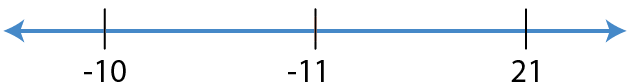Here, -10 < 11 < 21

We can write it as

-10/ 30 < 11/30 < 21/30

By further calculation

5/ -15 < -11/ -30 < 7/10

(ii) 4/-9, -5/12 and 2/-3

It is given that

= -4/9, -5/12 and -2/3

LCM of 9, 12 and 3 is 36

= (-4 × 4)/ (9 × 4), (-5 × 3)/ (12 × 3) and (-2 × 12)/ (3 × 12)

So we get

= -16/36, -15/36 and -24/36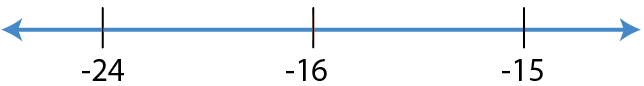Here, -24 < -16 < -15

We can write it as

-24/36 < -16/36 < -15/36

By further calculation

2/ -3 < 4/ -9 < -5/12

5. Arrange the given rational numbers in descending order:

(i) 5/8, 13/-16 and -7/12

(ii) 3/-10, -13/30 and 8/-20

Solution:

(i) 5/8, 13/-16 and -7/12

It can be written as

= 5/8, -13/16 and -7/12

LCM of 8, 16 and 12 is 48

= (5 × 6)/ (8 × 6), (-13 × 3)/ (16 × 3) and (-7 × 4)/ (12 × 4)

So we get

= 30/48, -39/48 and -28/48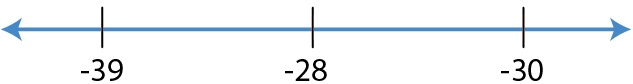Here, 30 > – 28 > – 39

We can write it as

30/48 > -28/48 > -39/48

By further calculation

5/8 > -7/12 > 13/-16

(ii) 3/-10, -13/30 and 8/-20

It can be written as

= -3/10, -13/30 and -8/20

LCM of 10, 20 and 30 is 60

= (- 3 × 6)/ (10 × 6), (-13 × 2)/ (30 × 2) and (-8 × 3)/ (20 × 3)

So we get

= -18/60, -26/60 and -24/60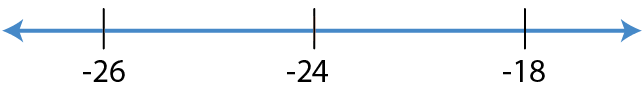Here, -18 > -24 > -26

We can write it as

-18/60 > -24/60 > -26/60

By further calculation

3/ -10 > 8/-20 > -13/30

6. Fill in the blanks:

(i) 5/8 and 3/10 are on the ……. side of zero.

(ii) -5/8 and 3/10 are on the …….. sides of zero.

(iii) -5/8 and -3/10 are on the ……. side of zero.

(iv) 5/8 and -3/10 are on the …….. sides of zero.

Solution:

(i) 5/8 and 3/10 are on the same side of zero.

(ii) -5/8 and 3/10 are on the opposite sides of zero.

(iii) -5/8 and -3/10 are on the same side of zero.

(iv) 5/8 and -3/10 are on the opposite sides of zero.

#### Exercise 2C page: 30

(i) 7/5 and 2/5

(ii) -4/9 and 2/9

(iii) 5/-12 and 1/12

(iv) 4/-15 and -7/-15

(v) -7/25 and 9/-25

(vi) -7/26 and 7/-26

Solution:

(i) 7/5 and 2/5

It can be written as

= 7/5 + 2/5

By further calculation

= (7 + 2)/ 5

= 9/5

= 1 4/5

(ii) -4/9 and 2/9

It can be written as

= -4/9 + 2/9

By further calculation

= (-4 + 2)/ 9

= -2/9

(iii) 5/-12 and 1/12

It can be written as

= -5/12 + 1/12

By further calculation

= (-5 + 1)/ 12

= -4/ 12

= -1/3

(iv) 4/-15 and -7/-15

It can be written as

= – 4/15 + 7/15

By further calculation

= (-4 + 7)/ 15

= 3/15

= 1/5

(v) -7/25 and 9/-25

It can be written as

= -7/25 + -9/25

By further calculation

= [(-7) + (-9)]/ 25

= -16/25

(vi) -7/26 and 7/-26

It can be written as

= – 7/26 + -7/26

By further calculation

= [(-7) + (-7)]/ 26

= – 14/26

= -7/13

(i) -2/5 and 3/7

(ii) -5/6 and 4/9

(iii) – 3 and 2/3

(iv) -5/9 and 7/18

(v) -7/24 and -5/48

(vi) 1/-18 and 5/-27

(vii) -9/25 and 1/-75

(viii) 13/-16 and -11/24

(ix) -9/-16 and -11/8

Solution:

(i) -2/5 and 3/7

It can be written as

= (-2 × 7)/ (5 × 7) + (3 × 5)/ (7 × 5)

LCM of 5 and 7 is 35

= -14/35 + 15/35

By further calculation

= (-14 + 15)/35

= 1/35

(ii) -5/6 and 4/9

It can be written as

= -5/6 + 4/9

LCM of 6 and 9 is 36

= (-5 × 6)/ (6 × 6) + (4 × 4)/ (9 × 4)

By further calculation

= -30/36 + 16/36

So we get

= (-30 + 16)/ 36

= -14/36

= – 7/18

(iii) – 3 and 2/3

It can be written as

= -3/1 + 2/3

LCM of 1 and 3 is 3

= (- 3 × 3)/ (1 × 3) + (2 × 1)/ (3 × 1)

By further calculation

= -9/3 + 2/3

So we get

= (-9 + 2)/ 3

= -7/3

(iv) -5/9 and 7/18

It can be written as

= -5/9 + 7/18

LCM of 9 and 18 is 18

= (-5 × 2)/ (9 × 2) + (7 × 1)/ (18 × 1)

By further calculation

= -10/18 + 7/18

So we get

= (-10 + 7)/ 18

= – 3/18

= -1/6

(v) -7/24 and -5/48

It can be written as

= -7/24 + -5/48

LCM of 24 and 48 is 48

= (-7 × 2)/ (24 × 2) + (-5 × 1)/ (48 × 1)

By further calculation

= -14/48 + -5/48

So we get

= (-14 – 5)/ 48

= – 19/48

(vi) 1/-18 and 5/-27

It can be written as

= – 1/18 + -5/27

LCM of 18 and 27 is 54

= (-1 × 3)/ (18 × 3) + (-5 × 2)/ (27 × 2)

By further calculation

= -3/54 + -10/54

So we get

= (- 3 – 10)/ 54

= -13/54

(vii) -9/25 and 1/-75

It can be written as

= -9/25 + -1/75

LCM of 24 and 75 is 75

= (-9 × 3)/ (25 × 3) + (-1 × 1)/ (75 × 1)

By further calculation

= -27/75 + -1/75

So we get

= (-27 – 1)/ 75

= -28/75

(viii) 13/-16 and -11/24

It can be written as

= -13/16 + -11/24

LCM of 16 and 24 is 48

= (-13 × 3)/ (16 × 3) + (-11 × 2)/ (24 × 2)

By further calculation

= -39/48 + -22/48

So we get

= (-39 – 22)/ 48

= -61/48

(ix) -9/-16 and -11/8

It can be written as

= 9/16 + -11/8

LCM of 16 and 8 is 16

= (9 × 1)/ (16 × 1) + (-11 × 2)/ (8 × 2)

By further calculation

= 9/16 + -22/16

So we get

= (9 – 22)/ 16

= -13/16

3. Evaluate:

(i) -2/5 + 3/5 + -1/5

(ii) -8/9 + 4/9 + -2/9

(iii) 5/-24 + -1/8 + 3/16

(iv) -7/6 + 4/-15 + -4/-30

(v) -2 + 2/5 + -2/15

(vi) -11/12 + 5/16 + -3/8

Solution:

(i) -2/5 + 3/5 + -1/5

It can be written as

= (-2 + 3 – 1)/ 5

By further calculation

= 0/5

= 0

(ii) -8/9 + 4/9 + -2/9

It can be written as

= (-8 + 4 – 2)/ 9

By further calculation

= (-10 + 4)/ 9

= -6/9

= -2/3

(iii) 5/-24 + -1/8 + 3/16

It can be written as

= -5/24 + -1/8 + 3/16

LCM of 8, 16 and 24 is 48

= (-5 × 2)/ (24 × 2) + (-1 × 6)/ (8 × 6) + (3 × 3)/ (16 × 3)

By further calculation

= -10/ 48 + -6/48 + 9/48

So we get

= (-10 – 6 + 9)/ 48

= (-16 + 9)/ 48

= -7/48

(iv) -7/6 + 4/-15 + -4/-30

It can be written as

= -7/6 + -4/15 + 4/30

LCM of 6, 15 and 30 is 30

= (-7 × 5)/ (6 × 5) + (-4 × 2)/ (15 × 2) + (4 × 1)/ (30 × 1)

By further calculation

= -35/30 + -8/30 + 4/30

So we get

= (-35 – 8 + 4)/ 30

= (-43 + 4)/ 30

= – 39/30

= -13/10

(v) -2 + 2/5 + -2/15

It can be written as

= -2/1 + 2/5 + -2/15

LCM of 1, 5 and 15 is 15

= (-2 × 15)/ (1 × 15) + (2 × 3)/ (5 × 3) + (-2 × 1)/ (15 × 1)

By further calculation

= -30/15 + 6/15 + -2/15

So we get

= (-30 + 6 – 2)/ 15

= (-32 + 6)/ 15

= -26/15

(vi) -11/12 + 5/16 + -3/8

It can be written as

= -11/12 + 5/16 + -3/8

LCM of 12, 16 and 8 is 48

= (-11 × 4)/ (12 × 4) + (5 × 3)/ (16 × 3) + (-3 × 6)/ (8 × 6)

By further calculation

= -44/48 + 15/48 + -18/48

So we get

= (-44 + 15 – 18)/ 48

= (-62 + 15)/ 48

= -47/ 48

4. Evaluate:

(i) -11/18 + -3/9 + 2/-3

(ii) -9/4 + 13/3 +25/6

(iii) – 5 + 5/-8 + -5/-12

(iv) -2/3 + 5/2 + 2

(v) 5 + -3/4 + -5/8

Solution:

(i) -11/18 + -3/9 + 2/-3

It can be written as

= – 11/18 + -3/9 + -2/3

LCM of 3, 9 and 18 is 18

= (-11 × 1)/ (18 × 1) + (-3 × 2)/ (9 × 2) + (-2 × 6)/ (3 × 6)

By further calculation

= -11/18 + -6/18 + -12/18

So we get

= (-11 – 6 – 12)/18

= -29/18

(ii) -9/4 + 13/3 +25/6

It can be written as

= -9/4 + 13/3 + 25/6

LCM of 4, 3 and 6 is 24

= (-9 × 6)/ (4 × 6) + (13 × 8)/ (3 × 8) + (25 × 4)/ (6 × 4)

By further calculation

= -54/24 + 104/24 + 100/24

So we get

= (-54 + 104 + 100)/ 24

= 150/24

= 25/4

= 6 1/4

(iii) – 5 + 5/-8 + -5/-12

It can be written as

= -5/1 + -5/8 + 5/12

LCM of 1, 8 and 12 is 24

= (-5 × 24)/ (1 × 24) + (-5 × 3)/ (8 × 3) + (5 × 2)/ (12 × 2)

By further calculation

= -120/24 + -15/24 + 10/24

So we get

= (-120 – 15 + 10)/24

= -125/24

(iv) -2/3 + 5/2 + 2

It can be written as

= -2/3 + 5/2 + 2/1

LCM of 3, 2 and 1 is 6

= (-2 × 2)/ (3 × 2) + (5 × 3)/ (2 × 3) + (2 × 6)/ (1 × 6)

By further calculation

= -4/6 + 15/6 + 12/6

So we get

= (-4 + 15 + 12)/ 6

= 23/6

= 3 5/6

(v) 5 + -3/4 + -5/8

It can be written as

= 5/1 + -3/4 + -5/8

LCM of 1, 4 and 8 is 8

= (5 × 8)/ (1 × 8) + (-3 × 2)/ (4 × 2) + (-5 × 1)/ (8 × 1)

By further calculation

= 40/8 + -6/8 + -5/8

So we get

= (40 – 6 – 5)/ 8

= (40 – 11)/8

= 29/8

= 3 5/8

5. Subtract:

(i) 2/9 from 5/9

(ii) -6/11 from -3/-11

(iii) -2/15 from -8/15

(iv) 11/18 from -5/18

(v) -4/11 from -2

Solution:

(i) 2/9 from 5/9

It can be written as

= 5/9 – 2/9

By further calculation

= (5 – 2)/ 9

= 3/9

= 1/3

(ii) -6/11 from -3/-11

It can be written as

= 3/ 11 – (-6/11)

By further calculation

= 3/11 + 6/11

So we get

= (3 + 6)/ 11

= 9/11

(iii) -2/15 from -8/15

It can be written as

= -8/15 – (-2/15)

By further calculation

= -8/15 + 2/15

So we get

= (-8 + 2)/ 15

= -6/ 15

= -2/5

(iv) 11/18 from -5/18

It can be written as

= -5/18 – 11/18

By further calculation

= (-5 – 11)/ 18

So we get

= -16/18

= -8/9

(v) -4/11 from -2

It can be written as

= -2/1 – (-4/11)

LCM of 1 and 11 is 11

= (- 2 × 11)/ (1 × 11) + (4 × 1)/ (11 × 1)

By further calculation

= -22/11 + 4/11

So we get

= (-22 + 4)/ 11

= -18/11

6. Subtract:

(i) -3/10 from 1/5

(ii) -6/25 from -8/5

(iii) -7/4 from -2

(iv) -16/21 from 1

(v) -8/15 from 0

(vi) 0 from -3/8

(vii) -2 from -3/10

(viii) 5/8 from -5/16

(ix) 4 from -3/13

Solution:

(i) -3/10 from 1/5

It can be written as

= 1/5 – (-3/10)

LCM of 5 and 10 is 10

= (1 × 2)/ (5 × 2) + 3/10

By further calculation

= 2/10 + 3/10

So we get

= (2 + 3)/ 10

= 5/10

= 1/2

(ii) -6/25 from -8/5

It can be written as

= -8/5 – (-6/25)

LCM of 5 and 25 is 25

= (-8 × 5)/ (5 × 5) + 6/25

By further calculation

= -40/25 + 6/25

So we get

= (-40 + 6)/ 25

= -34/25

(iii) -7/4 from -2

It can be written as

= (-2/1) – (-7/4)

LCM of 1 and 4 is 4

= (-2 × 4)/ (1 × 4) + 7/4

= -8/4 + 7/4

By further calculation

= (-8 + 7)/ 4

= -1/4

(iv) -16/21 from 1

It can be written as

= 1/1 – (-16/21)

= 1/1 + 16/21

By further calculation

= (21 + 16)/ 21

So we get

= (21 + 16)/21

= 37/21

= 1 16/21

(v) -8/15 from 0

It can be written as

= 0 – (-8/15)

By further calculation

= 0 + 8/15

= 8/15

(vi) 0 from -3/8

It can be written as

= -3/8 – 0

= -3/8

(vii) -2 from -3/10

It can be written as

= -3/10 – (-2/1)

By further calculation

= -3/10 + 2/1

So we get

= (-3 + 2 × 10)/10

= 17/10

= 1 7/10

(viii) 5/8 from -5/16

It can be written as

= -5/16 – 5/8

LCM of 8 and 16 is 16

= -5/16 – (5 × 2)/ (8 × 2)

By further calculation

= -5/16 – 10/16

So we get

= (-5 – 10)/16

= – 15/16

(ix) 4 from -3/13

It can be written as

= 3/13 – 4/1

LCM of 13 and 1 is 13

= (- 3 – 4 × 13)/ 13

By further calculation

= (-3 – 52)/13

= -55/13

7. The sum of two rational numbers is 11/24. If one of them is 3/8, find the other.

Solution:

It is given that

Sum of two rational numbers = 11/24

One of the rational number = 3/8

Other rational number = 11/24 – 3/8

LCM of 24 and 8 is 24

= 11/24 – (3 × 3)/ (8 × 3)

By further calculation

= 11/24 – 9/24

So we get

= (11 – 9)/ 24

= 2/24

= 1/12

8. The sum of two rational numbers is -7/12. If one of them is 13/24, find the other.

Solution:

It is given that

Sum of two rational numbers = -7/12

One of the rational number = 13/24

Other rational number = -7/12 – 13/24

LCM of 12 and 24 is 24

= (-7 × 2)/ (12 × 2) – 13/24

By further calculation

= -14/24 – 13/24

So we get

= (-14 – 13)/ 24

= -27/24

= -9/8

9. The sum of two rational numbers is -4. If one of them is -13/12, find the other.

Solution:

It is given that

Sum of two rational numbers = -4

One of the rational number = -13/12

Other rational number = – 4 – (-13/12)

LCM of 1 and 12 is 12

= – 4 + 13/12

By further calculation

= (-4 × 12 + 13)/ 12

So we get

= (-48 + 13)/ 12

= -35/12

10. What should be added to -3/16 to get 11/24?

Solution:

Consider x as the required rational number

Other number = -3/16

Sum of two numbers = 11/24

From the question

-3/16 + x = 11/24

By further calculation

x = 11/24 + 3/16

LCM of 16 and 24 is 48

x = (11 × 2)/ (24 × 2) + (3 × 3)/ (16 × 3)

So we get

x = 22/48 + 9/48

x = (22 + 9)/ 48 = 31/48

11. What should be added to -3/5 to get 2?

Solution:

Consider x as the required rational number

Other number = -3/5

Here the sum of two numbers is 2

From the question

-3/5 + x = 2

By further calculation

x = 2 + 3/5

LCM of 1 and 5 is 5

x = (2 × 5 + 3)/ 5

So we get

= (10 + 3)/ 5

= 13/5

= 2 3/5

12. What should be subtracted from -4/5 to get 1?

Solution:

Consider x as the required rational number

Other number = -4/5

Here the difference between two numbers is 1

From the question

-4/5 – x = 1

By further calculation

-4/5 – 1 = x

So we get

x = (-4 – 1 × 5)/ 5

x = (-4 – 5)/ 5 = -9/5

13. The sum of two numbers is -6/5. If one of them is -2, find the other.

Solution:

It is given that

Sum of two numbers = -6/5

One of the numbers = -2

Other number = -6/5 – (-2/1)

LCM of 1 and 5 is 5

= – 6/5 – (2 × 5)/ (1 × 5)

By further calculation

= (-6 + 10)/ 5

= 4/5

14. What should be added to -7/12 to get 3/8?

Solution:

Consider x as the required rational number

Other rational number = -7/12

Sum of two numbers = 3/8

Using the question

-7/12 + x = 3/8

So we get

x = 3/8 – (-7/12)

LCM of 8 and 12 is 24

x = (3 × 3)/ (8 × 3) + (7 × 2)/ (12 × 2)

By further calculation

= 9/24 + 14/24

So we get

= (9 + 14)/ 24 = 23/34

15. What should be subtracted from 5/9 to get 9/5?

Solution:

Consider x as the first number

Other number is 5/9

Here the difference between two numbers is 9/5

Using the question

5/9 – x = 9/5

So we get

x = 5/9 – 9/5

LCM of 9 and 5 is 45

x = (5 × 5)/ (9 × 5) – (9 × 9)/ (5 × 9)

By further calculation

x = 25/45 – 81/45

x = (25 – 81)/ 45 = – 56/45

#### Exercise 2D page: 34

1. Evaluate:

(i) 5/4 × 3/7

(ii) 2/3 × -6/7

(iii) (-12/5) × (10/-3)

(iv) -45/39 × -13/ 15

(v) 3 1/8 × (-2 2/5)

(vi) 2 14/25 × (-5/16)

(vii) (-8/9) × (-3/ 16)

(viii) (5/-27) × (-9/ 20)

Solution:

(i) 5/4 × 3/7

It can be written as

= (5 × 3)/ (4 × 7)

= 15/28

(ii) 2/3 × -6/7

It can be written as

= (2 × -6)/ (3 × 7)

By further calculation

= (2 × -2)/ 7

= -4/7

(iii) (-12/5) × (10/-3)

It can be written as

= (-12 × 10)/ (5 × -3)

By further calculation

= 4 × 2

= 8

(iv) -45/39 × -13/ 15

It can be written as

= (-45 × -13)/ (39 × 15)

By further calculation

= (-3 × -1)/ (3 × 1)

So we get

= 3/3

= 1

(v) 3 1/8 × (-2 2/5)

It can be written as

= (3 × 8 + 1)/ 8 × (-2 × 5 + 2)/ 5

By further calculation

= 25/8 × (-12/5)

So we get

= (25 × -12)/ (8 × 5)

On further simplification

= (5 × -3)/ (2 × 1)

= -15/2

(vi) 2 14/25 × (-5/16)

It can be written as

= (2 × 25 + 14)/ 25 × (-5/16)

By further calculation

= 64/25 × (-5/16)

= (64 × -5)/ (25 × 16)

On further simplification

= (4 × -1)/ (5 × 1)

= -4/5

(vii) (-8/9) × (-3/ 16)

It can be written as

= (-8 × -3)/ (9 × 16)

By further calculation

= (-1 × -1)/ (3 × 2)

= 1/6

(viii) (5/-27) × (-9/ 20)

It can be written as

= (5 × -9)/ (-27 × 20)

By further calculation

= (1 × 1)/ (3 × 4)

= 1/12

2. Multiply:

(i) 3/25 and 4/5

(ii) 1 1/8 and 10 2/3

(iii) 6 2/3 and -3/8

(iv) -13/15 and -25/26

(v) 1 1/6 and 18

(vi) 2 1/14 and -7

(vii) 5 1/8 and -16

(viii) 35 and -18/25

(ix) 6 2/3 and -3/8

(x) 3 3/5 and -10

(xi) 27/28 and -14

(xii) -24 and 5/16

Solution:

(i) 3/25 and 4/5

It can be written as

= 3/25 × 4/5

By further calculation

= (3 × 4)/ (25 × 5)

= 12/125

(ii) 1 1/8 and 10 2/3

It can be written as

= 9/8 × 32/2

By further calculation

= (9 × 32)/ (8 × 3)

= 3 × 4

= 12

(iii) 6 2/3 and -3/8

It can be written as

= 20/3 × -3/8

By further calculation

= (20 × -3)/ (3 × 8)

So we get

= (5 × -1)/ (1 × 2)

= -5/2

(iv) -13/15 and -25/26

It can be written as

= (-13 × -25)/ (15 × 26)

By further calculation

= (-1 × -5)/ (3 × 2)

= 5/6

(v) 1 1/6 and 18

It can be written as

= 7/6 × 18

By further calculation

= 7 × 3

= 21

(vi) 2 1/14 and -7

It can be written as

= (2 × 14 + 1)/ 14 × (-7)

By further calculation

= 29/4 × (-7)

So we get

= (29 × -1)/ 2

= -29/2

(vii) 5 1/8 and -16

It can be written as

= 41/8 × -16

By further calculation

= 41 × -2

= -82

(viii) 35 and -18/25

It can be written as

= 35 × -18/25

By further calculation

= (35 × -18)/ 25

So we get

= (7 × -18)/ 5

= -126/5

(ix) 6 2/3 and -3/8

It can be written as

= 20/3 × -3/8

By further calculation

= (20 × -3)/ (3 × 8)

So we get

= (5 × -1)/ (1 × 2)

= -5/2

(x) 3 3/5 and -10

It can be written as

= (3 × 5 + 3)/ 5 × -10

By further calculation

= 18/5 × -10

So we get

= 18 × -2

= -36

(xi) 27/28 and -14

It can be written as

= 27/28 × -14

By further calculation

= (27 × -1)/ 2

= -27/2

(xii) -24 and 5/16

It can be written as

= (-24 × 5)/ 16

By further calculation

= (-3 × 5)/ 2

So we get

= -15/2

3. Evaluate:

(i) (6 × 5/18) – (- 4 2/9)

(ii) (7/8 × 8/7) + (-5/9) × (6/-25)

(iii) (11/-9 × 21/44) + (-5/9) × (63/ -100)

(iv) (-5/9 × 6/-25) + (24/21 × 7/8)
(v) (-35/39 × -13/7) – (7/90 × -18/14)

(vi) (-4/5 × 3/2) + (9/-5 × 10/3) – (-3/2 × -1/4)

Solution:

(i) (6 × 5/18) – (- 4 2/9)

It can be written as

= (-1 × 5/3) – [- (4 × 9 + 2)/ 9]

LCM of 3 and 9 is 9

= -5/3 – (-38/9)

So we get

= -5/3 + 38/9

By further calculation

= (-5 × 3)/ (3 × 3) + (38 × 1)/ (9 × 1)

= (-15 + 38)/ 9

= 23/9

= 2 5/9

(ii) (7/8 × 8/7) + (-5/9) × (6/-25)

It can be written as

= (7/8 × 8/7) + (-5/9 × 6/-25)

By further calculation

= 1/1 + (1 × 2)/ (3 × 5)

So we get

= 1/1 + 2/15

= (15 + 2)/ 15

= 17/15

= 1 2/15

(iii) (11/-9 × 21/44) + (-5/9) × (63/ -100)

It can be written as

= (11/-9 × 21/44) + (5/9 × 63/100)

By further calculation

= (-1 × 7)/ (3 × 4) + (1 × 7)/ (1 × 20)

So we get

= -7/12 + 7/20

LCM of 12 and 20 is 60

= (-7 × 5)/ (12 × 5) + (7 × 3)/ (20 × 3)

Here

= -35/60 + 211/60

= (-35 + 21)/ 60

= -14/60

= -7/30

(iv) (-5/9 × 6/-25) + (24/21 × 7/8)

It can be written as

= (5/9 × 6/25) + (24/21 × 7/8)

By further calculation

= 2/ (3 × 5) + 1

= 2/15 + 1

LCM of 15 and 1 is 15

= (2 + 15)/ 15

= 17/15

= 1 2/15

(v) (-35/39 × -13/7) – (7/90 × -18/14)

It can be written as

= (-35/39 × -13/7) – (7/90 × -18/14)

By further calculation

= (-5 × -1)/ (3 × 1) – (1 × -1)/ (5 × 2)

So we get

= 5/3 – (-1/10)

LCM of 3 and 10 is 30

= (5 × 10)/ (3 × 10) + 1/ (10 × 3)

We get

= (50 + 3)/ 30

= 53/30

= 1 23/30

(vi) (-4/5 × 3/2) + (9/-5 × 10/3) – (-3/2 × -1/4)

It can be written as

= (-2 × 3)/ (5 × 1) + (3 × 2)/ (-1 × 1) – (-3 × -1)/ (2 × 4)

By further calculation

= -6/5 + -6/1 – 3/8

LCM of 5, 1 and 8 is 40

= = (-6 × 8)/ (5 × 8) – (6 × 40)/ (1 × 40) – (3 × 5)/ (8 × 5)

So we get

= (-48 – 240 – 15)/ 40

= – 303/40

4. Find the cost of 3 ½ m cloth, if one metre cloth costs ₹ 325 ½.

Solution:

It is given that cost of one metre cloth = ₹ 325 ½

We can write it as

= (2 × 325 + 1)/ 2

By further calculation

= (650 + 1)/ 2

= ₹ 651/2

Cost of 3 ½ m cloth

(2 × 3 + 1)/ 2 = 7/2 m

We get

= 651/2 × 7/2

It can be written as

= (651 × 7)/ (2 × 2)

= 4557/4

= ₹ 1139 ¼

5. A bus is moving with a speed of 65 ½ km per hour. How much distance will it cover in 1 1/3 hours.

Solution:

It is given that

Speed of bus = 65 ½ km per hour

We can write it as

= (2 × 65 + 1)/ 2

By further calculation

= (130 + 1)/ 2

= 131/ 2 km

Distance covered in 1 1/3 hour = 4/3 hour can be written as

= 131/2 × 4/3

We get

= 131/1 × 2/3

We know that distance covered = speed × time

= 131/2 × 4/3

= (131 × 2)/ (1 × 3)

So we get

= 262/3

= 87 1/3 km

6. Divide:

(i) 15/28 by ¾

(ii) -20/9 by -5/9

(iii) 16/-5 by -8/7

(iv) -7 by -14/5

(v) -14 by 7/-2

(vi) -22/9 by 11/18

(vii) 35 by -7/9

(viii) 21/44 by -11/9

Solution:

(i) 15/28 by ¾

We know that

= 15/28 ÷ 3/4

It can be written as

= 15/28 × 4/3

By further calculation

= 5/7 × 1/1

= 5/7

(ii) -20/9 by -5/9

We know that

= -20/9 ÷ -5/9

It can be written as

= -20/9 × 9/-5

By further calculation

= -4/-1

= 4

(iii) 16/-5 by -8/7

We know that

= 16/-5 ÷ -8/7

It can be written as

= 16/-5 × 7/-8

By further calculation

= 2/-5 × 7/-1

= (2 × 7)/ (-5 × -1)

So we get

= 14/5

= 2 4/5

(iv) -7 by -14/5

We know that

= -7 ÷ – 14/5

It can be written as

= -7 × 5/-14

By further calculation

= 1 × 5/2

= (1 × 5)/ 2

= 5/2

= 2 ½

(v) -14 by 7/-2

We know that

= -14 ÷ 7/-2

It can be written as

= -14 × -2/7

By further calculation

= (-2 × -2)/ (1 × 1)

= 4

(vi) -22/9 by 11/18

We know that

= – 22/9 ÷ 11/18

It can be written as

= -22/9 × 18/11

By further calculation

= -2/1 × 2/1

= (-2 × 2)/ (1 × 1)

= – 4/1

= – 4

(vii) 35 by -7/9

We know that

= 35 ÷ -7/9

It can be written as

= 35 × 9/-7

By further calculation

= 5 × 9/-1

So we get

= (5 × 9)/ -1

= 45/-1

= -45

(viii) 21/44 by -11/9

We know that

= 21/44 ÷ -11/9

It can be written as

= 21/44 × -9/11

By further calculation

= (21 × -9)/ (44 × 11)

= -189/484

7. Evaluate:

(i) 3 5/12 + 1 2/3

(ii) 3 5/12 – 1 2/3

(iii) (3 5/12 + 1 2/3) ÷ (3 5/12 – 1 2/3)

Solution:

(i) 3 5/12 + 1 2/3

It can be written as

= (12 × 3 + 5)/ 12 + (3 × 1 + 2)/ 3

= 41/12 + 5/3

LCM of 12 and 3 is 12

= (41 × 1)/ (12 × 1) + (5 × 4)/ (3 × 4)

By further calculation

= 41/12 + 20/12

= (41 + 20)/ 12

= 61/12

= 5 1/12

(ii) 3 5/12 – 1 2/3

It can be written as

= (12 × 3 + 5)/ 12 – (3 × 1 + 2)/ 3

= 41/12 – 5/3

LCM of 12 and 3 is 12

= (41 × 1)/ (12 × 1) – (5 × 4)/ (3 × 4)

By further calculation

= (41 – 20)/ 12

= 21/12

= 2/4

= 1 ¾

(iii) (3 5/12 + 1 2/3) ÷ (3 5/12 – 1 2/3)

It can be written as

= [(12 × 3 + 5)/ 12 + (3 × 1 + 2)/ 3] ÷ [(12 × 3 + 5)/ 12 – (3 × 1 + 2)/ 3]

= (41/12 + 5/3) ÷ (41/12 – 5/3)

LCM of 12 and 3 is 12

= (41 + 20)/ 12 ÷ (41 – 20)/ 12

By further calculation

= 61/12 ÷ 21/12

We can write it as

= 61/12 × 12/21

= 61/21

= 2 19/21

8. The product of two numbers is 14. If one of the numbers is -8/7, find the other.

Solution:

It is given that

Product of two numbers = 14

One of the number = -8/7

Other number = 14 ÷ -8/7

We can write it as

= 14 × -7/8

= -98/8

= – 49/4

9. The cost of 11 pens is ₹ 24 ¾. Find the cost of one pen.

Solution:

It is given that

Cost of 11 pens = ₹ 24 ¾

We can write it as

= (24 × 4 + 3)/ 4

= ₹ 99/4

So the cost of one pen = 99/4 ÷ 11

It can be written as

= 99/4 × 1/11

= ₹ 9/4

= ₹ 2 ¼

10. If 6 identical articles can be bought for ₹ 2 6/17. Find the cost of each article.

Solution:

It is given that

Cost of 6 articles = ₹ 2 6/17

We can write it as

= (2 × 17 + 6)/ 17

= ₹ 40/17

So the cost of each article = 40/17 ÷ 6

It can be written as

= 40/17 × 1/6

= ₹ 20/51

11. By what number should -3/8 be multiplied so that the product is -9/16?

Solution:

Number = -3/8 ÷ (-9/16)

We can write it as

= -3/8 × 16/-9

By further calculation

= 2/3

= 1 ½

12. By what number should -5/7 be divided so that the result is -15/28?

Solution:

Consider the number as x

-5/7 ÷ x = -15/28

It can be written as

-5/7 × 1/x = -15/28

By further calculation

-5/7x = -15/28

So we get

x = 5/7 × 28/15 = 4/3

x = 1 1/3

13. Evaluate: (32/15 + 8/5) ÷ (32/15 – 8/5).

Solution:

It is given that

(32/15 + 8/5) ÷ (32/15 – 8/5)

LCM of 15 and 5 is 15

= [(32 × 1)/ (15 × 1) + (8 × 3)/ (5 × 3)] ÷ [(32 × 1)/ (15 × 1) – (8 × 1)/ (5 × 1)]

By further calculation

= (32 + 24)/ 15 ÷ (32 – 24)/ 15

So we get

= 56/15 ÷ 8/15

= 56/15 × 15/8

= 7

14. Seven equal pieces are made out of a rope of 21 5/7 m. Find the length of each piece.

Solution:

It is given that

Length of 7 pieces of rope = 21 5/7 m

It can be written as

= (21 × 7 + 5)/ 7

= 152/7

So the length of each piece = 152/7 ÷ 7

We can write it as

= 152/7 × 1/7

So we get

= 152/49

= 3 5/49 m

#### Exercise 2E page: 36

1. Evaluate:

(i) -2/3 + 3/4

(ii) 7/-27 + 11/18

(iii) -3/8 + -5/12

(iv) 9/-16 + -5/-12

(v) -5/9 + -7/12 + 11/18

(vi) 7/-26 + 16/39

(vii) -2/3 – (-5/7)

(viii) -5/7 – (-3/8)

(ix) 7/26 + 2 + -11/13

(x) -1 + 2/-3 + 5/6

Solution:

(i) -2/3 + 3/4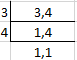Here the LCM of 3 and 4 is 12

So we get

= (-2 × 4)/ (3 × 4) + (3 × 3)/ (4 × 3)

By further calculation

= (-8 + 9)/ 12

= 1/12

(ii) 7/-27 + 11/18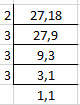Here the LCM of 27 and 18 is 54

So we get

= (7 × 2)/ (-27 × 2) + (11 × 3)/ (18 × 3)

By further calculation

= (-14 + 33)/ 54

= 19/54

(iii) -3/8 + -5/12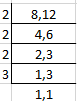Here LCM of 8 and 12 is 24

So we get

= (-3 × 3)/ (8 × 3) + (-5 × 2)/ (12 × 2)

By further calculation

= (-9 – 10)/ 24

= -19/24

(iv) 9/-16 + -5/-12

It can be written as

= 9/-16 + 5/12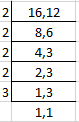Here LCM of 16 and 12 is 48

So we get

= (9 × 3)/ (-16 × 3) + (5 × 4)/ (12 × 4)

By further calculation

= (-27 + 20)/48

= -7/48

(v) -5/9 + -7/12 + 11/18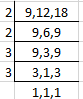Here LCM of 9, 12 and 18 is 36

So we get

= (-5 × 4)/ (9 × 4) – (7 × 3)/ (12 × 3) + (11 × 2)/ (18 × 2)

By further calculation

= (-20 – 21 + 22)/ 36

So we get

= (-41 + 22)/ 36

= -19/36

(vi) 7/-26 + 16/39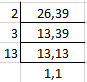Here LCM of 26 and 39 is 78

So we get

= (-7 × 3)/ (26 × 3) + (16 × 2)/ (39 × 2)

By further calculation

= (-21 + 32)/ 78

= 11/78

(vii) -2/3 – (-5/7)

It can be written as

= -2/3 + 5/7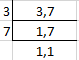Here LCM of 3 and 7 is 21

So we get

= (-2 × 7)/ (3 × 7) + (5 × 3)/ (7 × 3)

By further calculation

= (-14 + 15)/ 21

= 1/21

(viii) -5/7 – (-3/8)

It can be written as

= -5/7 + 3/8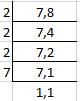Here LCM of 7 and 8 is 56

So we get

= (-5 × 8)/ (7 × 8) + (3 × 7)/ (8 × 7)

By further calculation

= (-40 + 21)/ 56

= -19/56

(ix) 7/26 + 2 + -11/13

It can be written as

= 7/26 + 2/1 + -11/13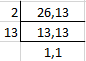Here LCM of 26 and 13 is 26

So we get

= (7 × 1)/ (26 × 1) + (2 × 26)/ (1 × 26) – (11 × 2)/ (13 × 2)

By further calculation

= (7 + 52 – 22)/ 26

So we get

= (59 – 22)/ 26

= 37/26

= 1 11/26

(x) -1 + 2/-3 + 5/6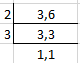Here LCM of 3 and 6 is 6

So we get

= (-1 × 6)/ (1 × 6) – (2 × 2)/ (3 × 2) + (5 × 1)/ (6 × 1)

By further calculation

= (-6 – 4 + 5)/ 6

We get

= (-10 + 5)/ 6

= -5/6

2. The sum of two rational numbers is -3/8. If one of them is 3/16, find the other.

Solution:

It is given that

Sum of two rational numbers = -3/8

One rational number = 3/16

Other rational number = -3/8 – 3/16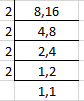Here LCM of 8 and 16 is 16

So we get

= (-3 × 2)/ (8 × 2) – (3 × 1)/ (16 × 1)

By further calculation

= (-6 – 3)/ 16

= -9/16

3. The sum of two rational numbers is -5. If one of them is -52/25, find the other.

Solution:

It is given that

Sum of two rational numbers = -5

One rational number = -52/25

Other rational number = – 5 – (-52/25)

Here LCM is 25

= (-5 × 25)/ (1 × 25) + (52 × 1)/ (25 × 1)

By further calculation

= (-125 + 52)/ 25

= – 73/25

4. What rational number should be added to -3/16 to get 11/24?

Solution:

It is given that

Sum of two rational numbers = 11/24

One rational number = -3/16

Other number = 11/24 – (-3/16)

It can be written as

= 11/24 + 3/16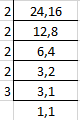Here LCM of 16 and 24 is 48

= (11 × 2)/ (24 × 2) + (3 × 3)/ (16 × 3)

By further calculation

= (22 + 9)/ 48

= 31/48

5. What rational number should be added to -3/5 to get 2?

Solution:

So the required rational number = 2 – (-3/5)

It can be written as

= 2 + 3/5

LCM of 1 and 5 is 5

= (2 × 5)/ (1 × 5) + (3 × 1)/ (5 × 1)

By further calculation

= (10 + 3)/ 5

So we get

= 13/5

= 2 3/5

6. What rational number should be subtracted from -5/12 to get 5/24?

Solution:

Required rational number = -5/12 – 5/24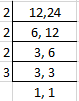Here the LCM of 12 and 24 is 72

= (-5 × 6)/ (12 × 6) – (5 × 3)/ (24 × 3)

By further calculation

= (-30 – 15)/ 72

So we get

= – 45/72

= -5/8

7. What rational number should be subtracted from 5/8 to get 8/5?

Solution:

Required rational number = 5/8 – 8/5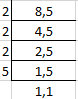Here LCM of 8 and 5 is 40

= (5 × 5)/ (8 × 5) – (8 × 8)/ (5 × 8)

By further calculation

= (25 – 64)/ 40

= -39/40

8. Evaluate:

(i) (7/8 × 24/21) + (-5/9 × 6/-25)

(ii) (8/15 × -25/16) + (-18/35 × 5/6)

(iii) (18/33 × -22/27) – (13/25 × -75/26)

(iv) (-13/7 × -35/39) – (-7/45 × 9/14)

Solution:

(i) (7/8 × 24/21) + (-5/9 × 6/-25)

It can be written as

= (7 × 24)/ (8 × 21) + (-5 × 6)/ (9 × -25)

By further simplification

= (1 × 3)/ (1 × 3) + (1 × 2)/ (3 × 5)

So we get

= 3/3 + 2/15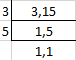Here LCM of 3 and 15 is 15

= (3 × 5)/ (3 × 5) + (2 × 1)/ (15 × 1)

By further calculation

= (15 + 2)/ 15

= 17/15

= 1 2/15

(ii) (8/15 × -25/16) + (-18/35 × 5/6)

It can be written as

= (8 × -25)/ (15 × 16) + (-18 × 5)/ (35 × 6)

By further calculation

= (1 × -5)/ (3 × 2) + (-3 × 1)/ (7 × 1)

So we get

= -5/6 – 3/7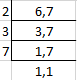Here LCM of 6 and 7 is 42

= (-5 × 7)/ (6 × 7) – (3 × 6)/ (7 × 6)

By further calculation

= (-35 – 18)/ 42

= -53/42

(iii) (18/33 × -22/27) – (13/25 × -75/26)

It can be written as

= (18 × -22)/ (33 × 27) – (13 × -75)/ (25 × 26)

By further calculation

= (2 × -2)/ (3 × 3) – (1 × -3)/ (1 × 2)

So we get

= -4/9 – (-3/2)

= -4/9 + 3/2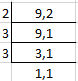Here LCM of 9 and 2 is 18

= (-4 × 2)/ (9 × 2) + (3 × 9)/ (2 × 9)

By further calculation

= (-8 + 27)/ 18

= 19/18

= 1 1/18

(iv) (-13/7 × -35/39) – (-7/45 × 9/14)

It can be written as

= (-13 × -35)/ (7 × 39) + (7 × 9)/ (45 × 14)

By further calculation

= (-1 × -5)/ (1 × 3) + (1 × 1)/ (5 × 2)

So we get

= 5/3 + 1/10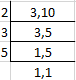Here the LCM of 3 and 10 is 30

= (5 × 10)/ (3 × 10) + (1 × 3)/ (10 × 3)

By further calculation

= (50 + 3)/ 30

= 53/30

= 1 23/30

9. The product of two rational numbers is 24. If one of them is -36/11, find the other.

Solution:

It is given that

Product of two rational numbers = 24

One rational number = -36/11

Other rational number = 24 ÷ (-36/ 11)

It can be written as

= 24 × (-11/36)

By further calculation

= 2 × (-11/3)

= -22/3

10. By what rational number should we multiply 20/-9, so that the product may be -5/9?

Solution:

Here the required rational number = -5/9 ÷ (20/-9)

By further calculation

= -5/9 × (-9/20)

= 1/4